# Modular Oriclip

One of my interests involves building binder clip sculptures. The name oriclip is inspired by origami, which stands for ori “fold” and kami “paper”. Sometimes, binder clips are called foldover clip or foldback clip.

Fast scroll to

## Special case

### 2-clip constructions

#### 2-ftf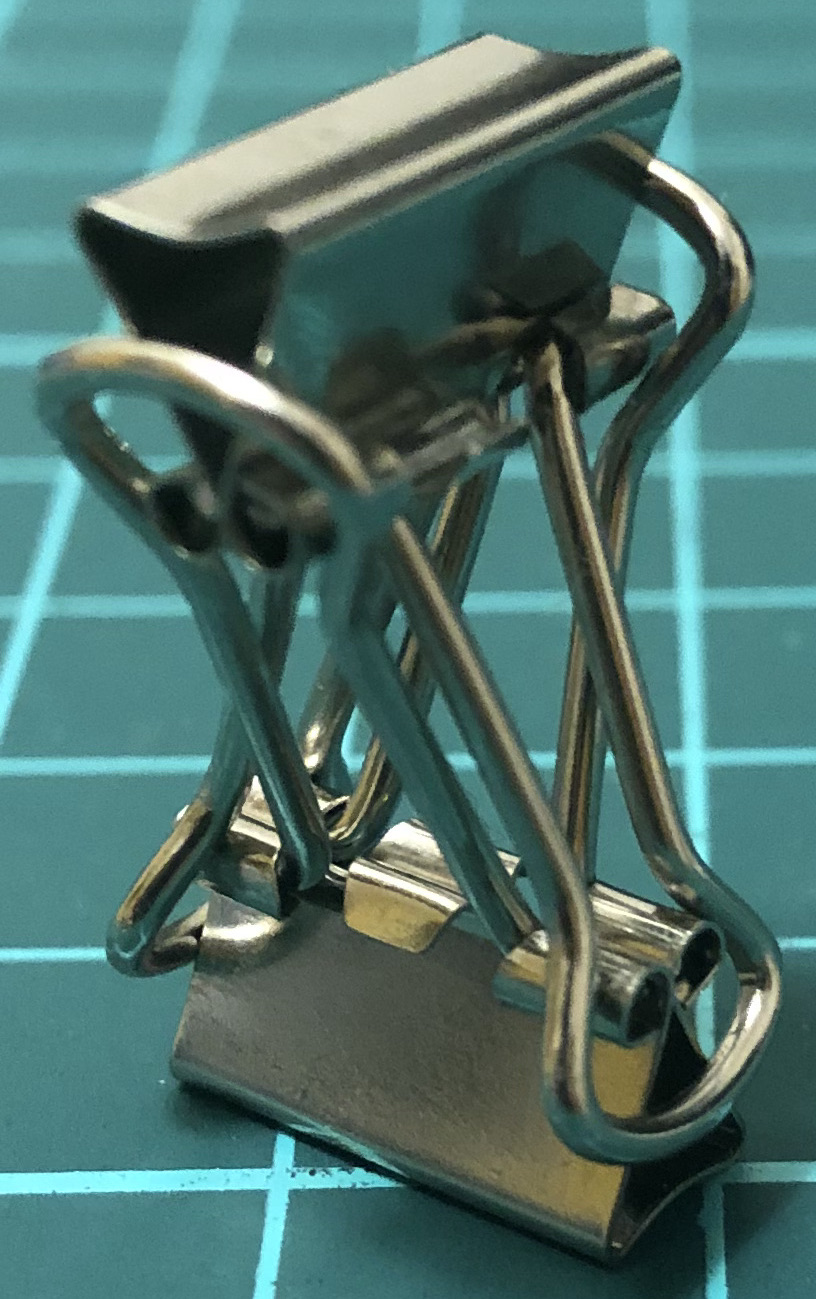↑ # Clips = 2

#### 2-btb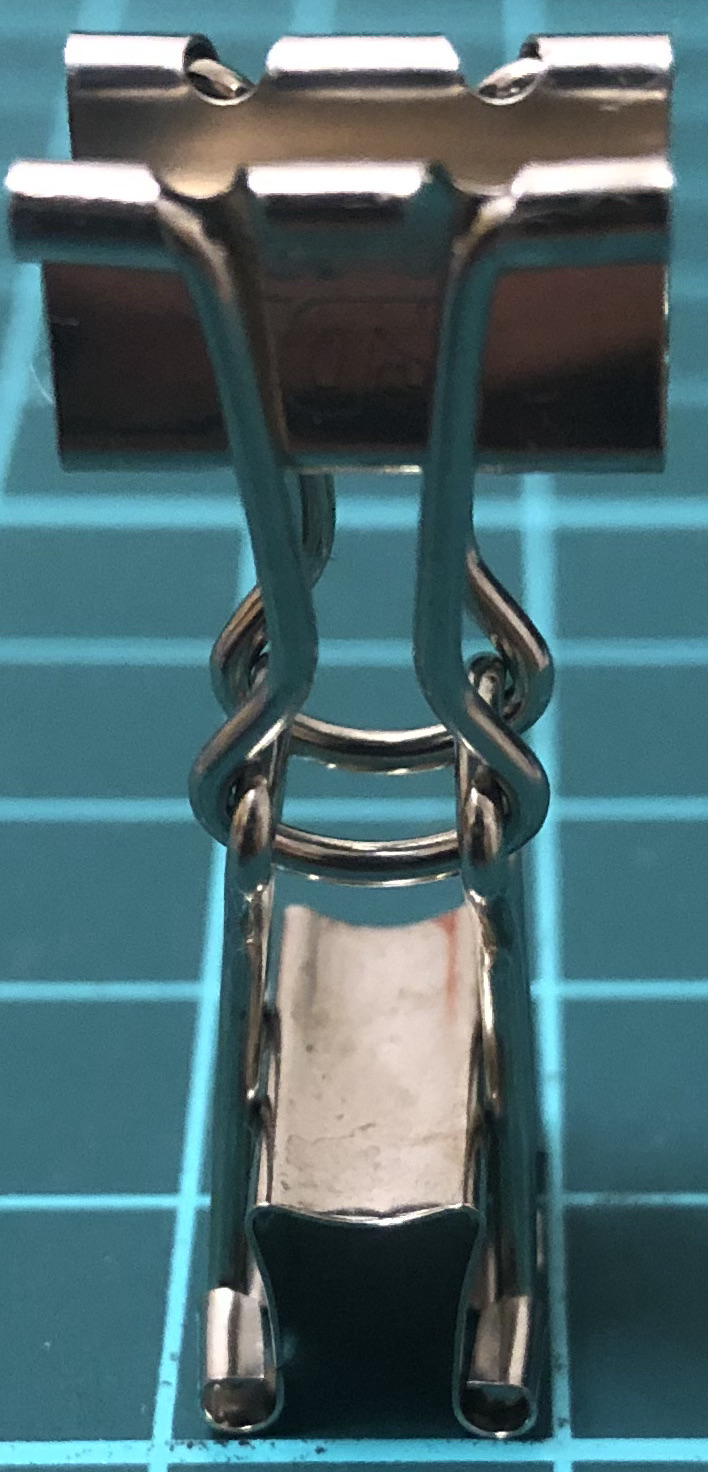↑ # Clips = 2

#### 2-hth↑ # Clips = 2

### 6-clip constructions

#### 6-cycle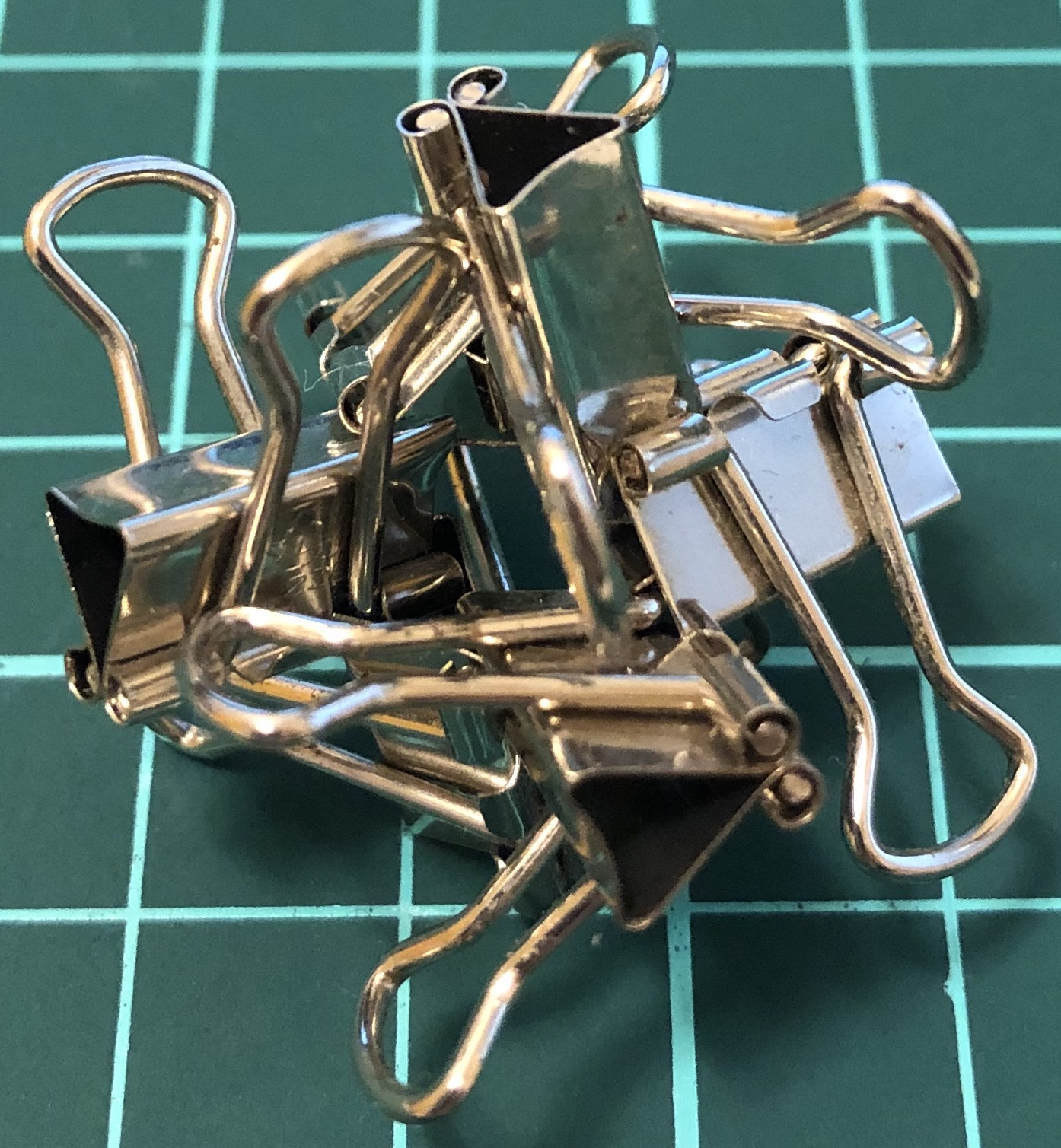↑ # Clips = 6
↑ Base = triangular antiprism
↑ Symmetry = triangular antiprism’s rotations

#### 6-dense↑ # Clips = 6
↑ Base = six-piece burr
↑ Symmetry = triangular antiprism’s rotations

#### 6-wedge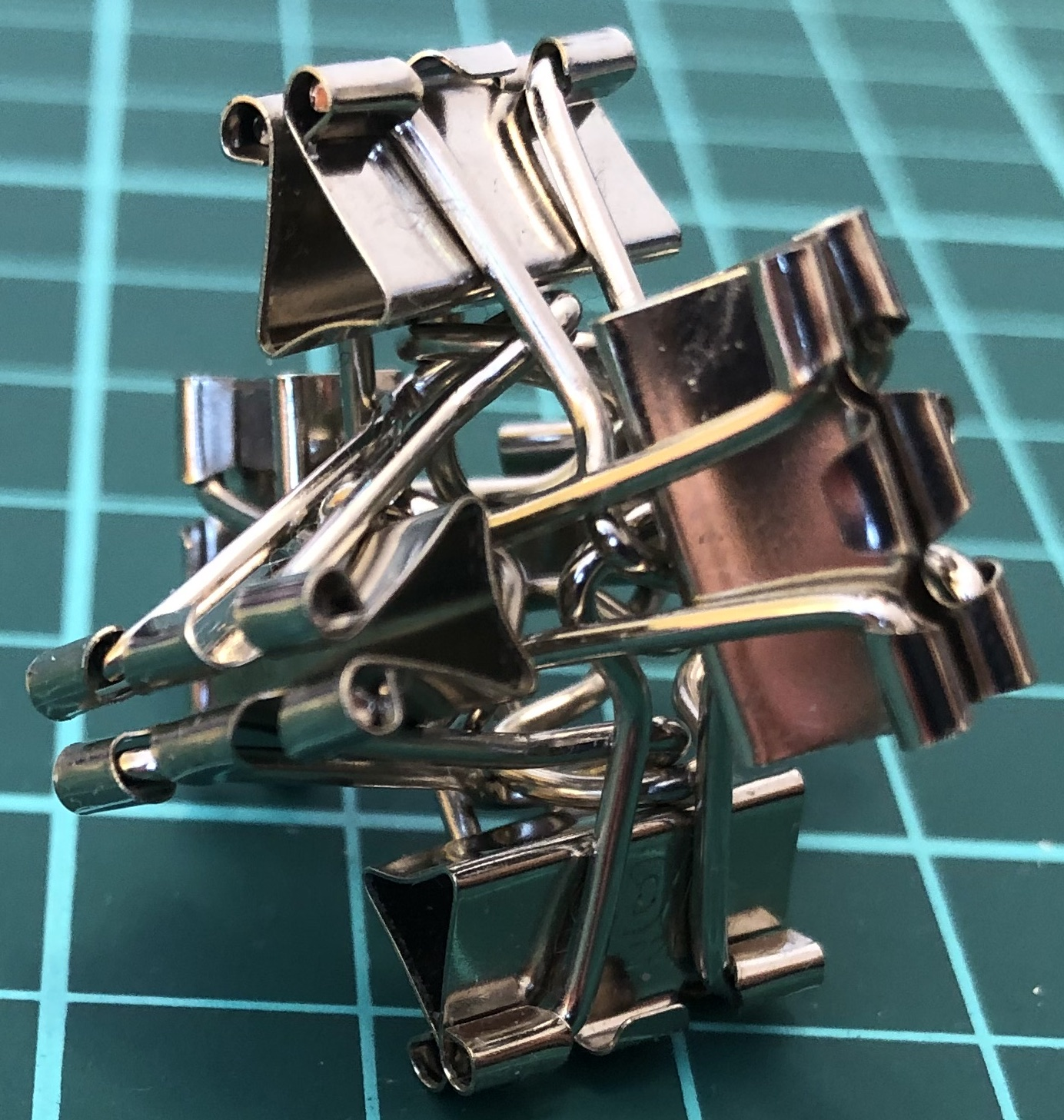↑ # Clips = 6
↑ Base = six-piece burr
↑ Symmetry = tetrahedron’s rotations

#### 6-fitin↑ # Clips = 6
↑ Base = octahedron
↑ Symmetry = tetrahedron’s rotations

#### 6-twist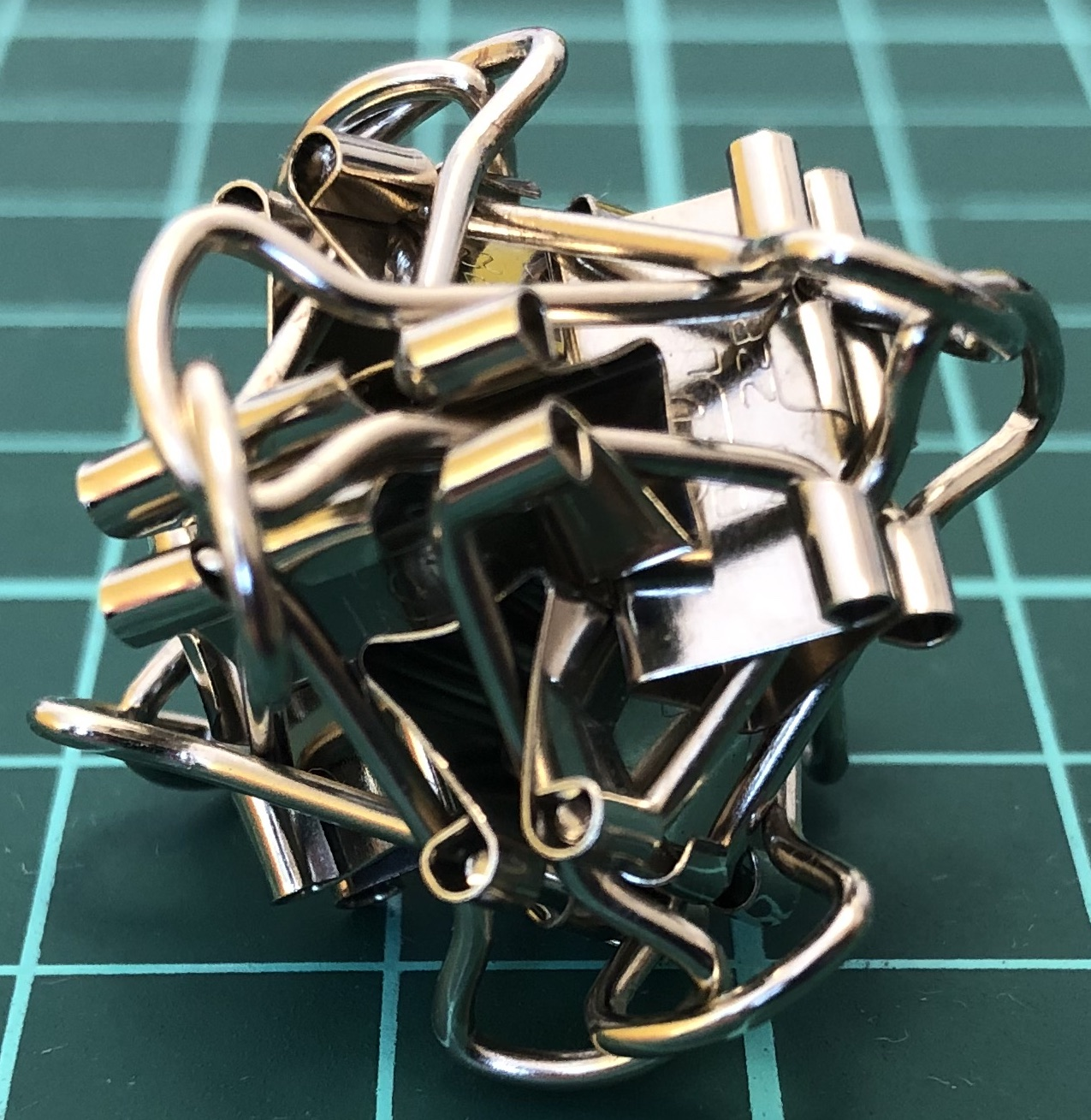↑ # Clips = 6
↑ Base = octahedron
↑ Symmetry = tetrahedron’s rotations

#### 6-cross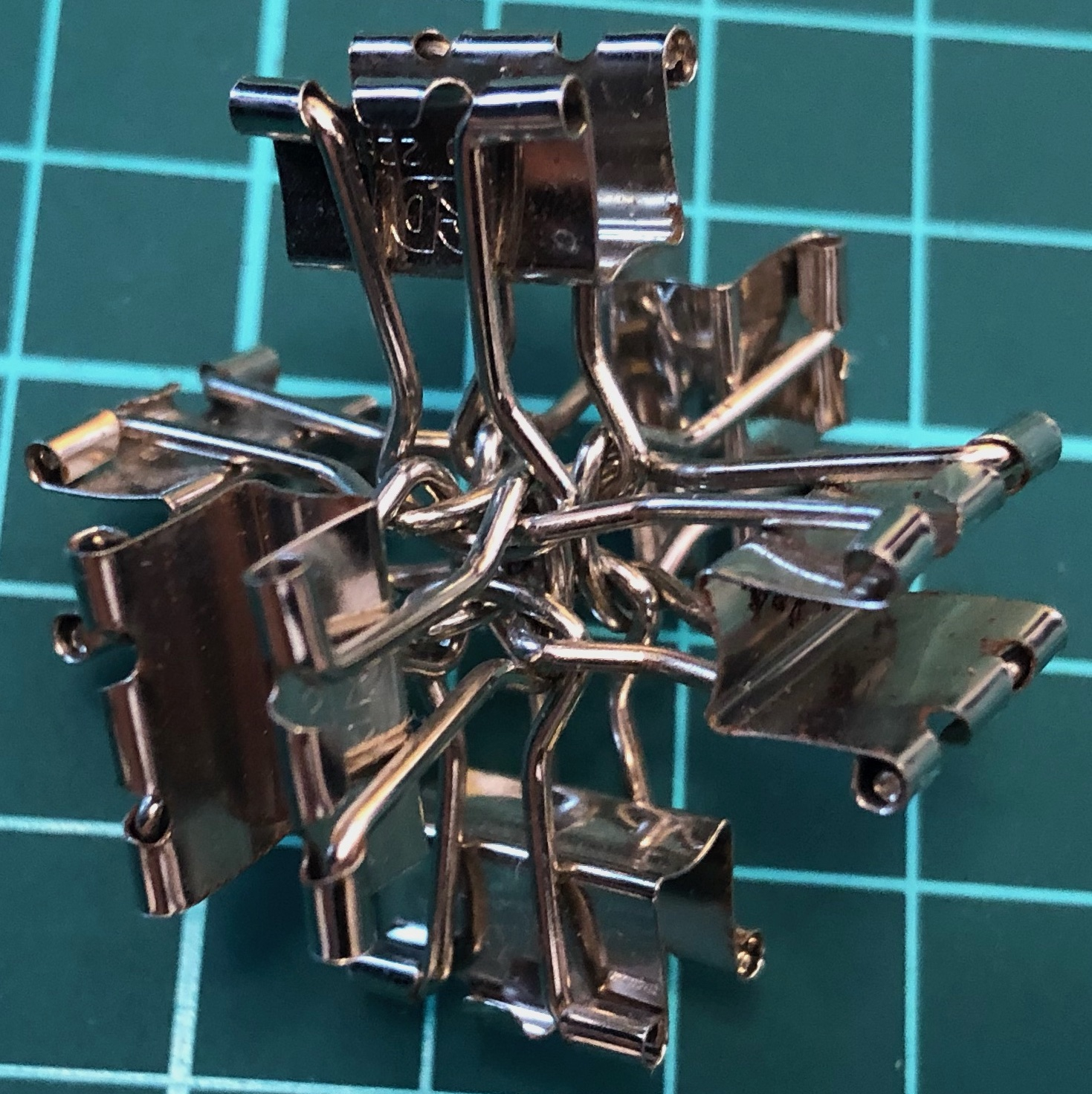↑ # Clips = 6
↑ Base = three-piece burr
↑ Symmetry = pyritohedron’s rotations and reflections

#### 6-spike↑ # Clips = 6
↑ Base = six-piece burr
↑ Symmetry = pyritohedron’s rotations and reflections

#### 6-stand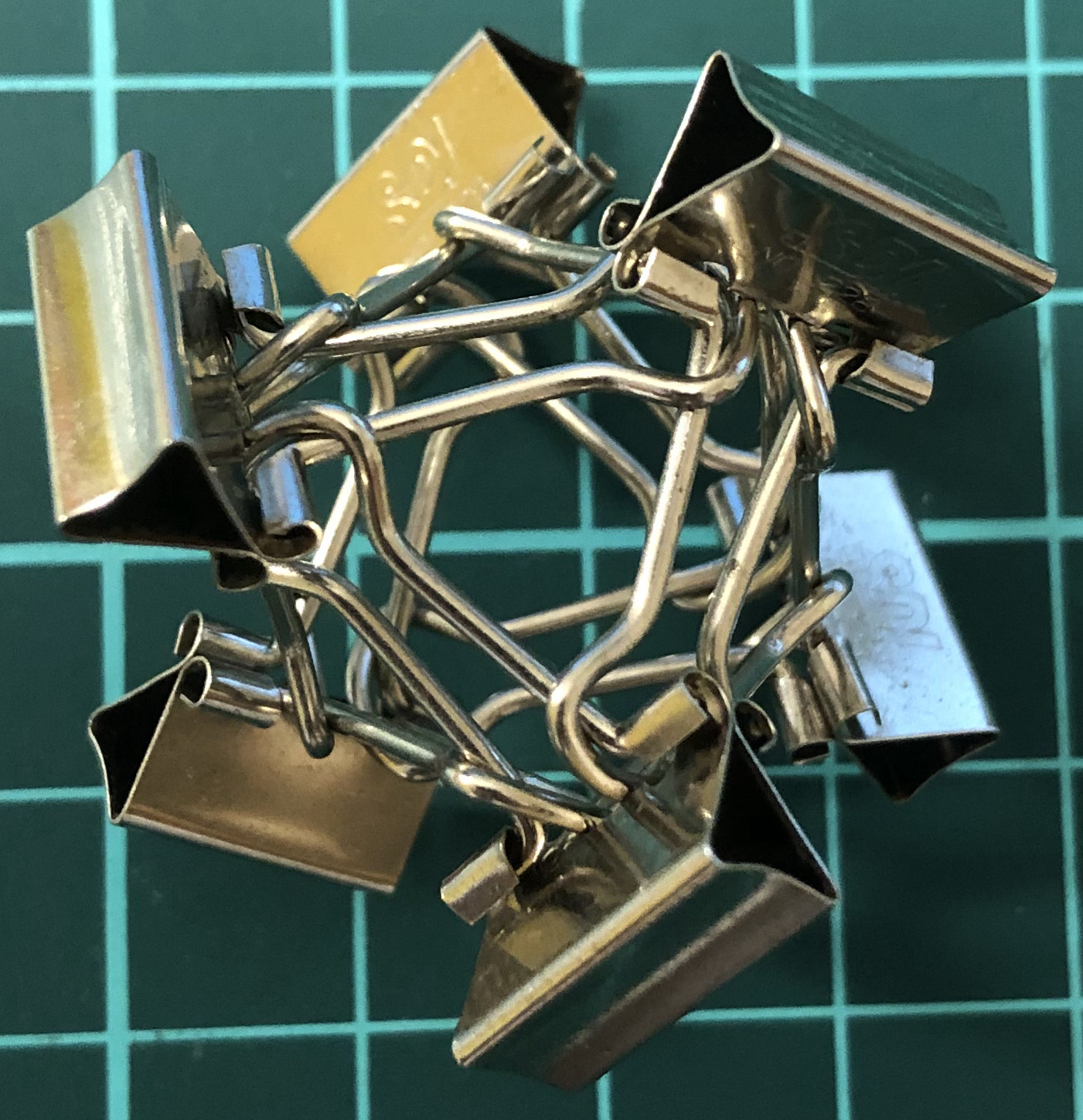↑ # Clips = 6
↑ Base = octahedron
↑ Symmetry = pyritohedron’s rotations and reflections

### 12-clip constructions

#### 12-angel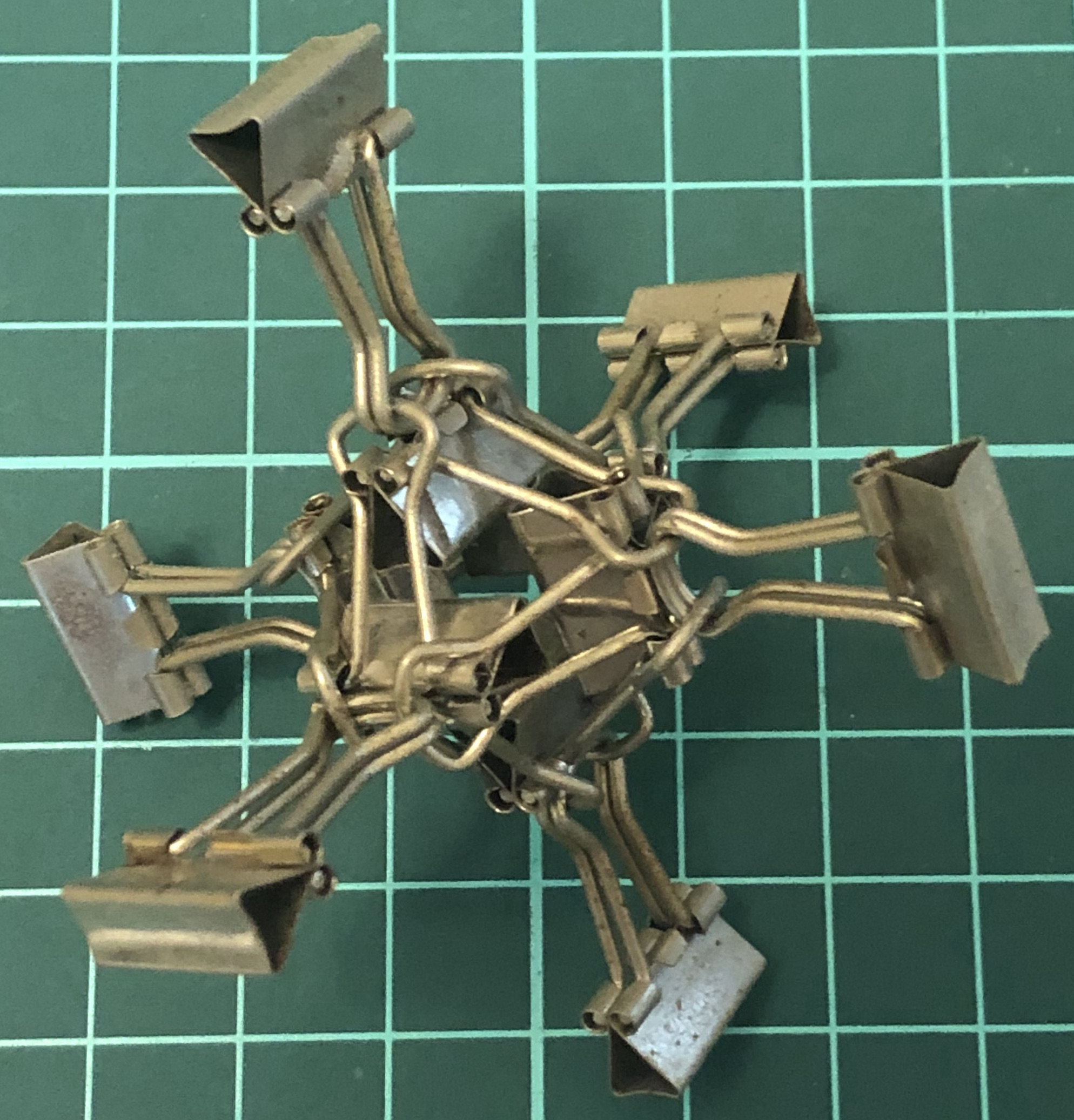↑ # Clips = 12
↑ Base = octahedron
↑ Symmetry = pyritohedron’s rotations and reflections

#### Q12-aC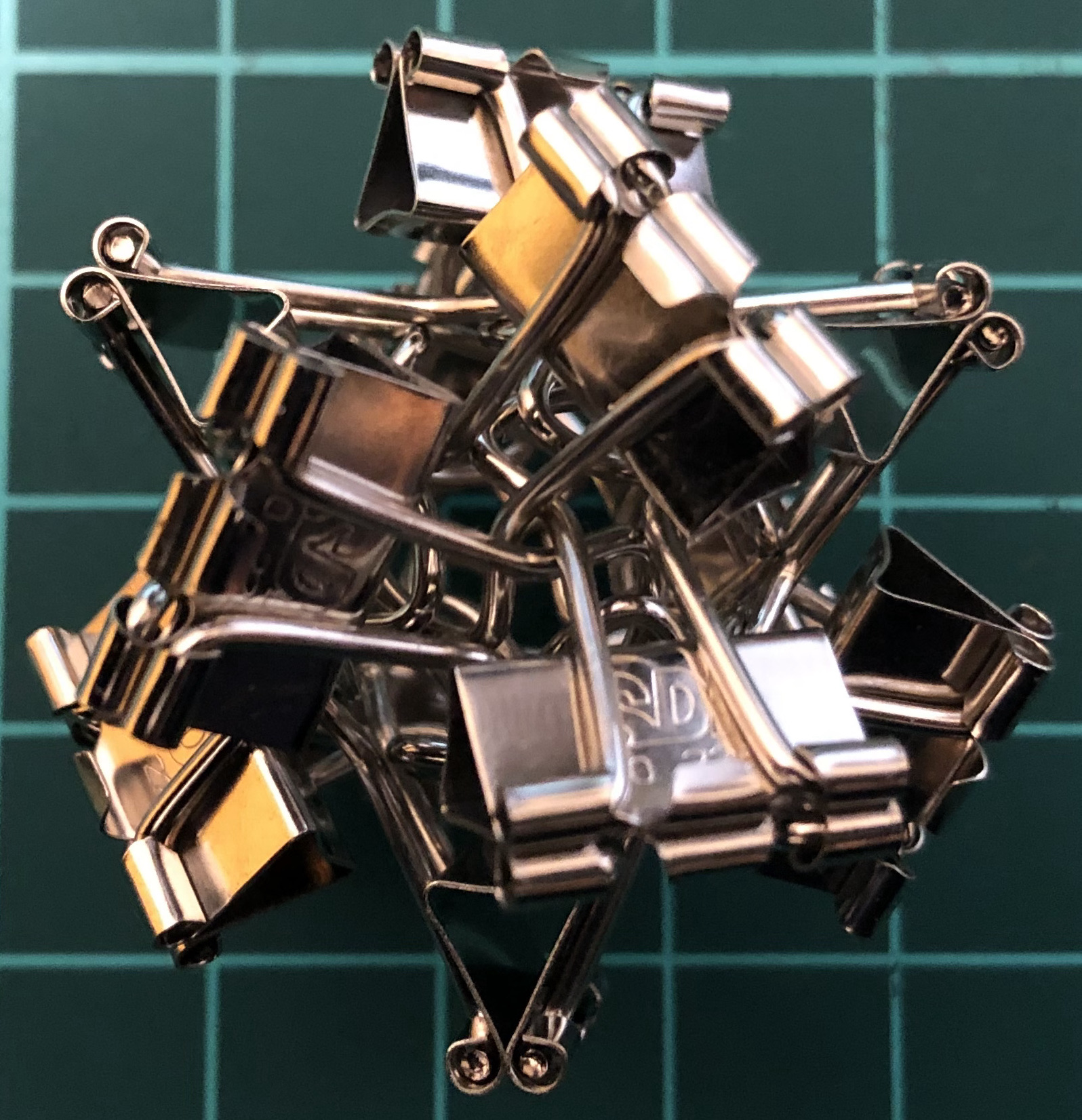↑ # Clips = 12
↑ Base = cuboctahedron

### S-series

One clip = one vertex. One handle = one edge.

#### S12-aC↑ # Clips = 12
↑ Base = cuboctahedron
↑ Symmetry = cube’s rotations↑ # Clips = 30
↑ Base = icosidodecahedron
↑ Symmetry = dodecahedron’s rotations

### A-series

One clip = one edge.

#### A12-O↑ # Clips = 12
↑ Base = octahedron
↑ Symmetry = pyritohedron’s rotations and reflections

#### A24-aC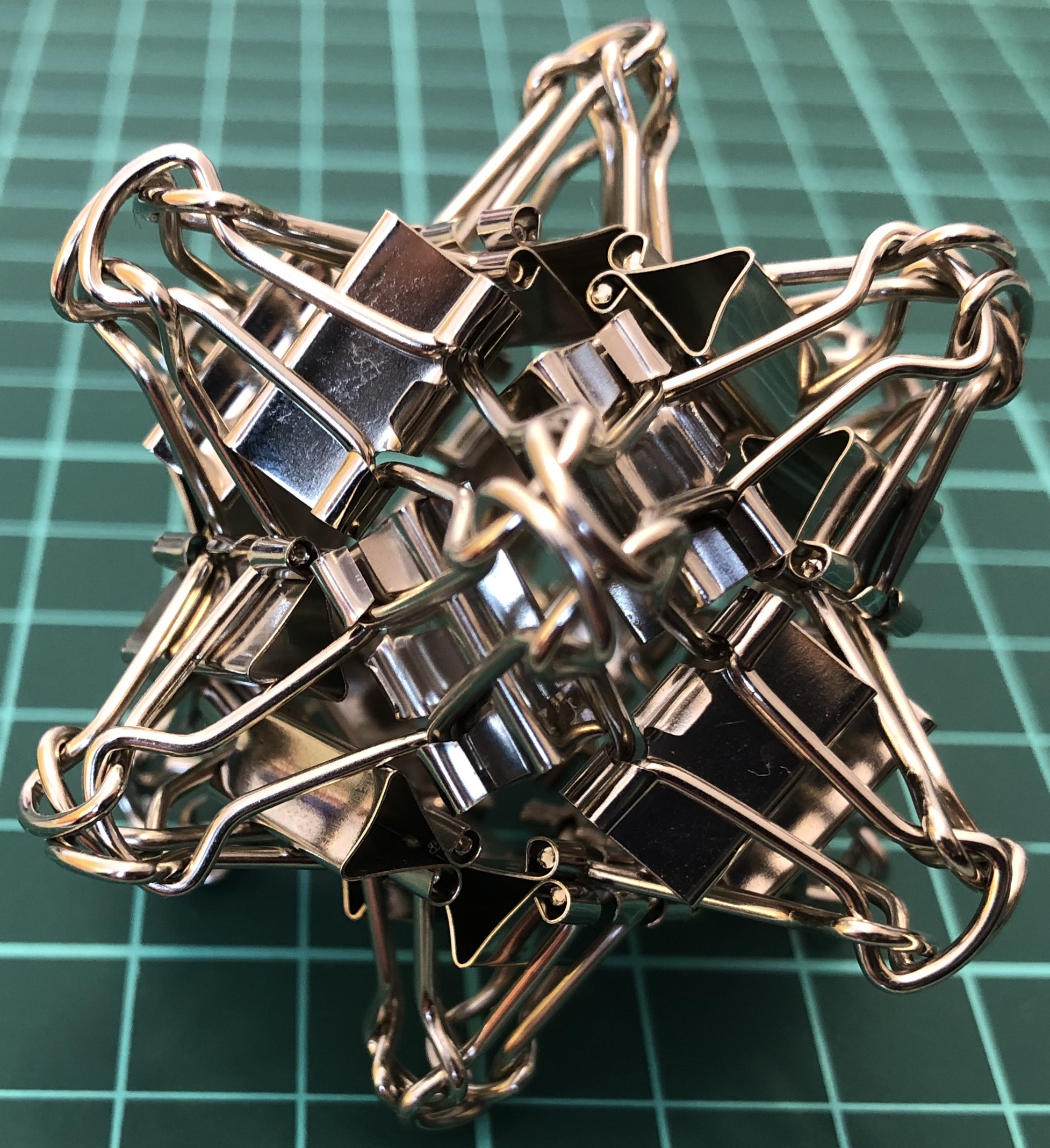↑ # Clips = 24
↑ Base = cuboctahedron
↑ Symmetry = cube’s rotations

#### A36-kC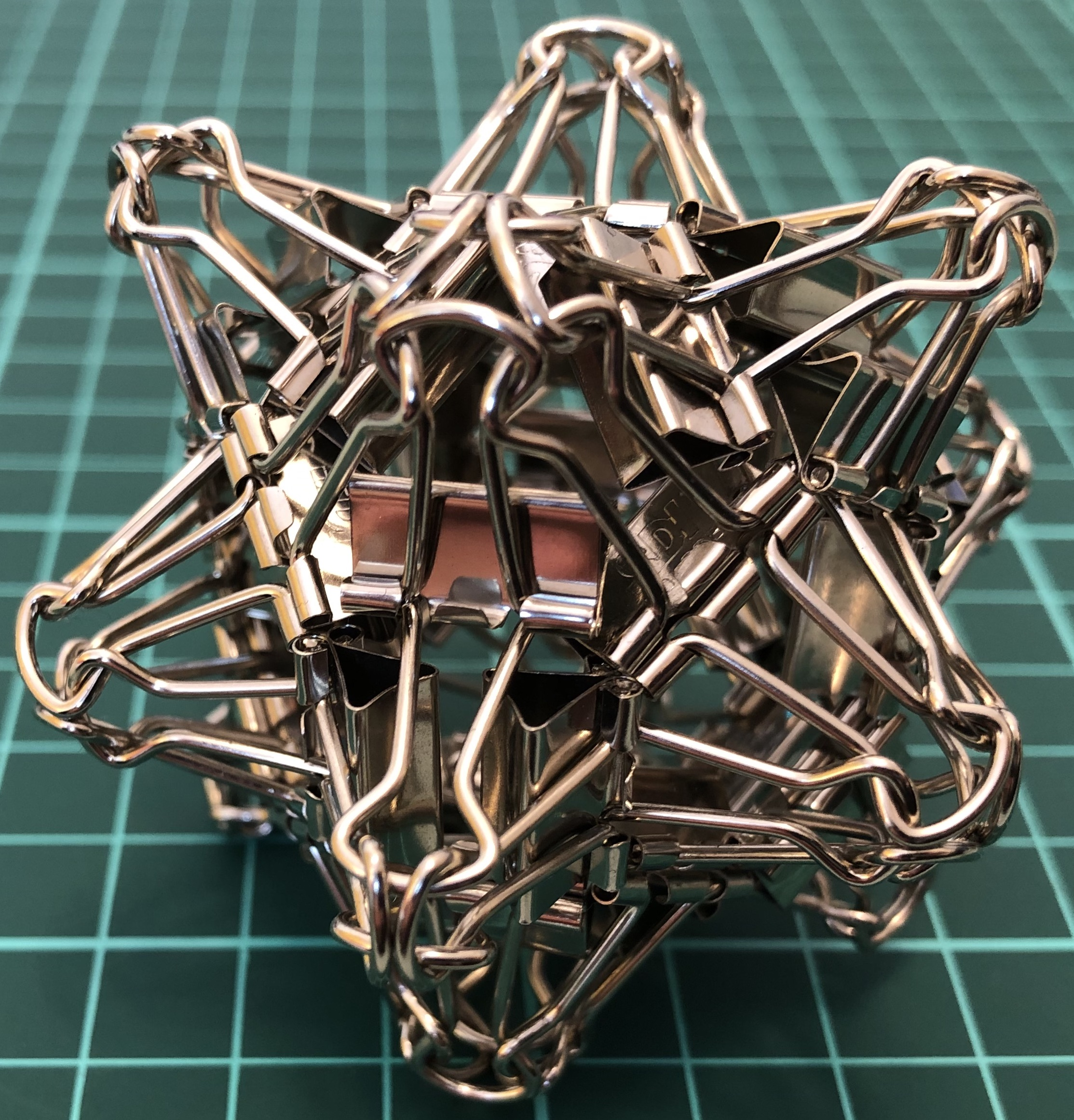↑ # Clips = 36
↑ Base = tetrakis hexahedron
↑ Symmetry = pyritohedron’s rotations and reflections

#### A48-aaC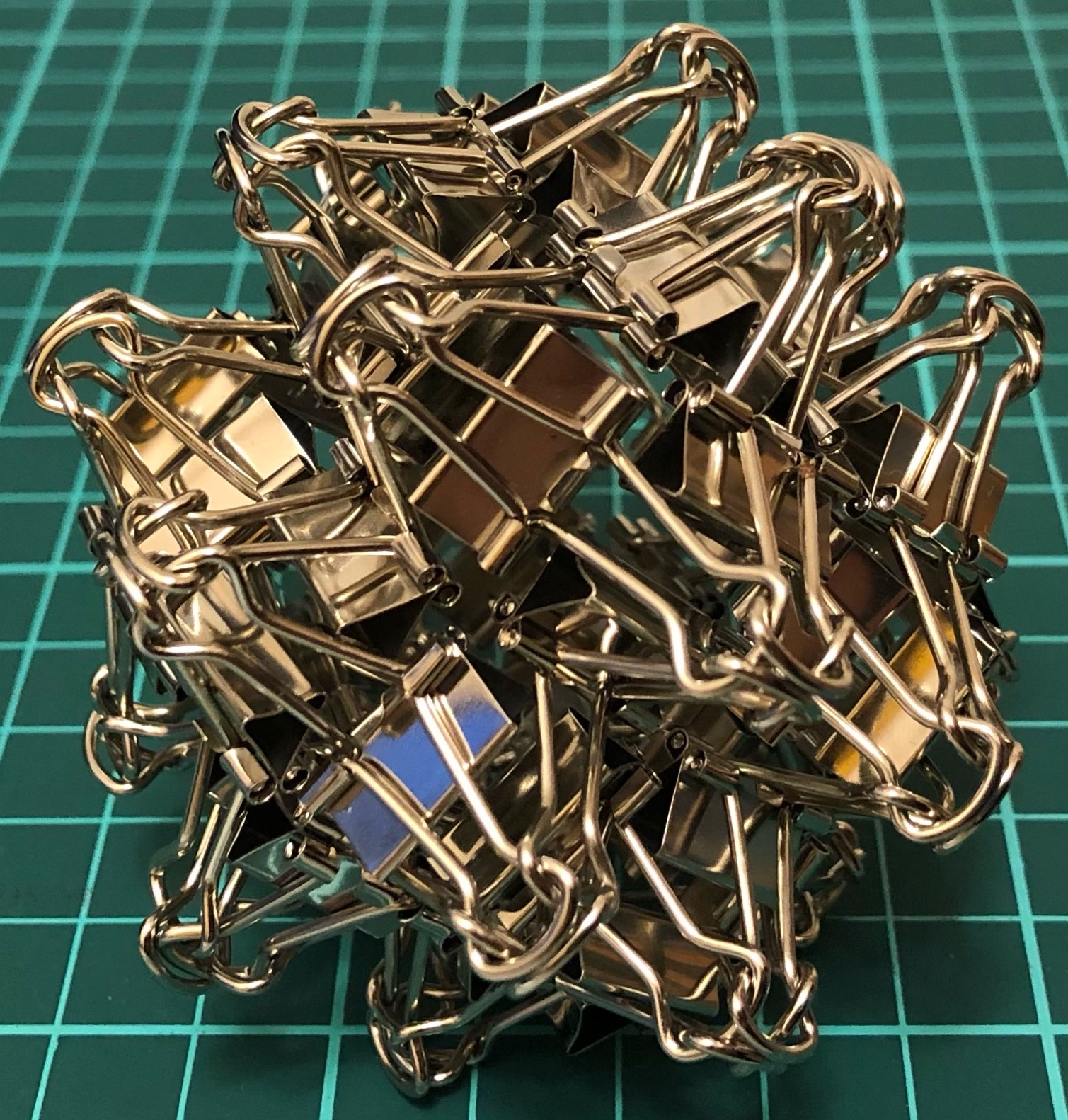↑ # Clips = 48
↑ Base = rhombicuboctahedron
↑ Symmetry = cube’s rotations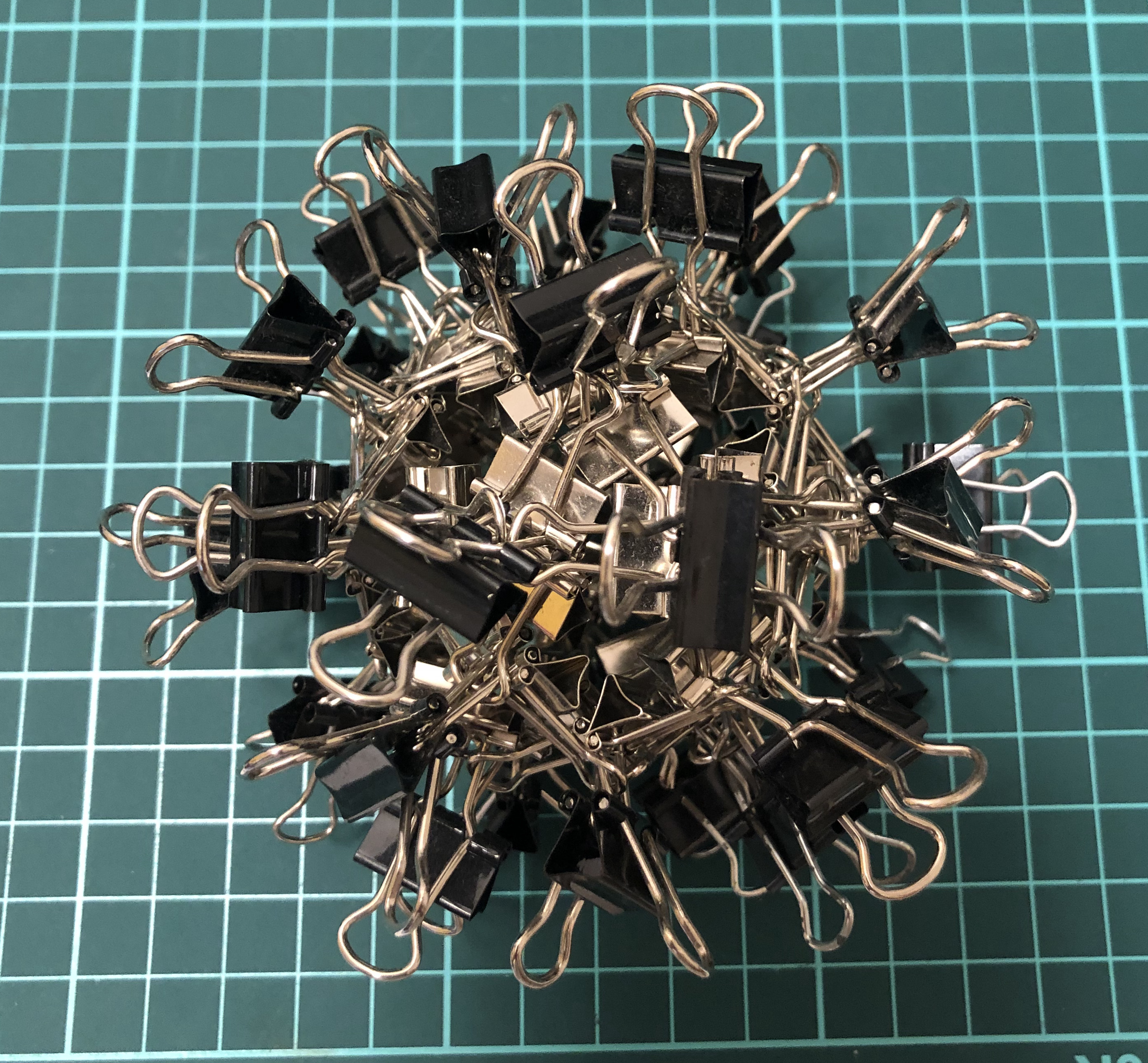↑ # Clips = 60
↑ Base = icosidodecahedron
↑ Symmetry = dodecahedron’s rotations

#### A12-O8-C↑ # Clips = 96
↑ Local base = octahedron
↑ Global base = cube
↑ Symmetry = cube’s rotations

#### A24-aC4-T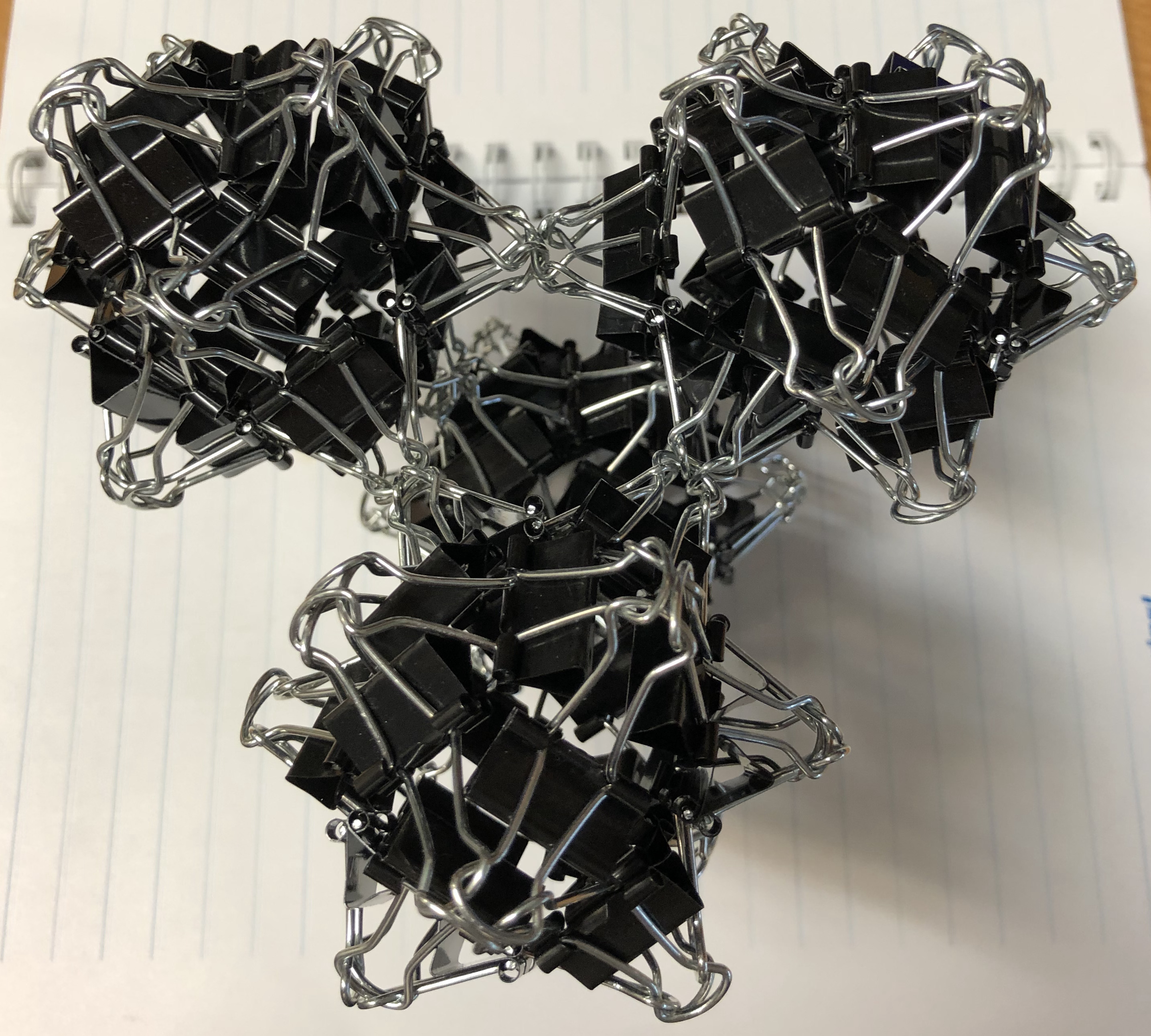↑ # Clips = 96
↑ Local base = cuboctahedron
↑ Global base = tetrahedron
↑ Symmetry = tetrahedron’s rotations

#### A24-aC8-O↑ # Clips = 144
↑ Local base = cuboctahedron
↑ Global base = octahedron
↑ Symmetry = cube’s rotations

## Vertex unit

### Η-series

Four clips = one Η-vertex = one vertex.

#### Η24-O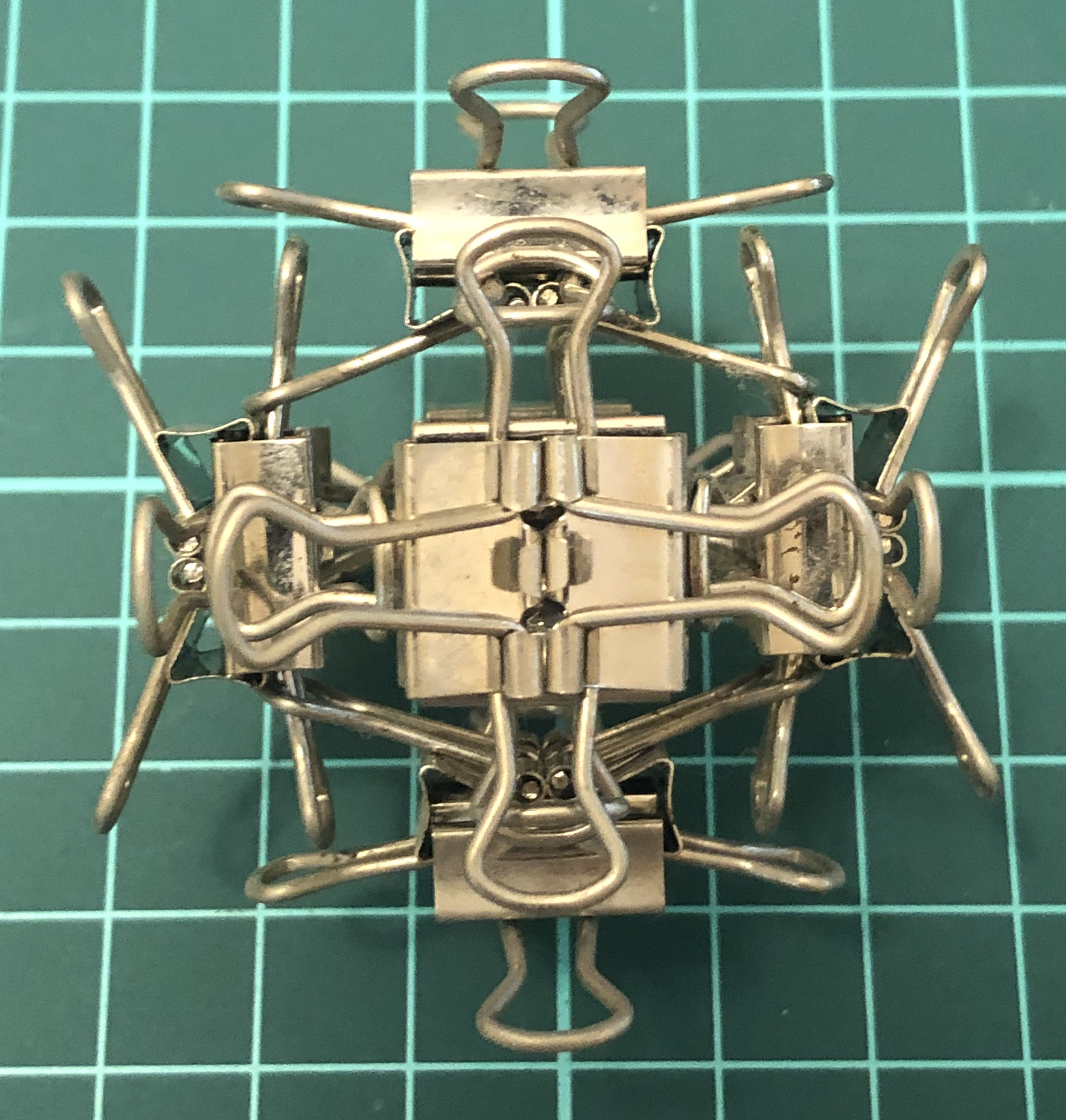↑ # Clips = 24
↑ Base = octahedron
↑ Vertex config = 3.3.3.3
↑ Symmetry = pyritohedron’s rotations and reflections

#### Η48-jC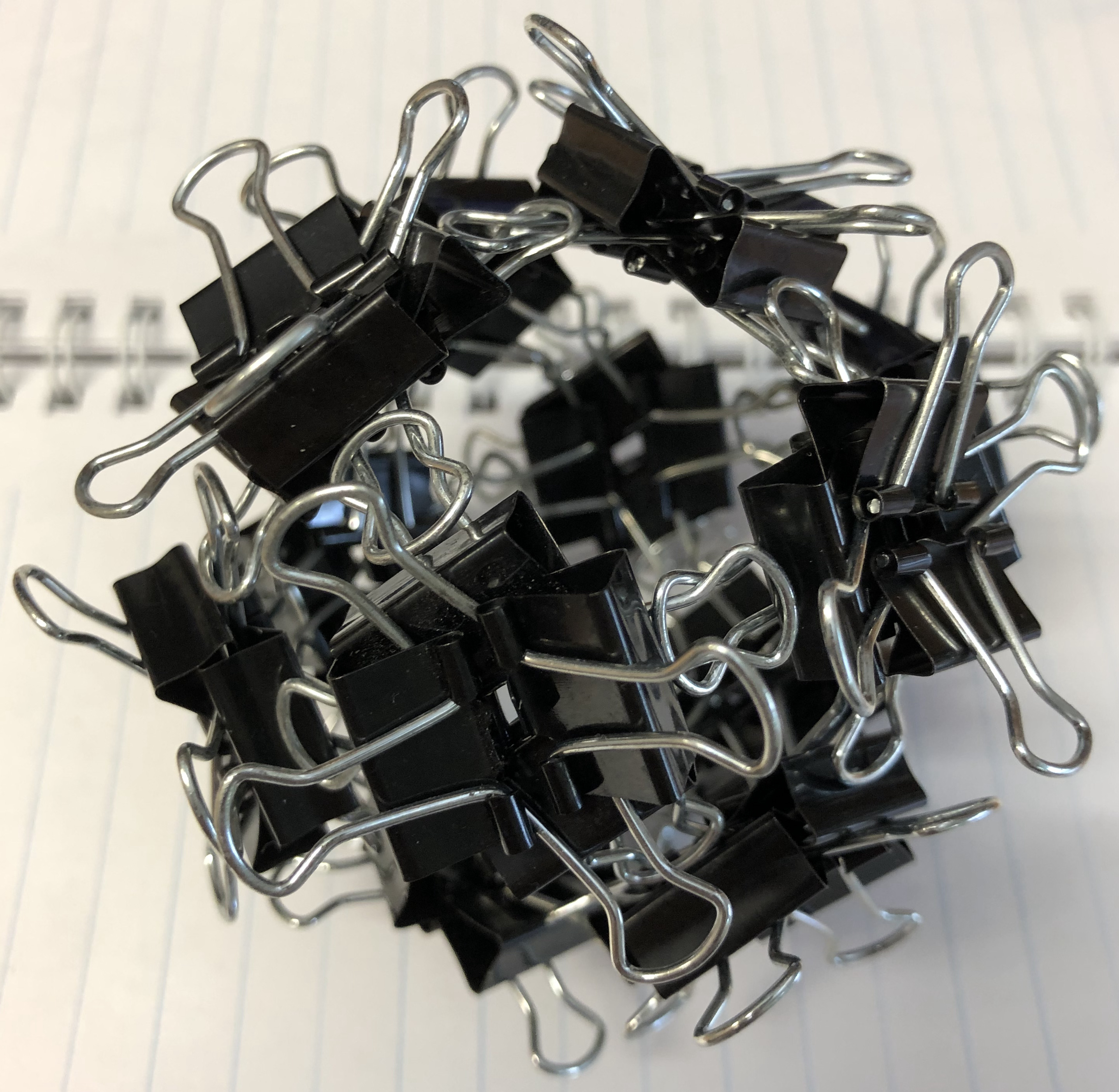↑ # Clips = 48
↑ Base = rhombic dodecahedron
↑ Face config = 3.4.3.4
↑ Symmetry = cube’s rotations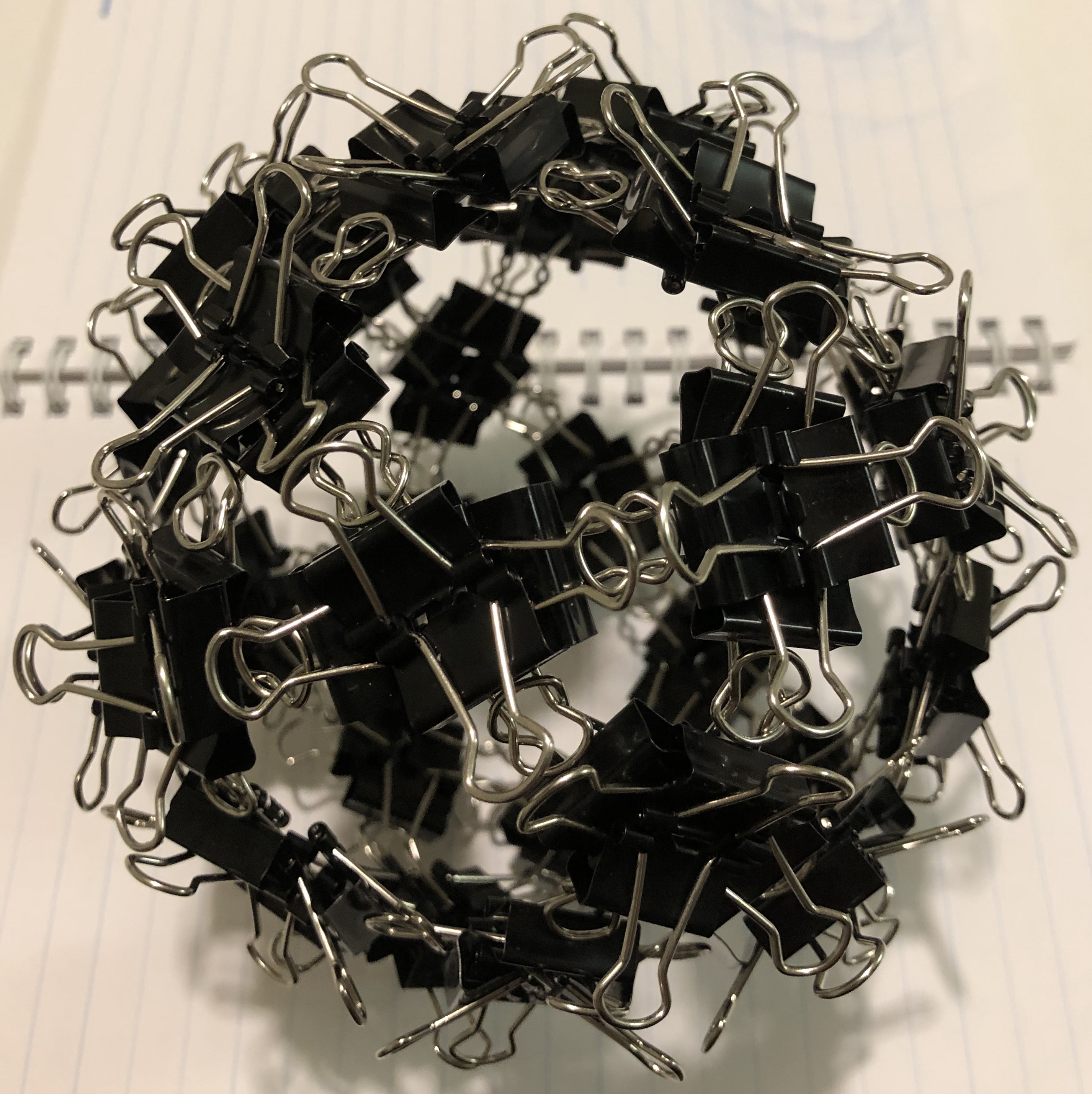↑ # Clips = 120
↑ Base = icosidodecahedron ↑ Symmetry = dodecahedron’s rotations

#### Η120-lC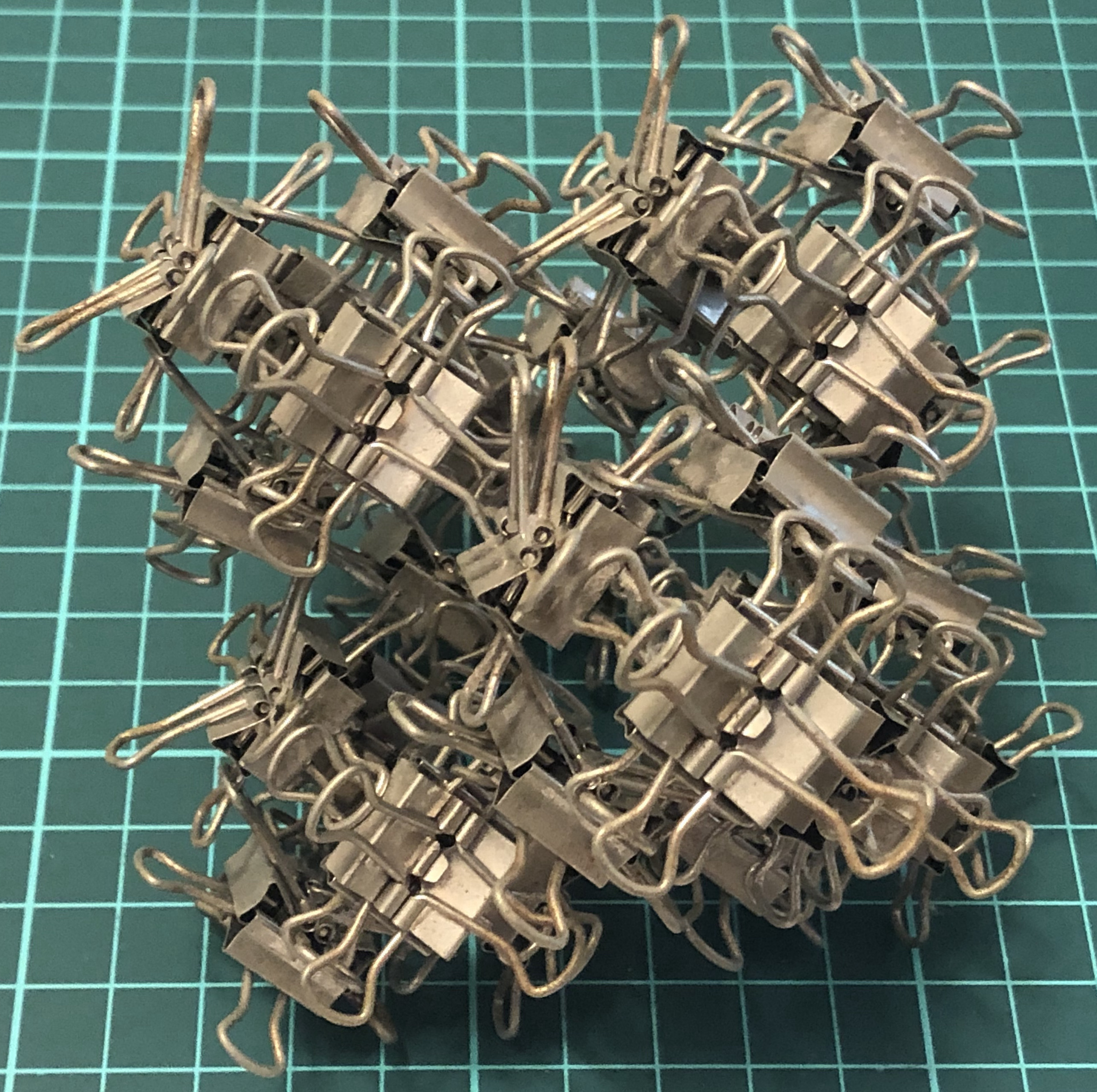↑ # Clips = 120
↑ Base = application of the loft operation upon a cube
↑ Symmetry = cube’s rotations

#### Η24-T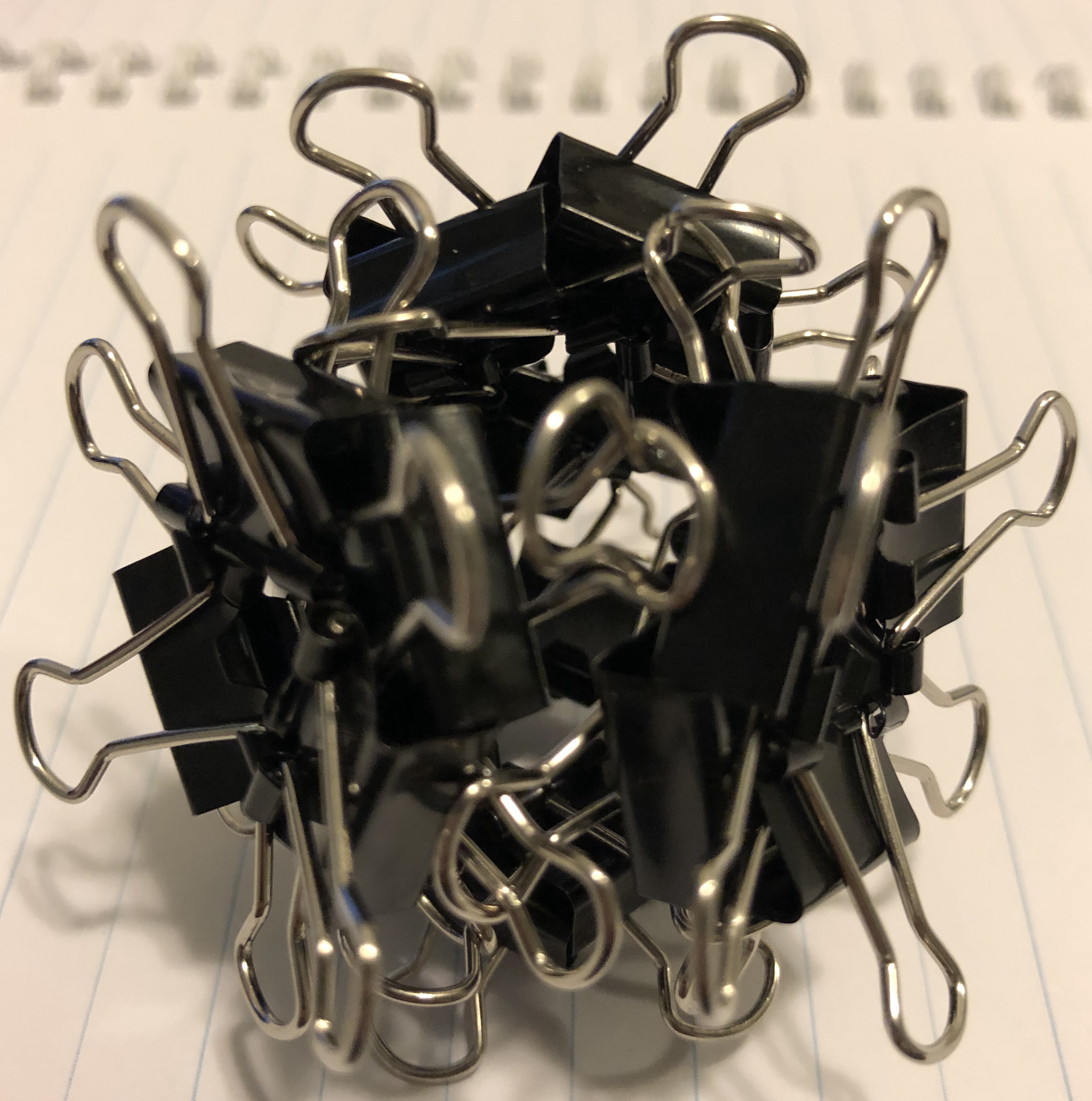↑ # Clips = 24
↑ Base = tetrahedron
↑ Vertex config = 3.3.3
↑ Symmetry = tetrahedron’s rotations

#### Η48-O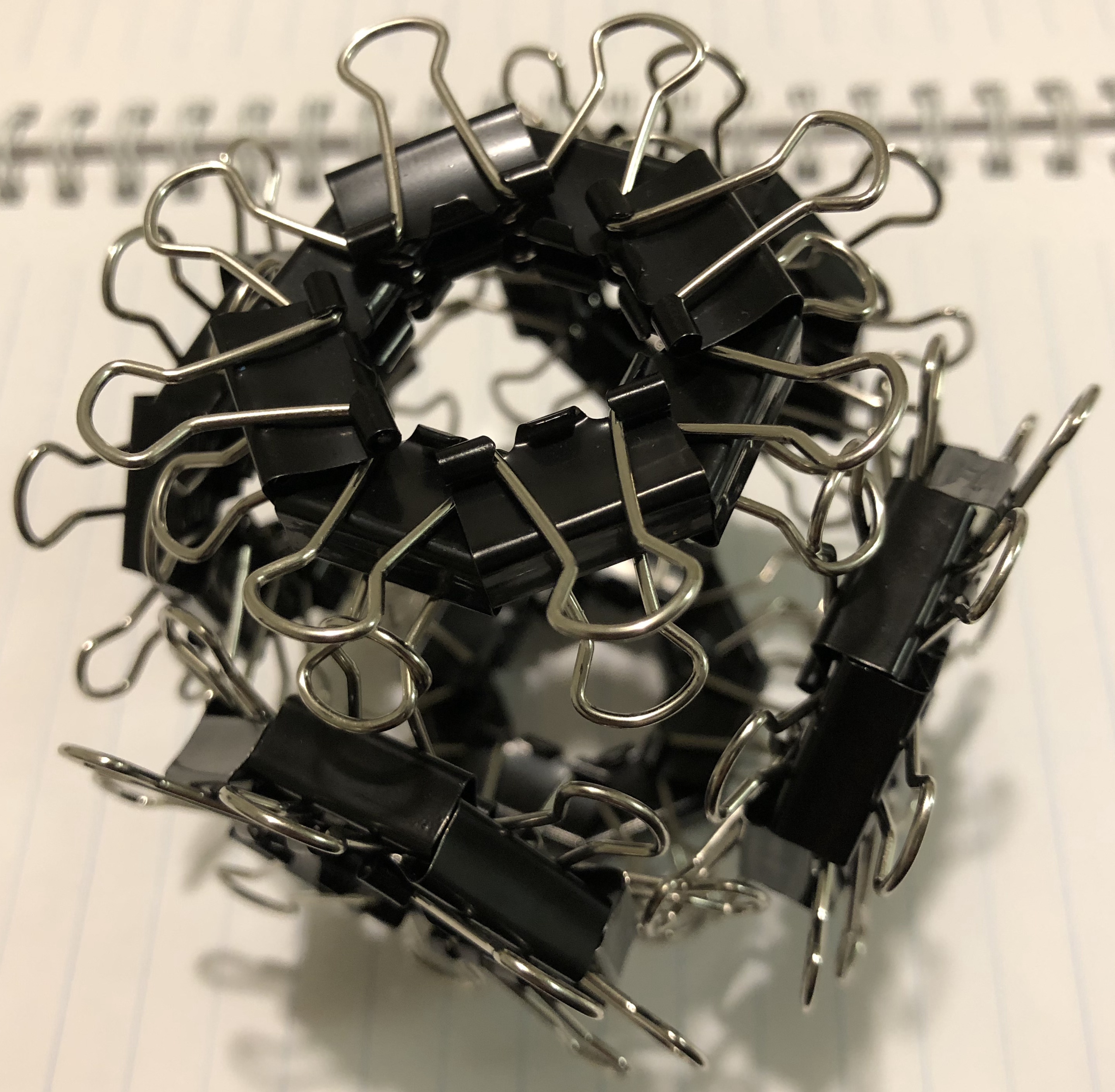↑ # Clips = 48
↑ Base = octahedron
↑ Vertex config = 3.3.3.3
↑ Symmetry = pyritohedron’s rotations and reflections

#### Η48-C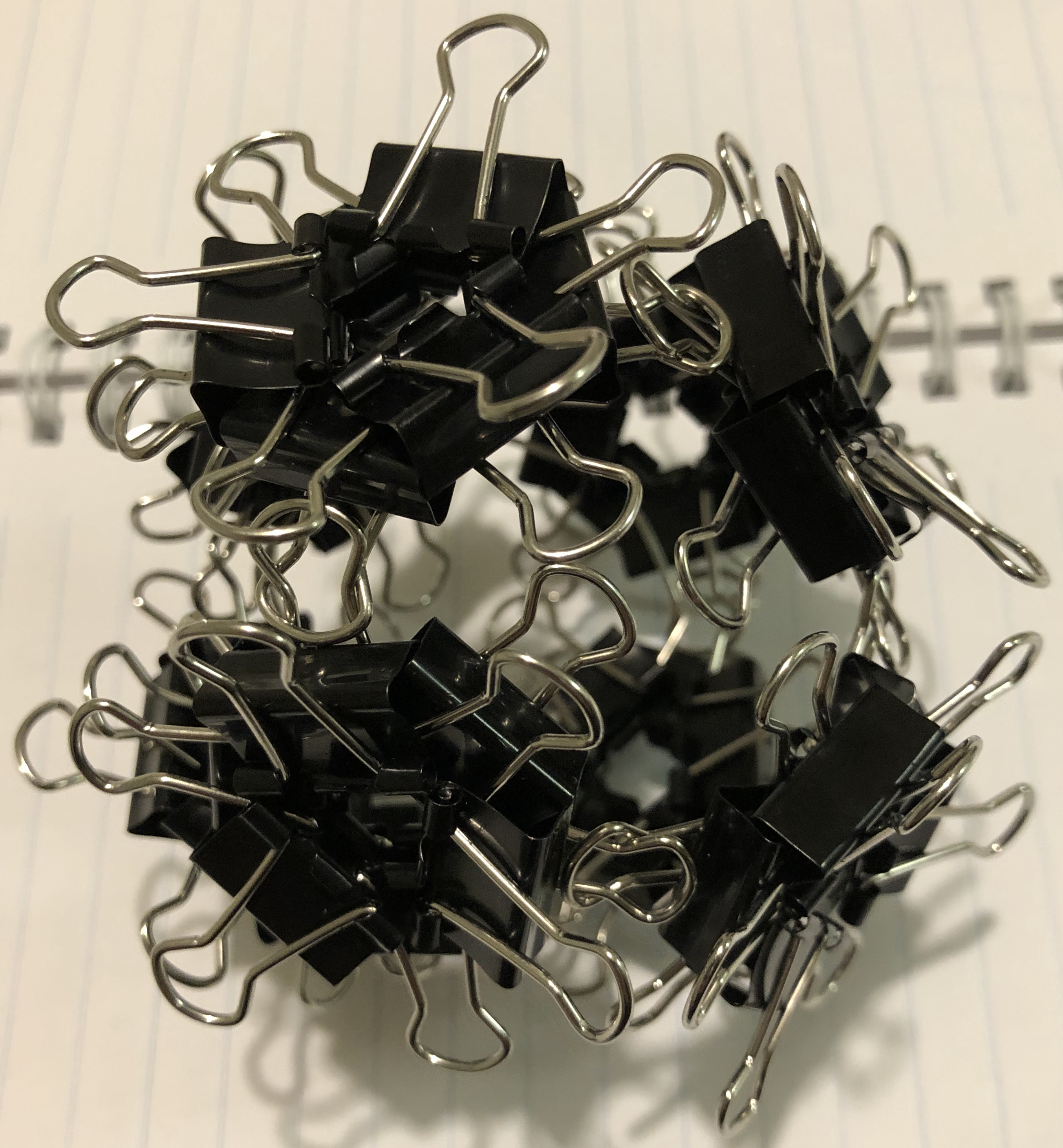↑ # Clips = 48
↑ Base = cube
↑ Vertex config = 4.4.4
↑ Symmetry = tetrahedron’s rotations

#### H48-O

This “H” is the Latin Ech because it is used as an edge unit. The other “Η” are Greek Eta because they are used as vertex unit.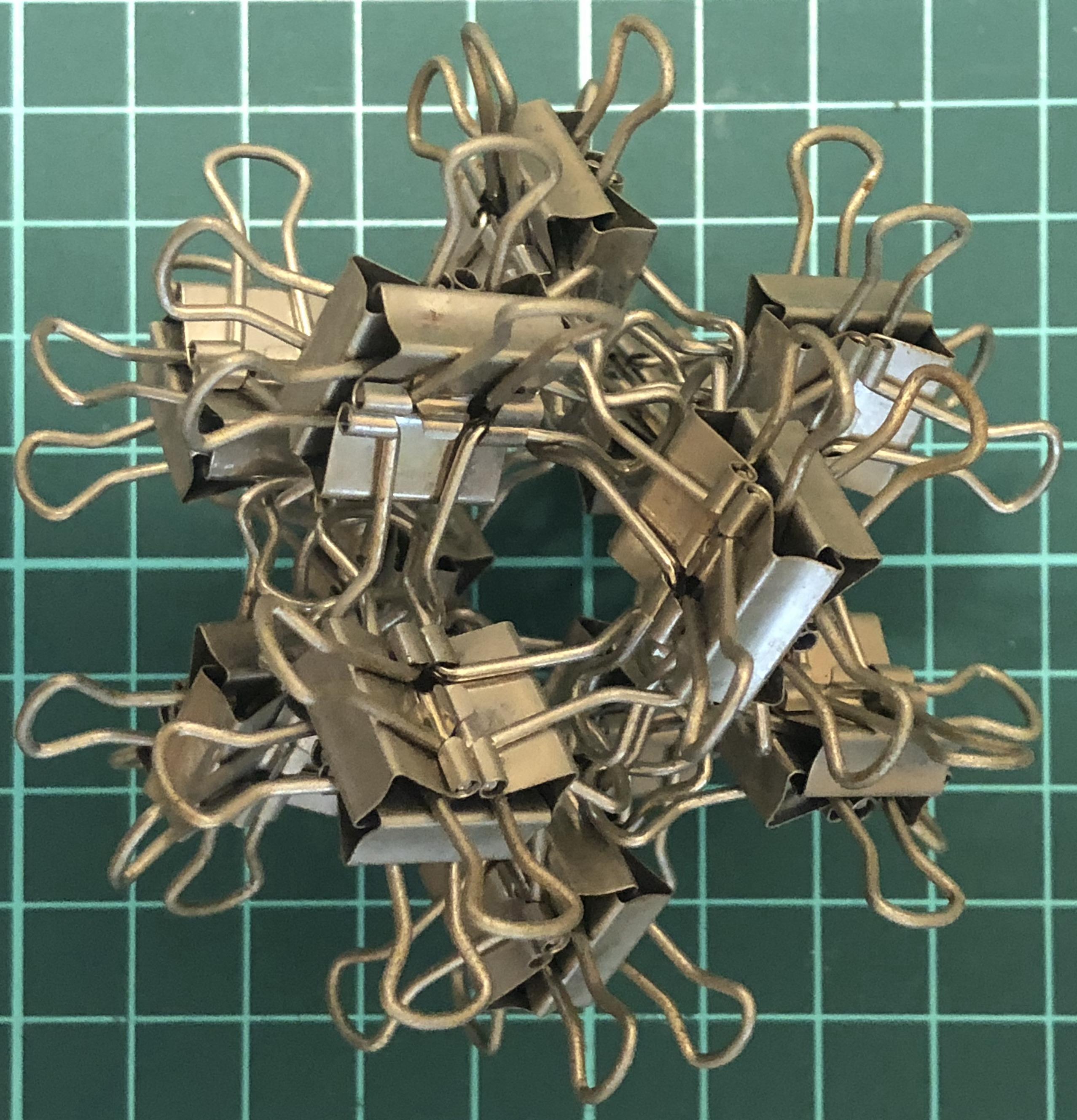↑ # Clips = 48
↑ Base = octahedron
↑ Vertex config = 3.3.3.3
↑ Symmetry = cube’s rotations

#### H120-D

This “H” is the Latin Ech because it is used as an edge unit. The other “Η” are Greek Eta because they are used as vertex unit.↑ # Clips = 120
↑ Base = dodecahedron
↑ Vertex config = 5.5.5
↑ Symmetry = dodecahedron’s rotations

### Φ-series

Three clips = one Φ-vertex = one vertex.

#### Φ12-T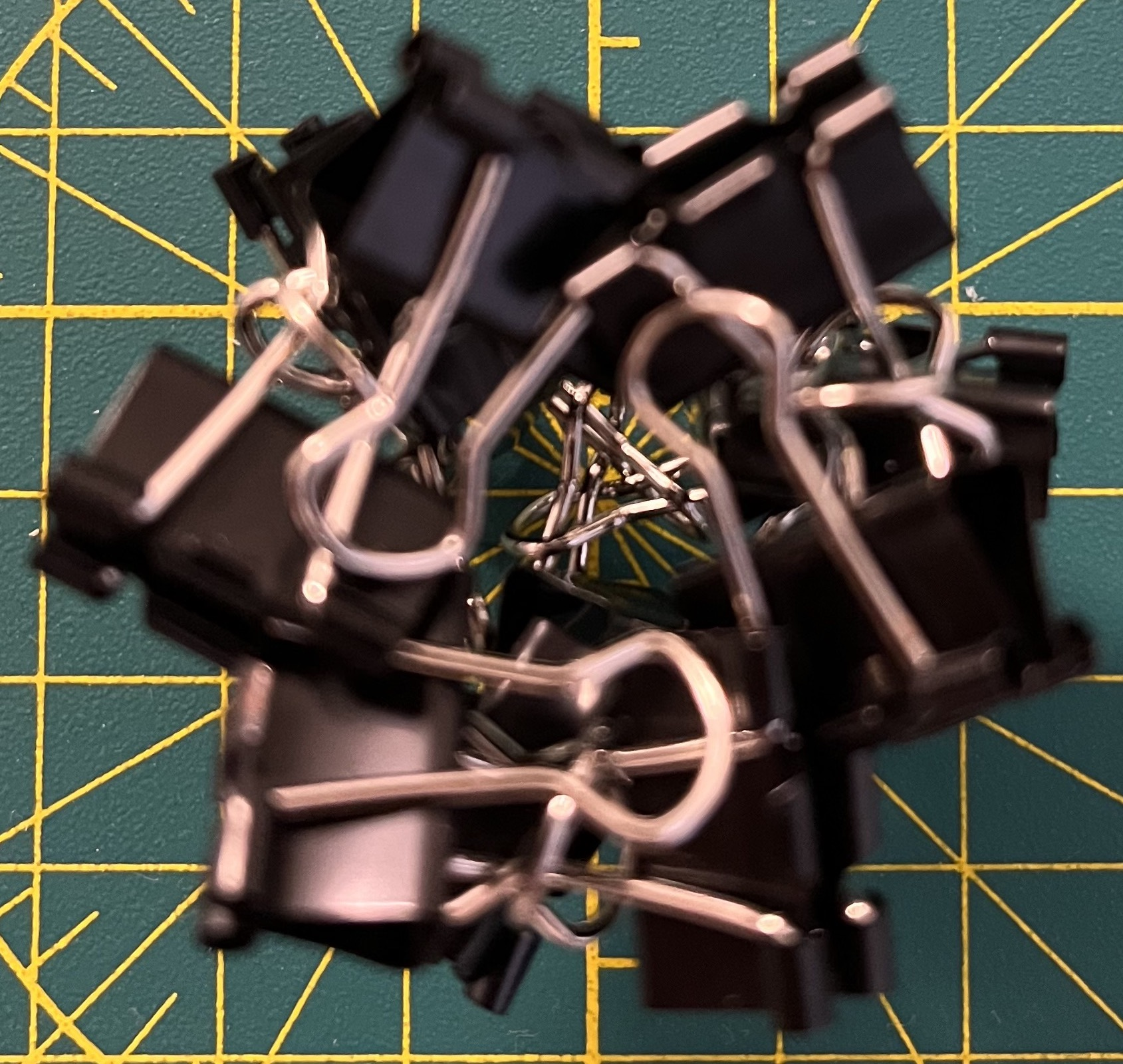↑ # Clips = 12
↑ Base = tetrahedron
↑ Vertex config = 3.3.3
↑ Symmetry = tetrahedron’s rotations
↑ Dual = itself

#### Φ24-C↑ # Clips = 24
↑ Base = cube
↑ Vertex config = 4.4.4
↑ Symmetry = cube’s rotations
↑ Dual = Φ24-O

#### Φ24-O↑ # Clips = 24
↑ Base = octahedron
↑ Vertex config = 3.3.3.3
↑ Symmetry = cube’s rotations
↑ Dual = Φ24-C

#### ΦB60-I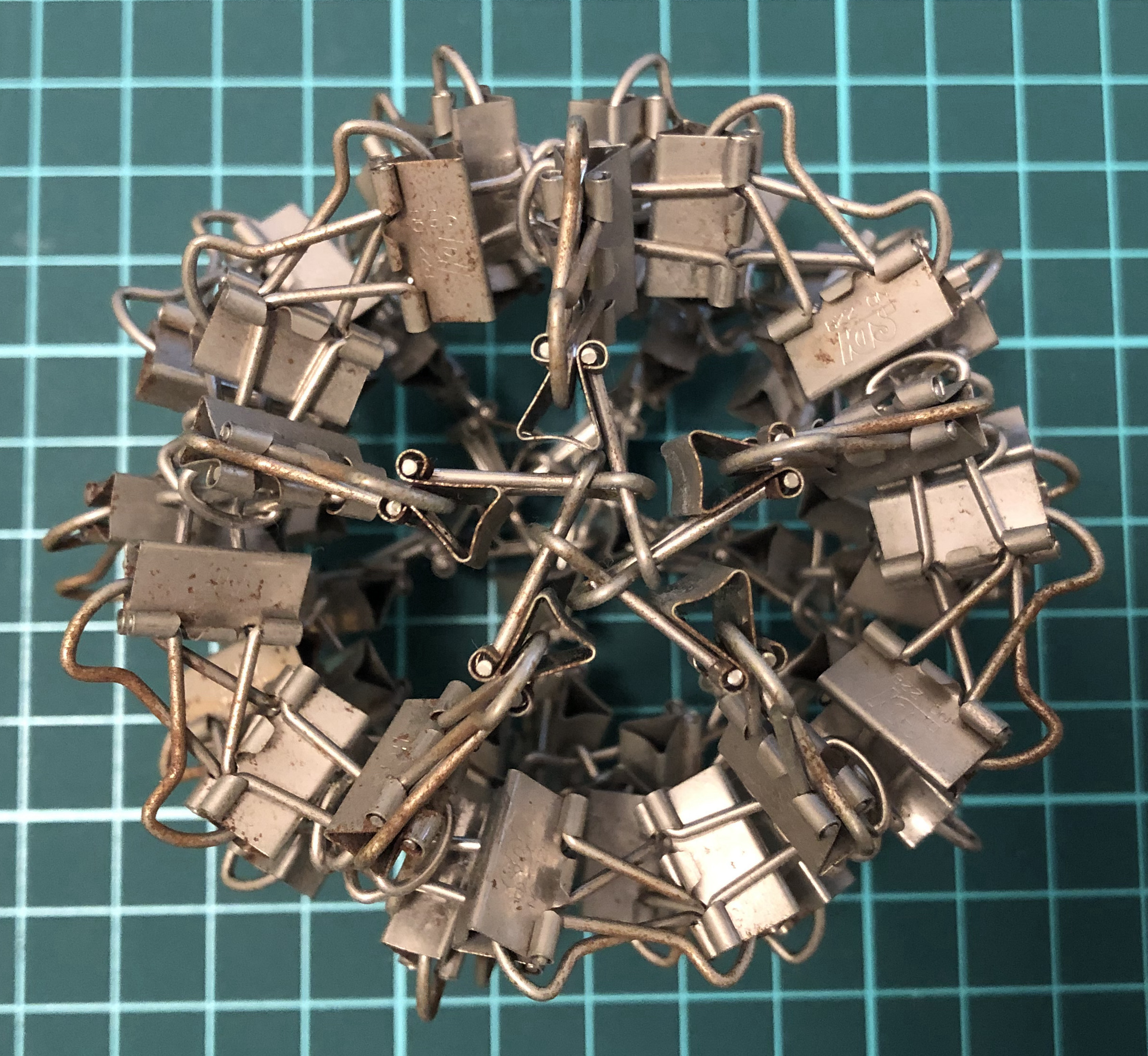↑ # Clips = 60
↑ Vertex config = 3.3.3.3.3
↑ Base = icosahedron
↑ Symmetry = dodecahedron’s rotations

#### ΦJ60-D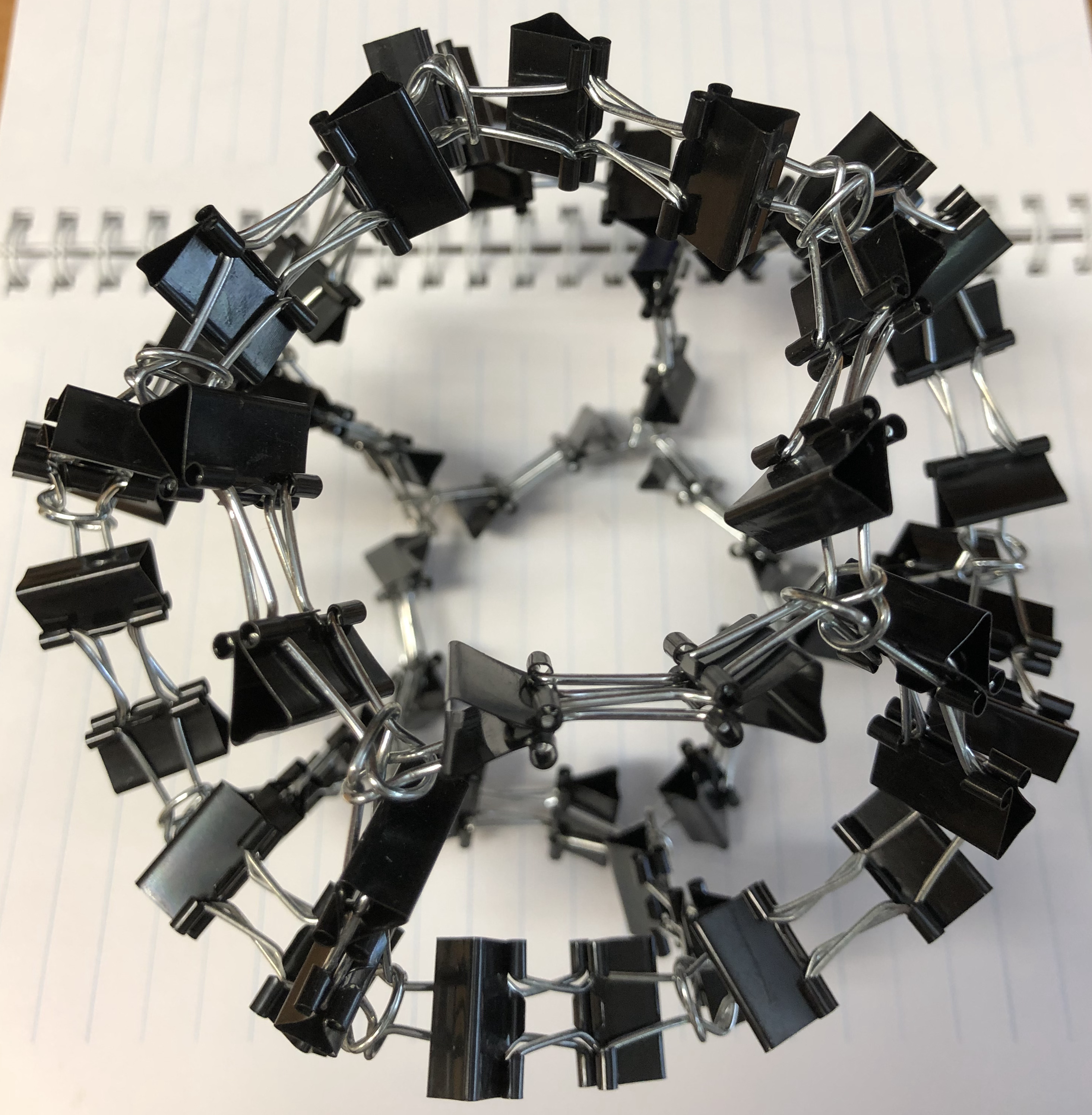↑ # Clips = 60
↑ Vertex config = 5.5.5
↑ Base = dodecahedron
↑ Symmetry = dodecahedron’s rotations

### Ψ-series

Three clips = one Ψ-vertex = one vertex.

#### Ψ12-T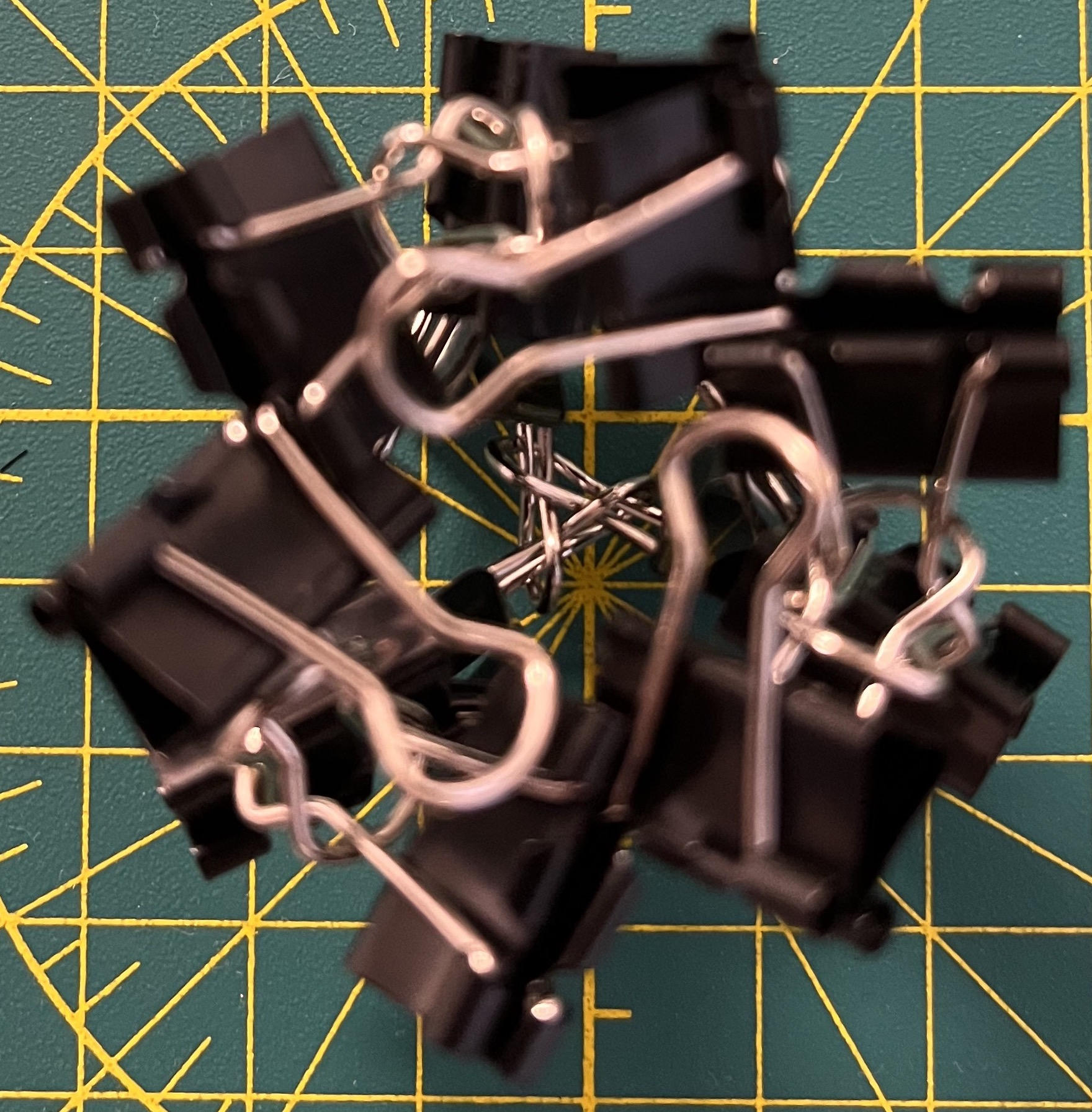↑ # Clips = 12
↑ Base = tetrahedron
↑ Vertex config = 3.3.3
↑ Symmetry = tetrahedron’s rotations
↑ Dual = itself

#### Ψ24-C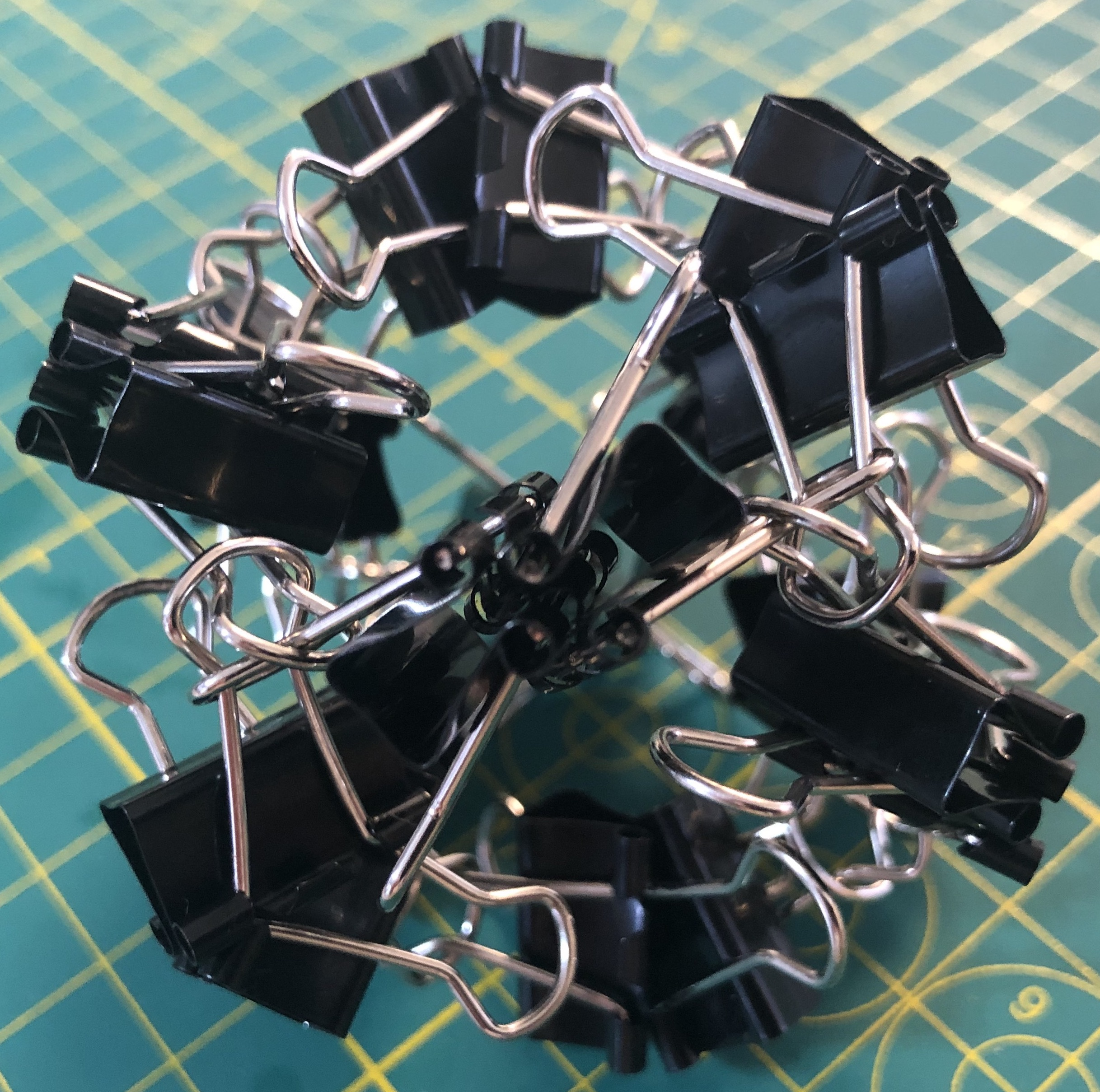↑ # Clips = 24
↑ Base = cube
↑ Vertex config = 4.4.4
↑ Symmetry = cube’s rotations
↑ Dual = Ψ24-O

#### Ψ24-O↑ # Clips = 24
↑ Base = octahedron
↑ Vertex config = 3.3.3.3
↑ Symmetry = cube’s rotations
↑ Dual = Ψ24-C

### Δ-series

Three clips = one Δ-vertex = one vertex.

#### Δ60-D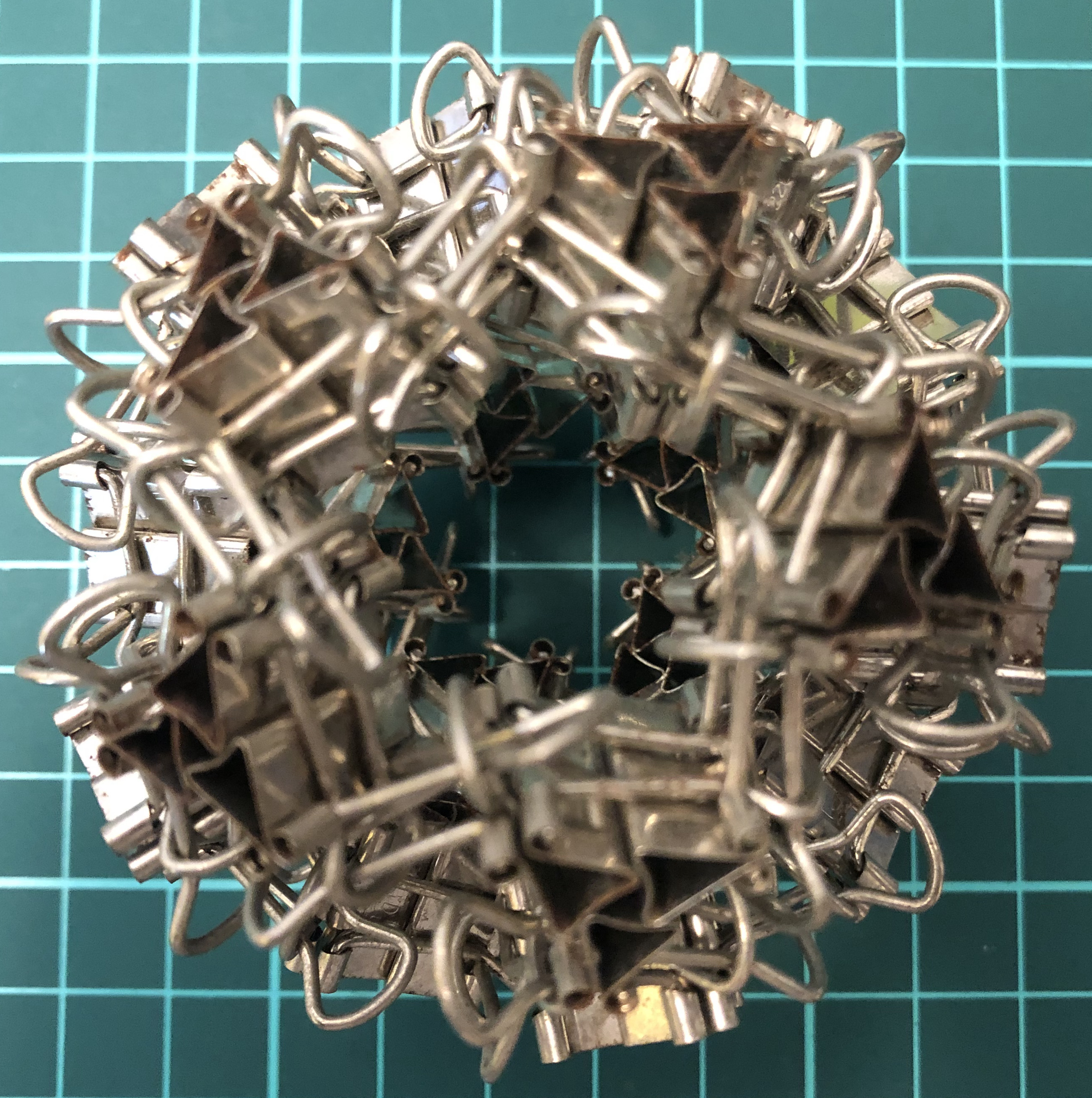↑ # Clips = 60
↑ Vertex config = 5.5.5
↑ Base = dodecahedron
↑ Symmetry = dodecahedron’s rotations

#### Δ180-tI↑ # Clips = 180
↑ Vertex config = 5.6.6
↑ Base = truncated icosahedron
↑ Symmetry = dodecahedron’s rotations

## Edge unit

### Y-series

Three clips = one Y-edge = one edge.

#### Y18-T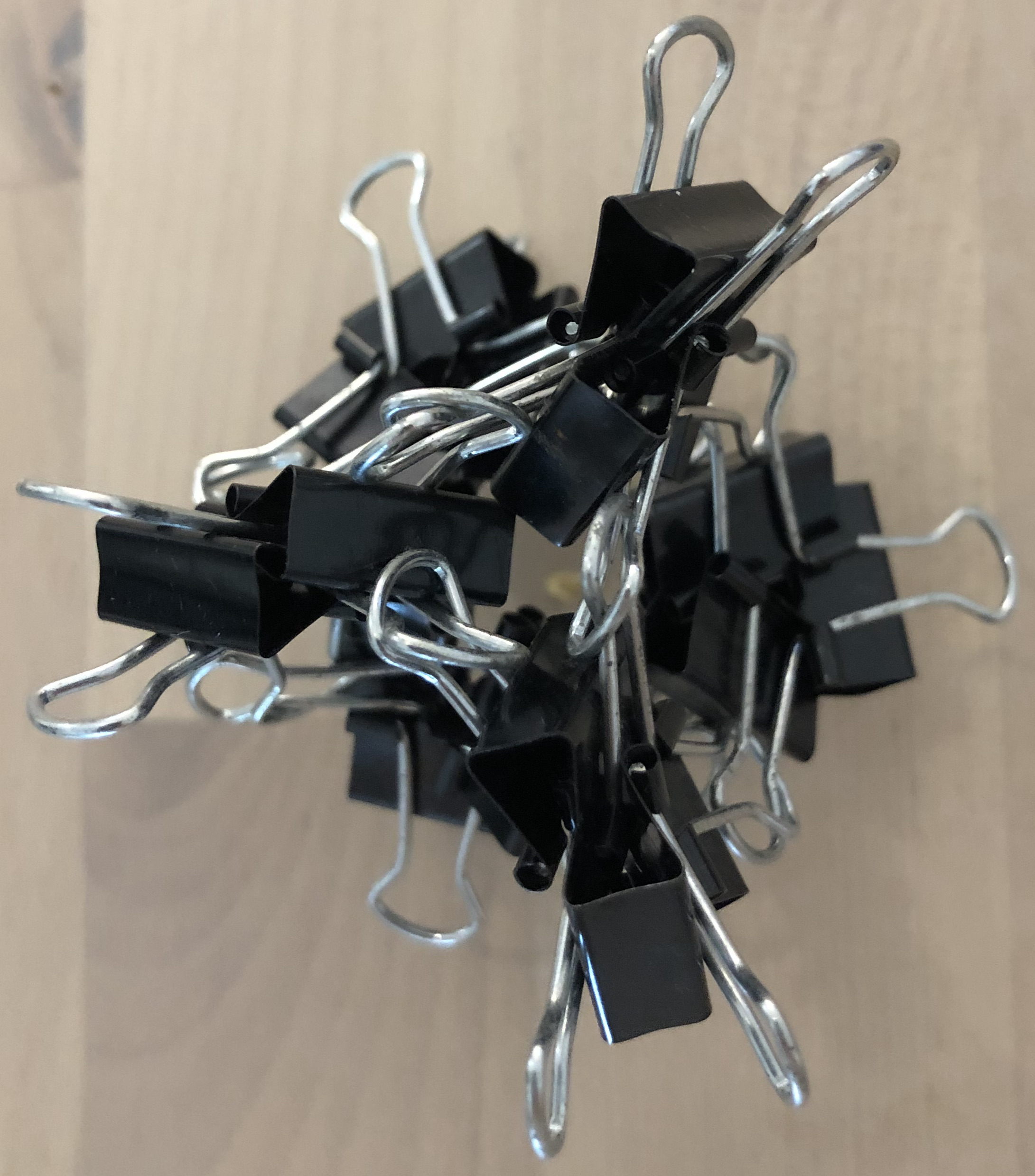↑ # Clips = 24
↑ Base = tetrahedron
↑ Vertex config = 3.3.3
↑ Symmetry = tetrahedron’s rotations

### X-series

Two clips = one X-edge = one edge.

#### X12-T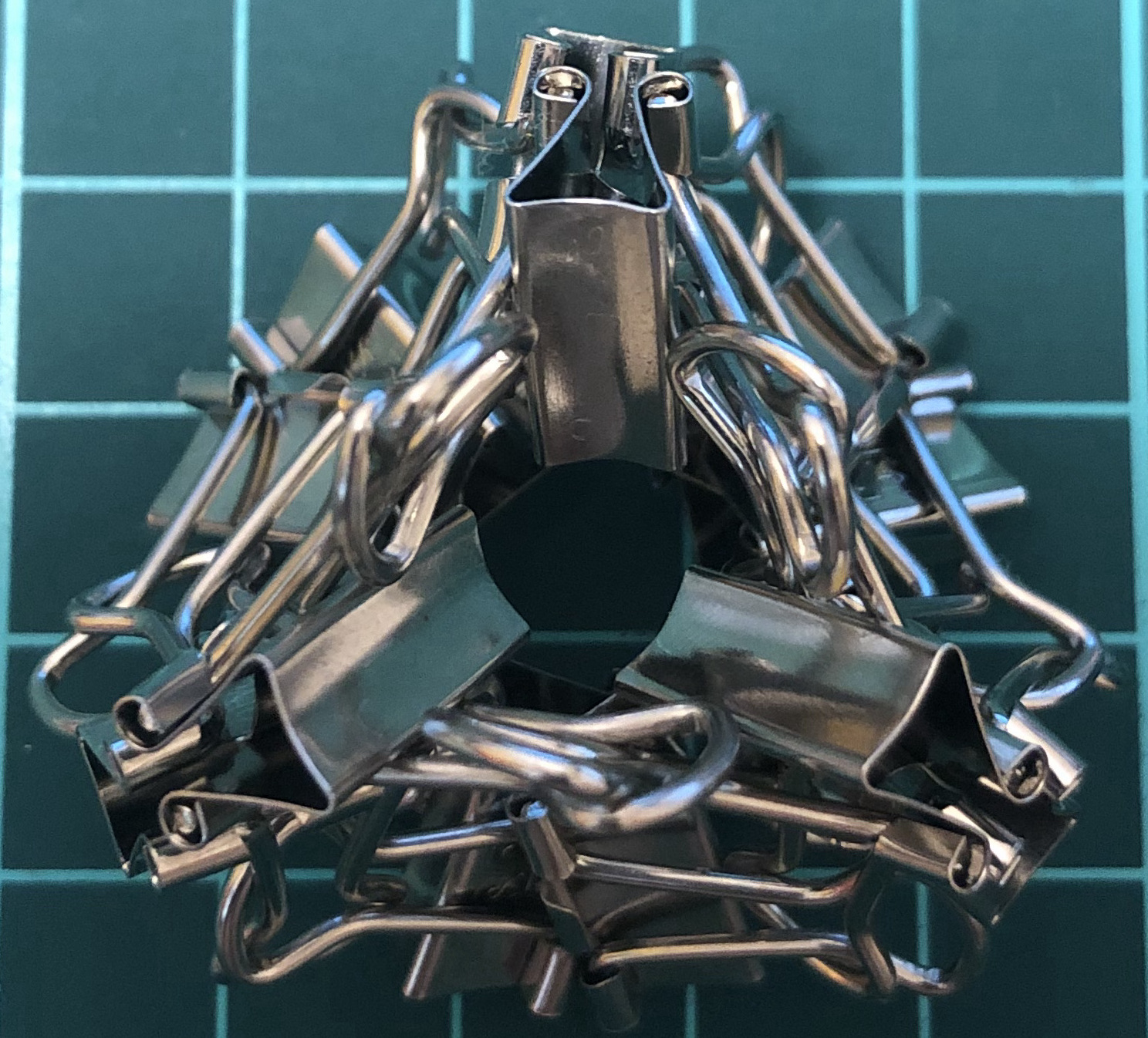↑ # Clips = 12
↑ Base = tetrahedron
↑ Vertex config = 3.3.3
↑ Symmetry = tetrahedron’s rotations
↑ Dual = itself

#### X24-C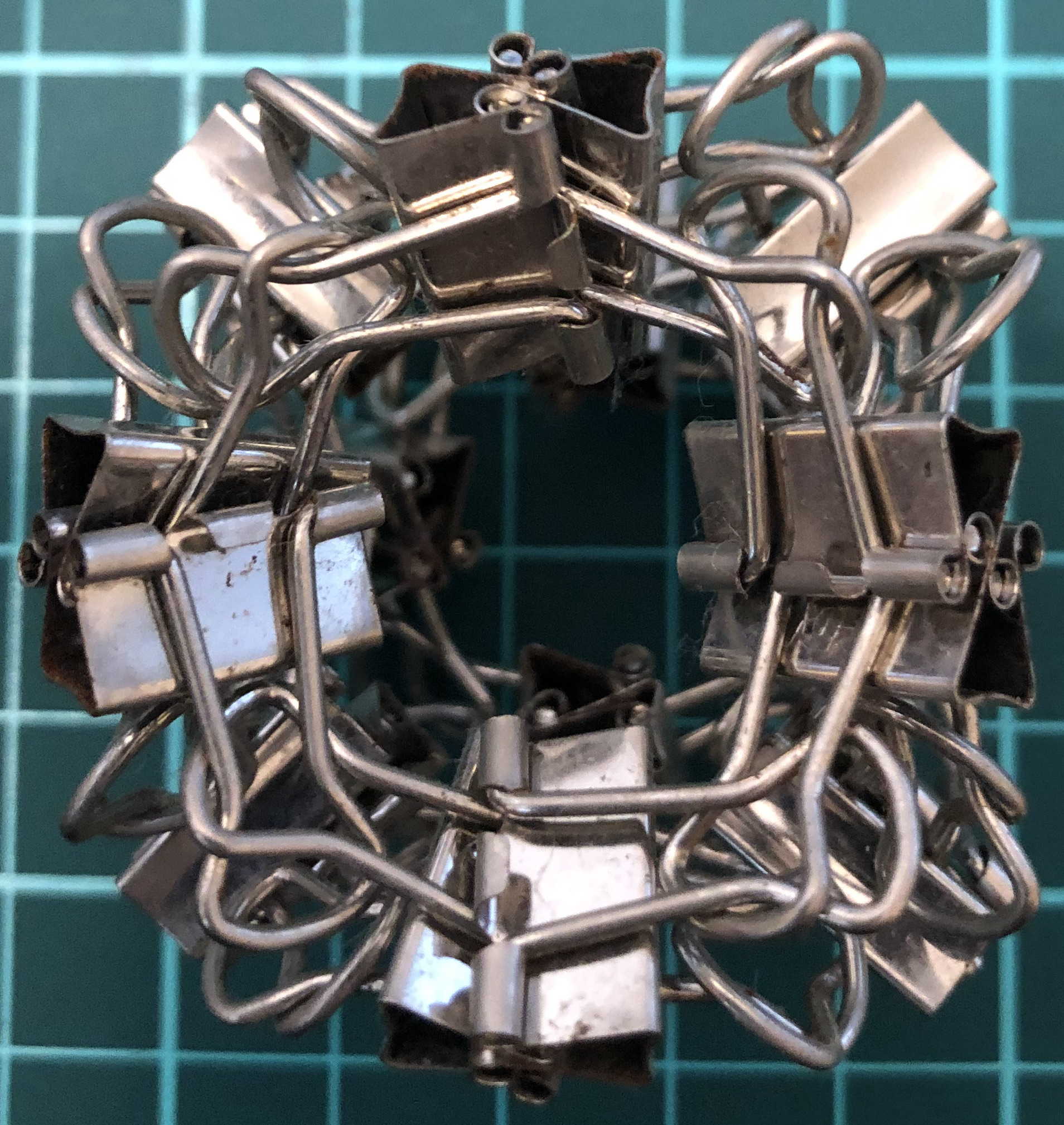↑ # Clips = 24
↑ base = cube
↑ Vertex config = 4.4.4
↑ Symmetry = cube’s rotations
↑ dual = X24-O

#### X24-O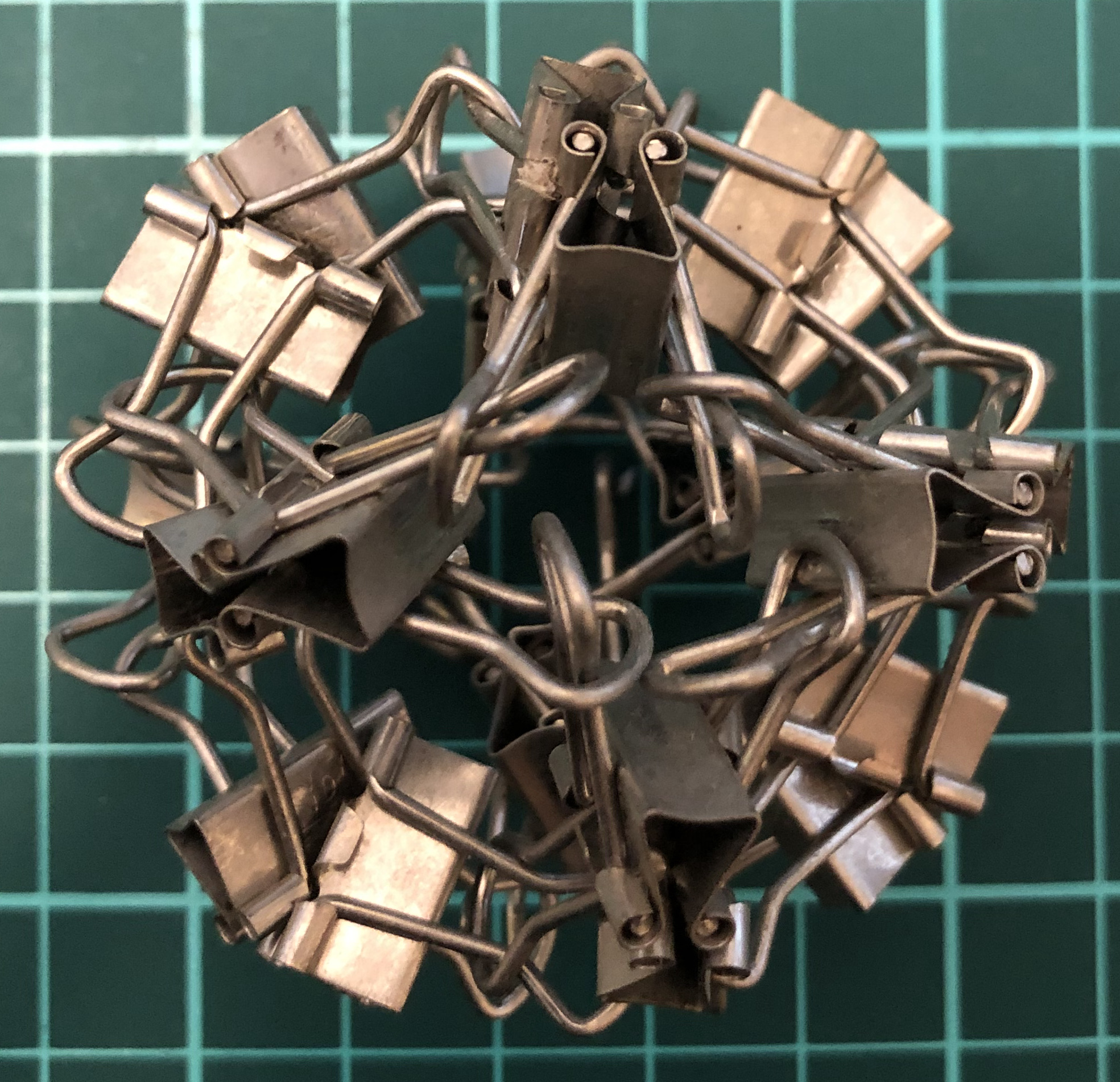↑ # Clips = 24
↑ base = octahedron
↑ Vertex config = 3.3.3.3
↑ Symmetry = cube’s rotations
↑ dual = X24-C

#### X60-D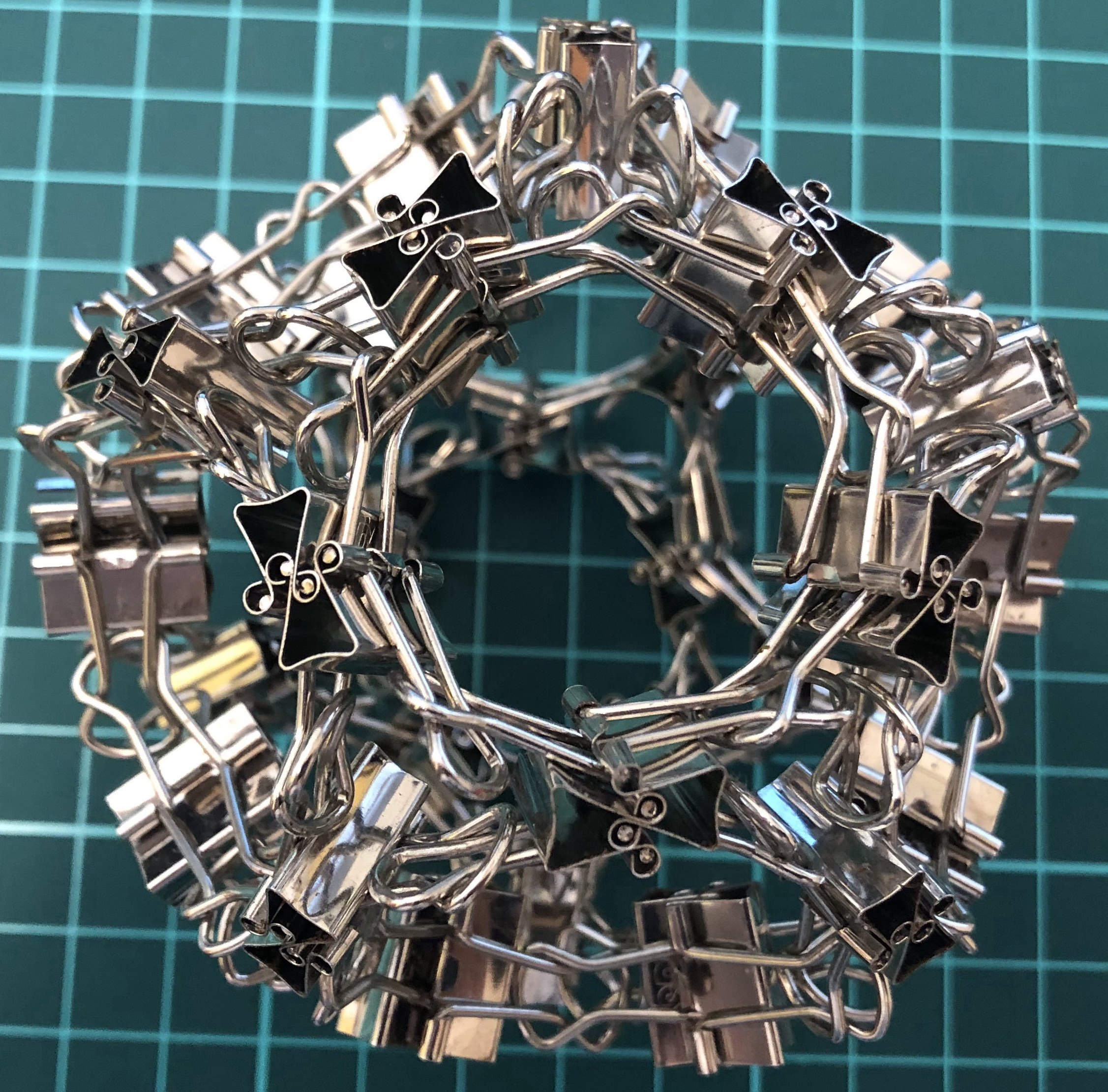↑ # Clips = 60
↑ Vertex config = 5.5.5
↑ Base = dodecahedron
↑ Symmetry = dodecahedron’s rotations
↑ dual = X60-I

#### X60-I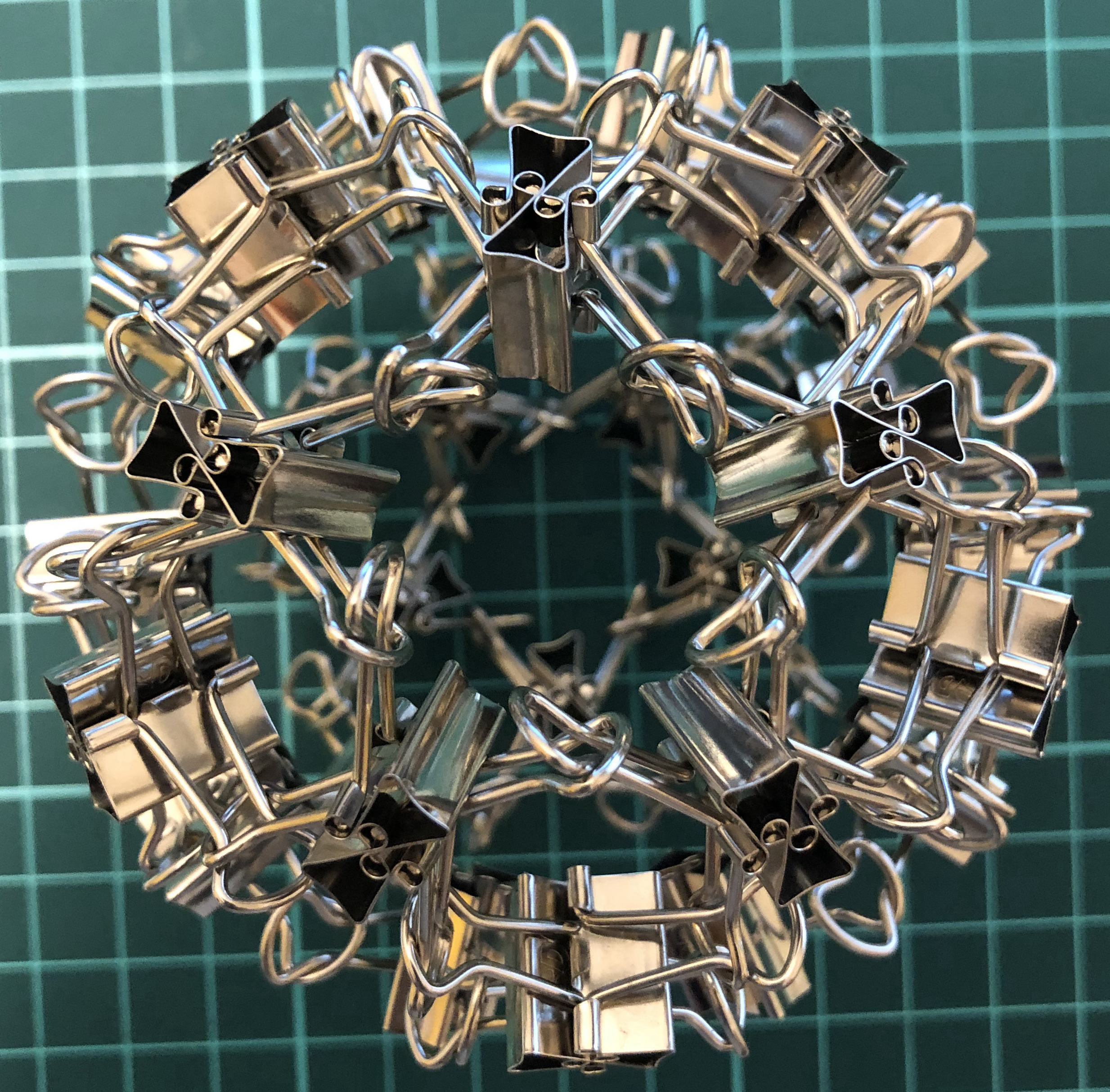↑ # Clips = 60
↑ Vertex config = 3.3.3.3.3
↑ Base = icosahedron
↑ Symmetry = dodecahedron’s rotations
↑ dual = X60-D

#### XX120-D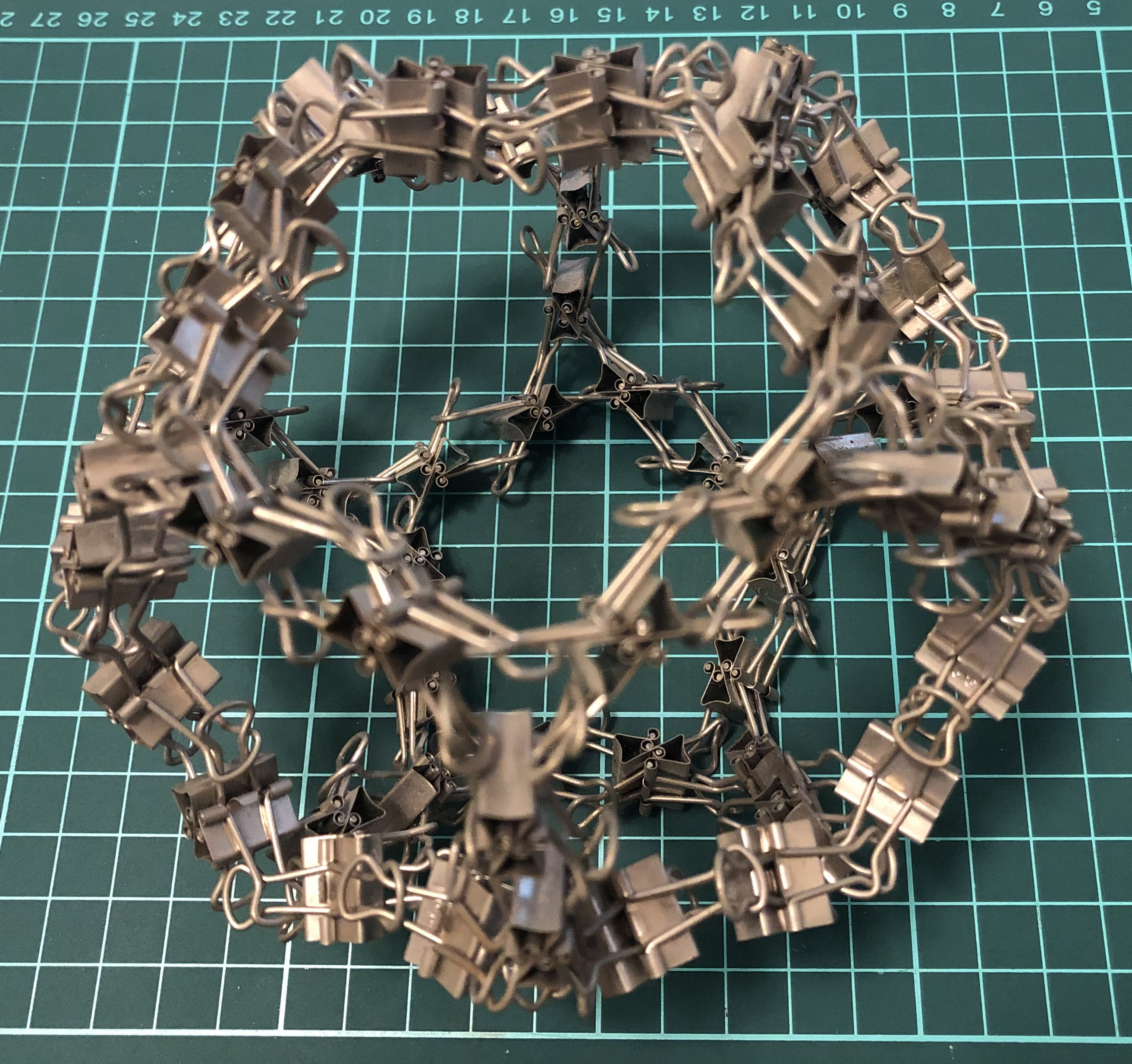↑ # Clips = 120
↑ Vertex config = 5.5.5
↑ Base = dodecahedron
↑ Symmetry = dodecahedron’s rotations

### L-series

Two clips = one L-edge = one edge.

#### L12-T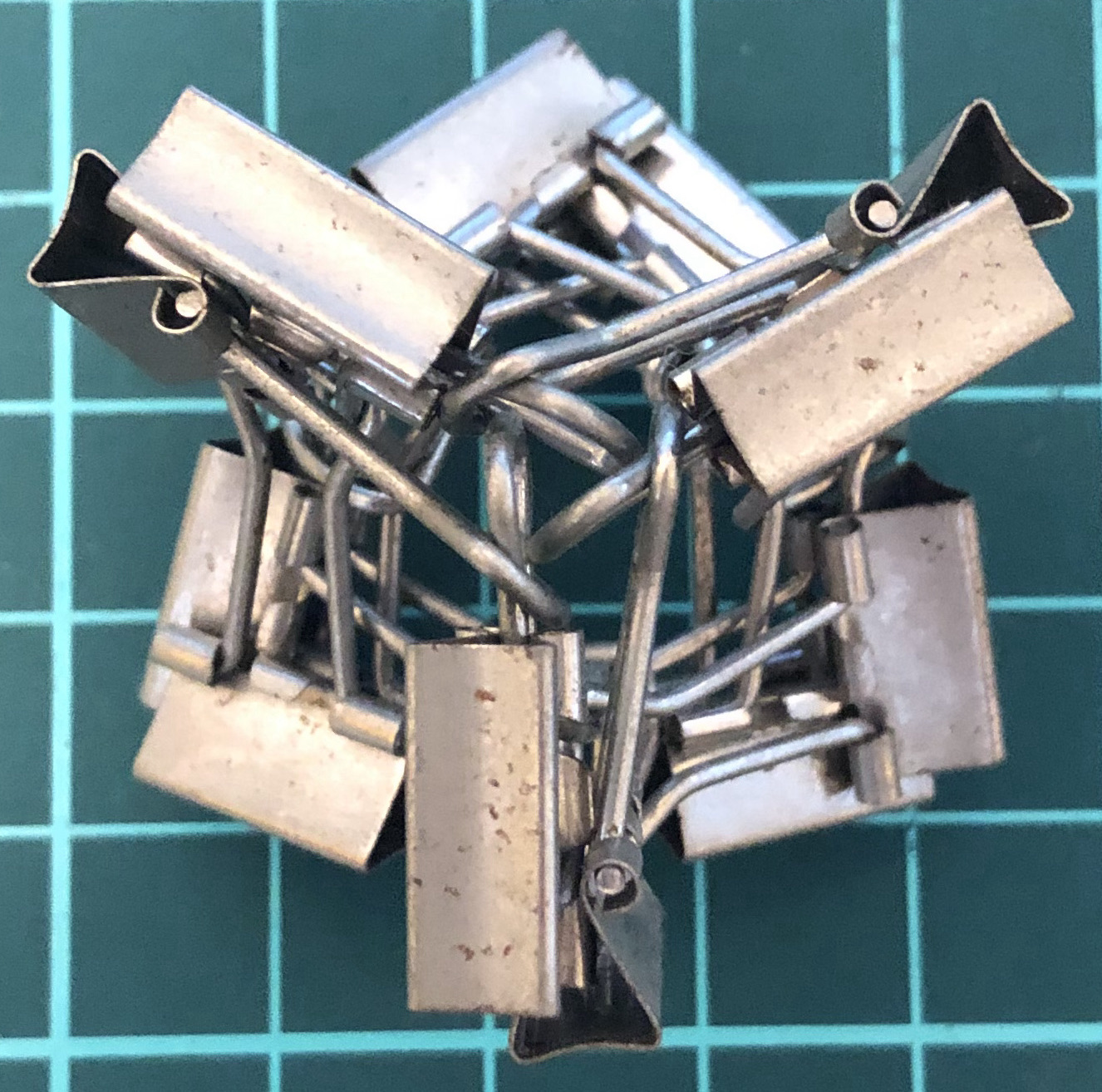↑ # Clips = 12
↑ Base = tetrahedron
↑ Vertex config = 3.3.3
↑ Symmetry = tetrahedron’s rotations
↑ Dual = itself

#### L24-C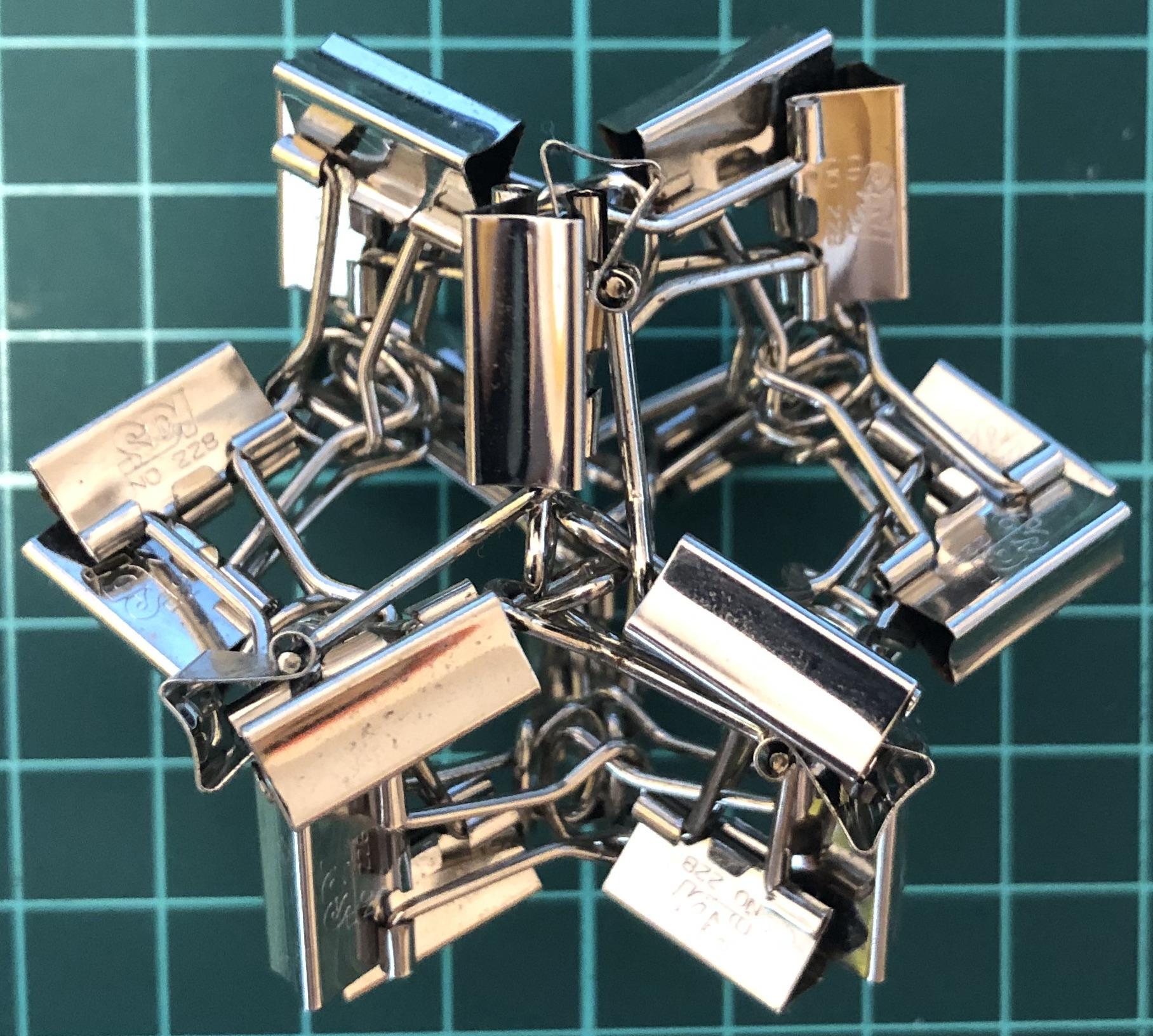↑ # Clips = 24
↑ Base = cube
↑ Vertex config = 4.4.4
↑ Symmetry = cube’s rotations
↑ Dual = L24-O

#### L24-O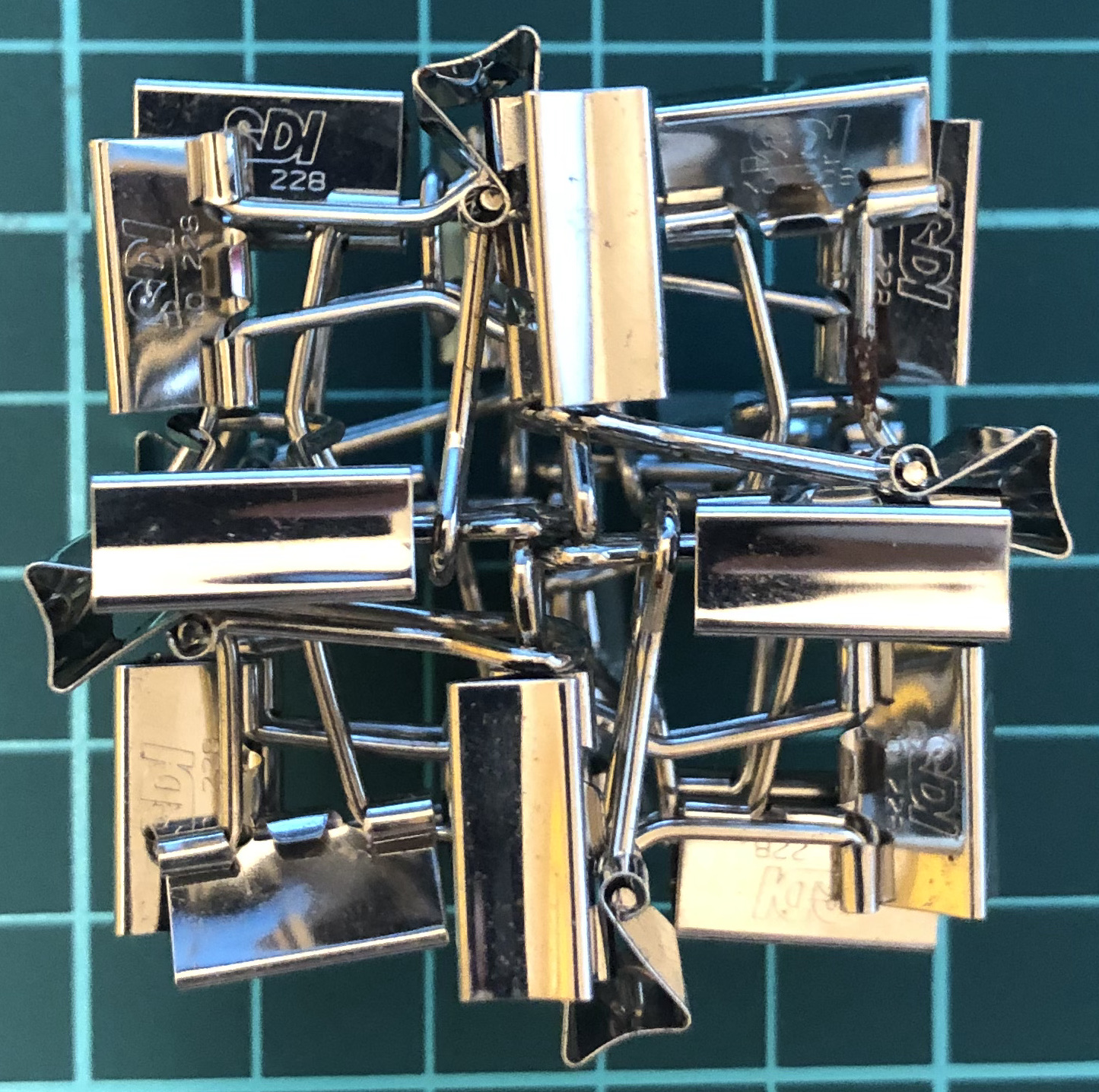↑ # Clips = 24
↑ Base = octahedron
↑ Vertex config = 3.3.3.3
↑ Symmetry = cube’s rotations
↑ Dual = L24-C

#### L60-D↑ # Clips = 60
↑ Base = dodecahedron
↑ Vertex config = 5.5.5
↑ Symmetry = dodecahedron’s rotations
↑ Dual = L60-I

#### L60-I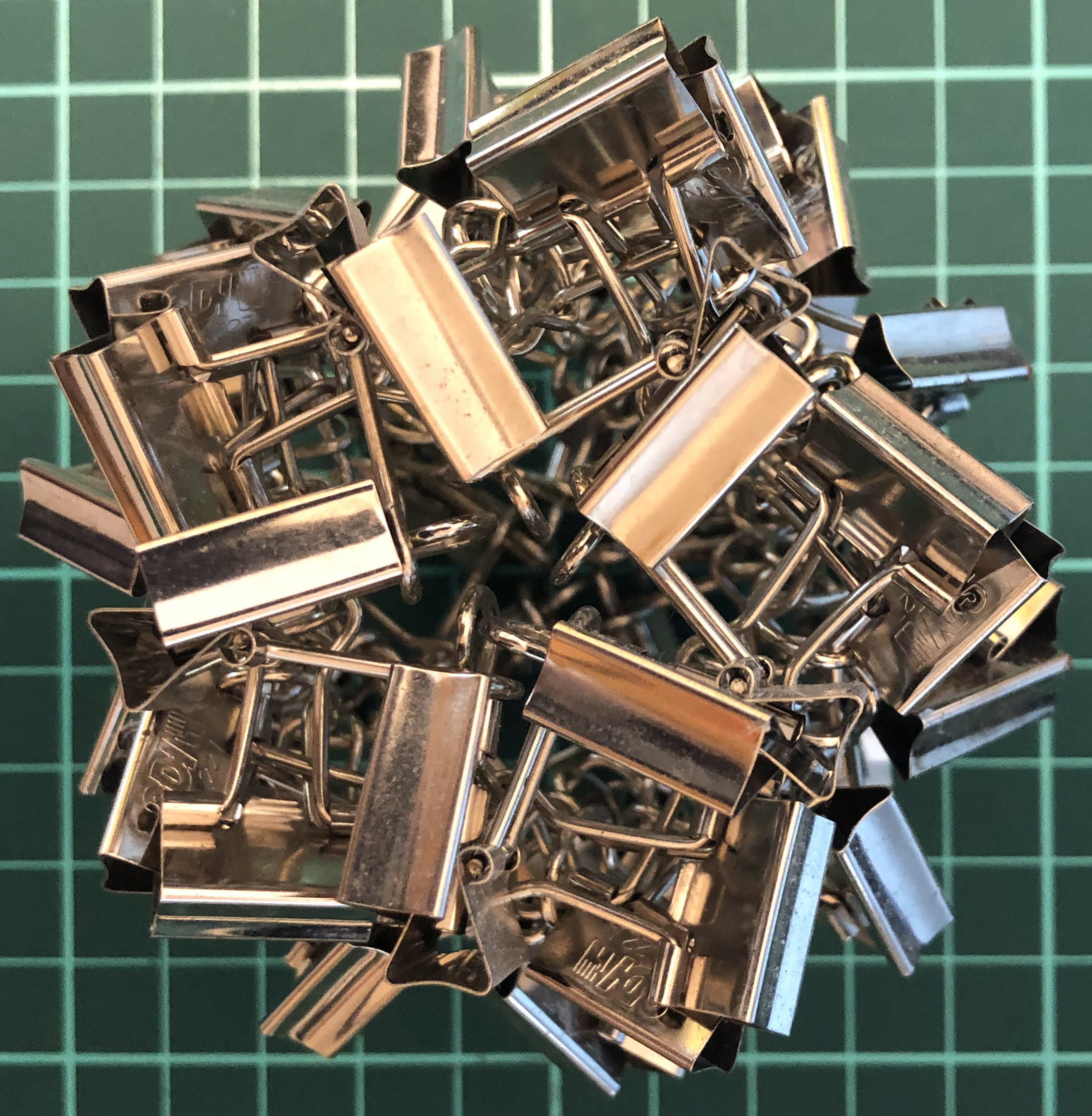↑ # Clips = 60
↑ Base = icosahedron
↑ Vertex config = 3.3.3.3.3
↑ Symmetry = dodecahedron’s rotations
↑ Dual = L60-D

#### L36-tT↑ # Clips = 36
↑ Base = truncated tetrahedron
↑ Vertex config = 3.6.6
↑ Symmetry = tetrahedron’s rotations

#### L48-aC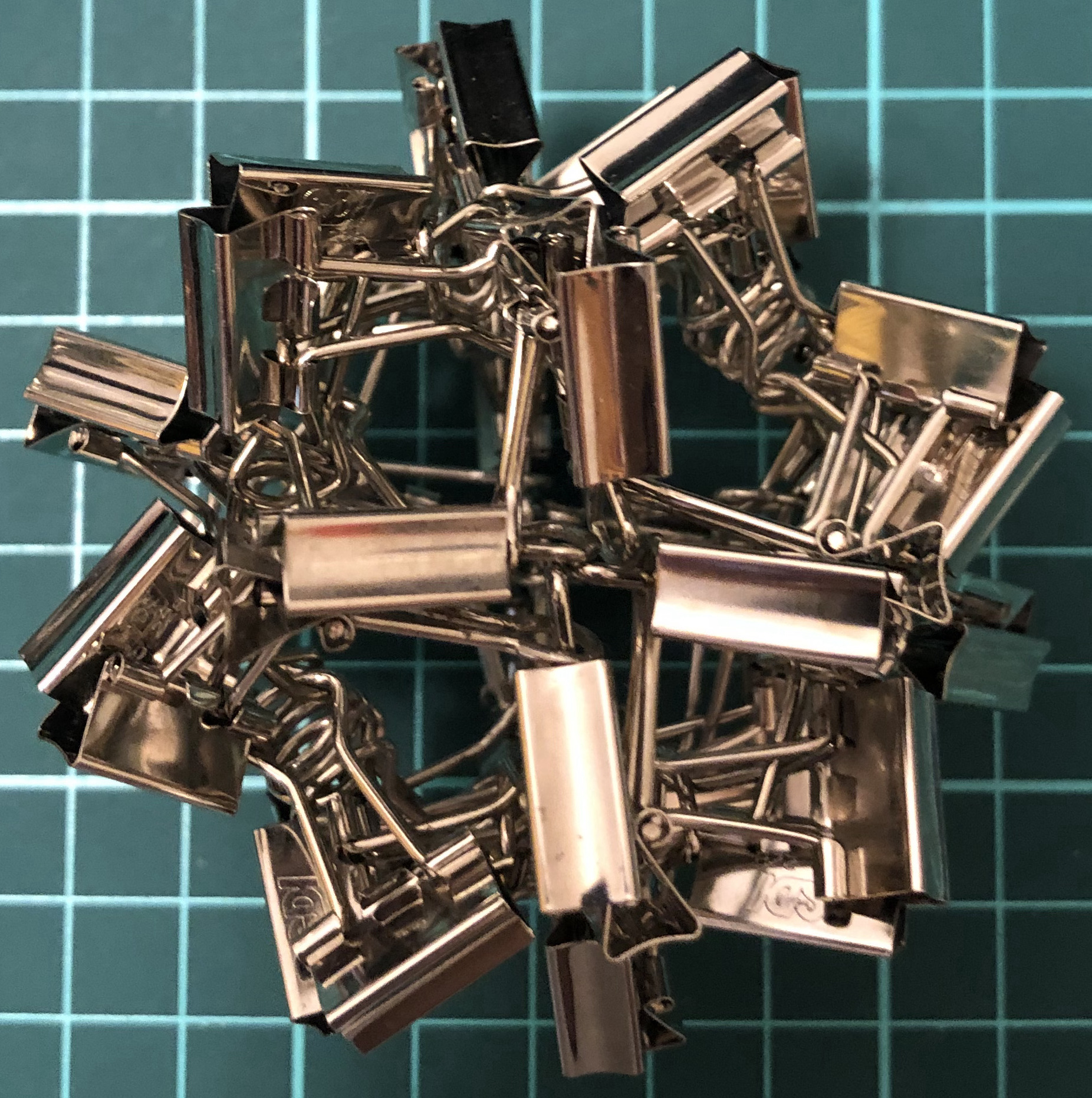↑ # Clips = 48
↑ Base = cuboctahedron
↑ Vertex config = 3.4.3.4
↑ Symmetry = cube’s rotations

#### L180-tI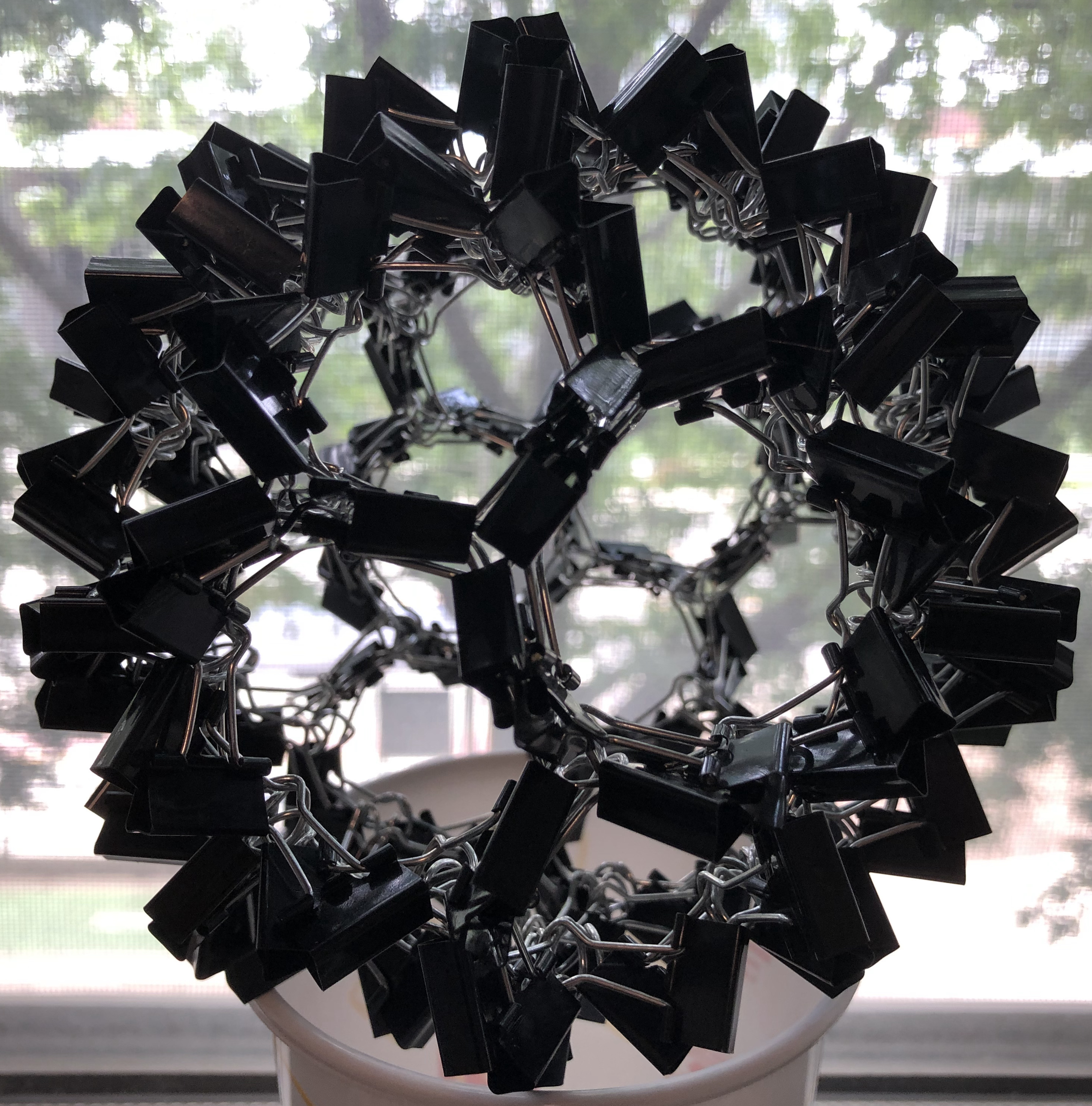↑ # Clips = 180
↑ Base = truncated icosahedron
↑ Vertex config = 5.6.6
↑ Symmetry = dodecahedron’s rotations

### I-series, Platonic

Two clips = one I-edge = one edge.

#### I12-T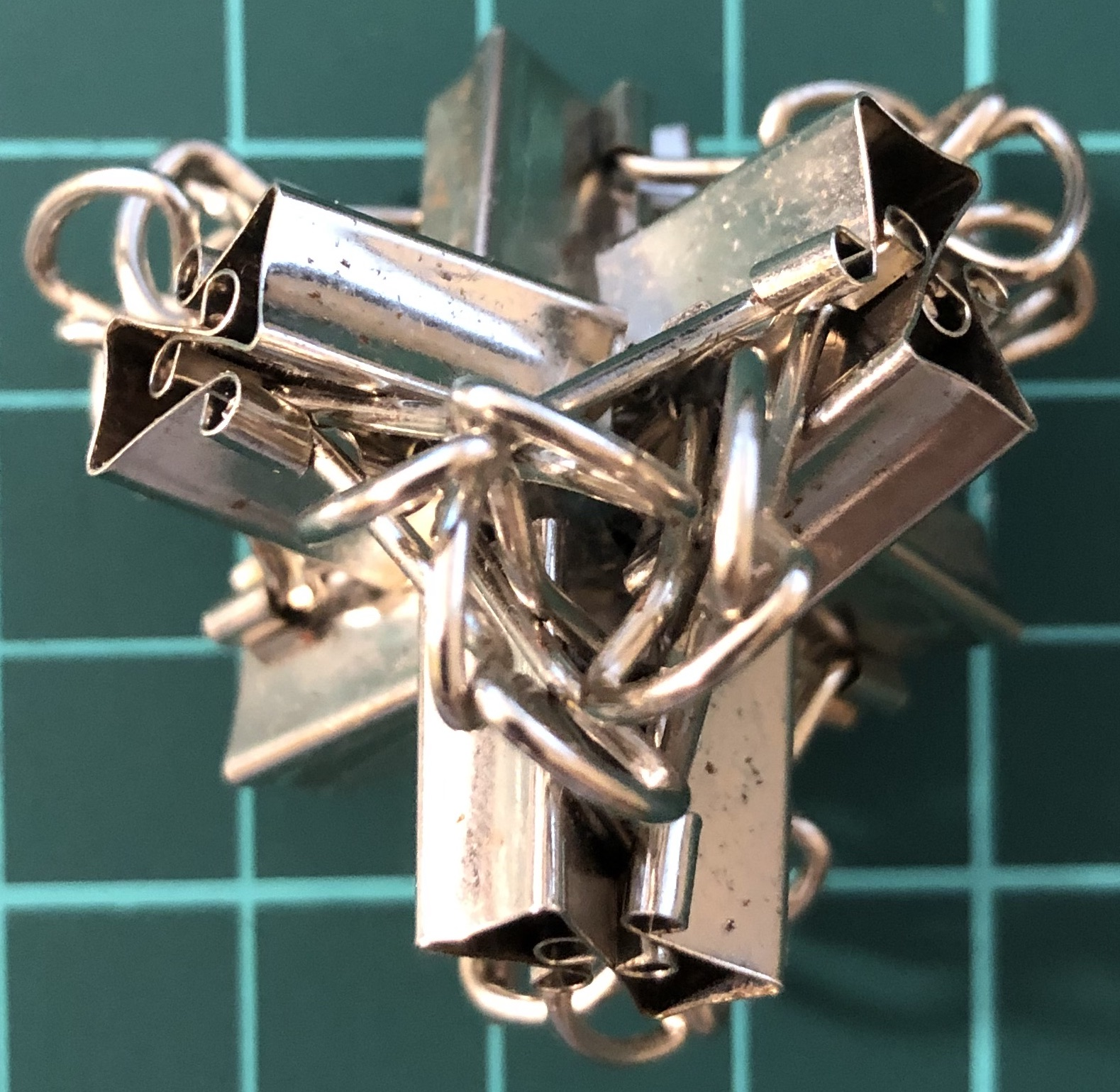↑ # Clips = 12
↑ Base = tetrahedron
↑ Vertex config = 3.3.3
↑ Symmetry = tetrahedron’s rotations
↑ Dual = itself

#### I24-C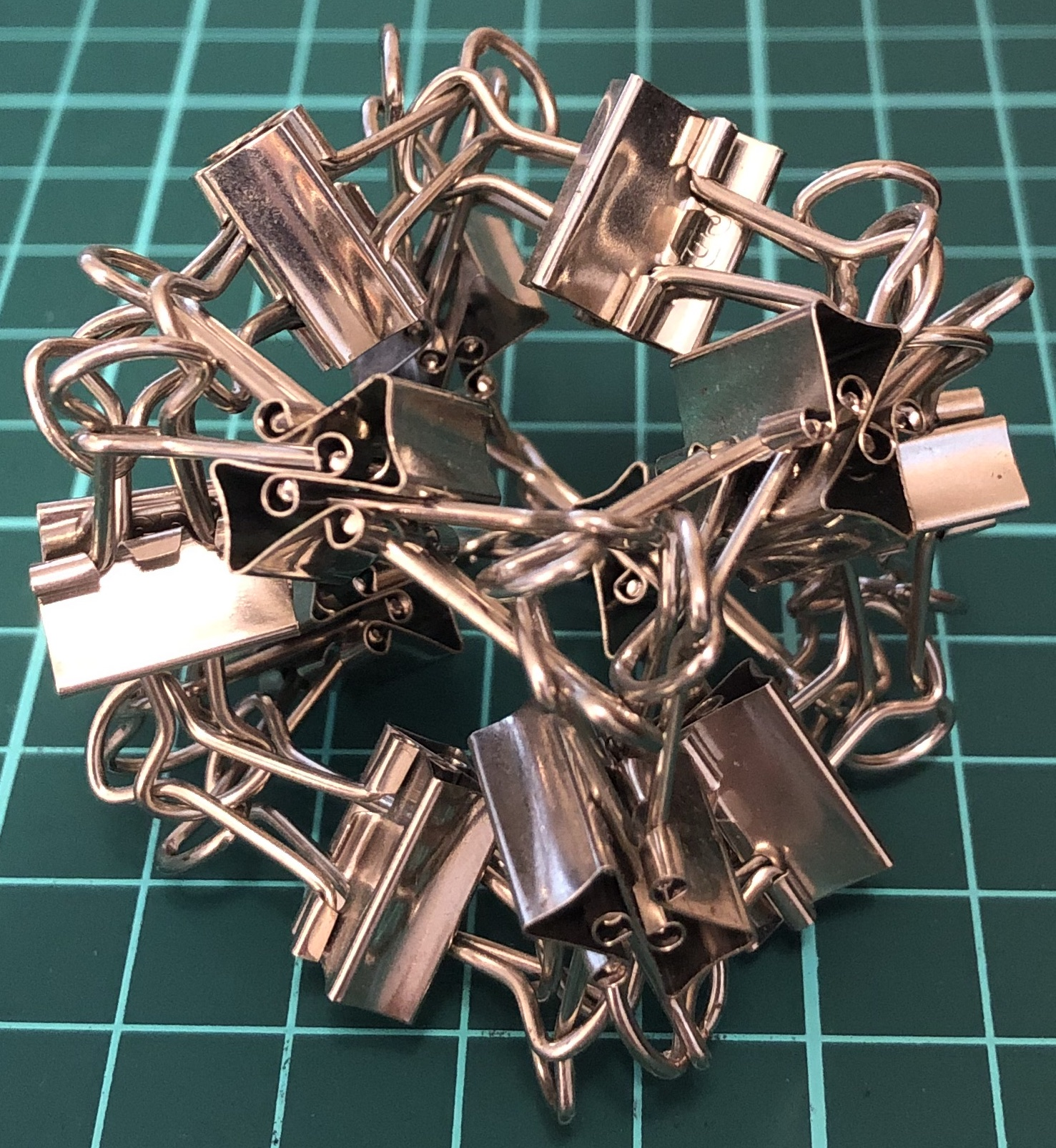↑ # Clips = 24
↑ base = cube
↑ Vertex config = 4.4.4
↑ Symmetry = cube’s rotations
↑ dual = I24-O

#### I24-O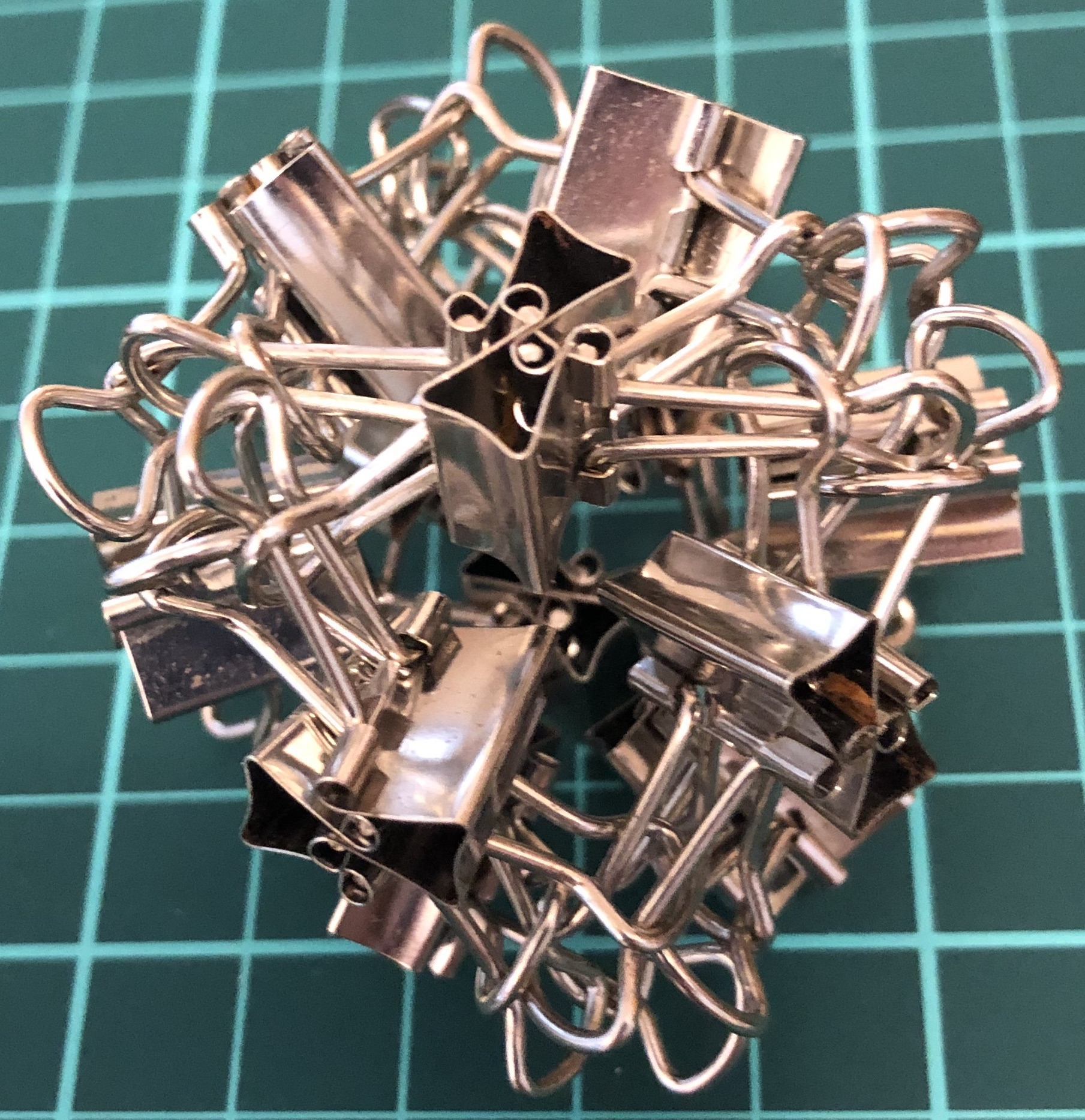↑ # Clips = 24
↑ Base = octahedron
↑ Vertex config = 3.3.3.3
↑ Symmetry = cube’s rotations
↑ Dual = I24-C

#### I60-D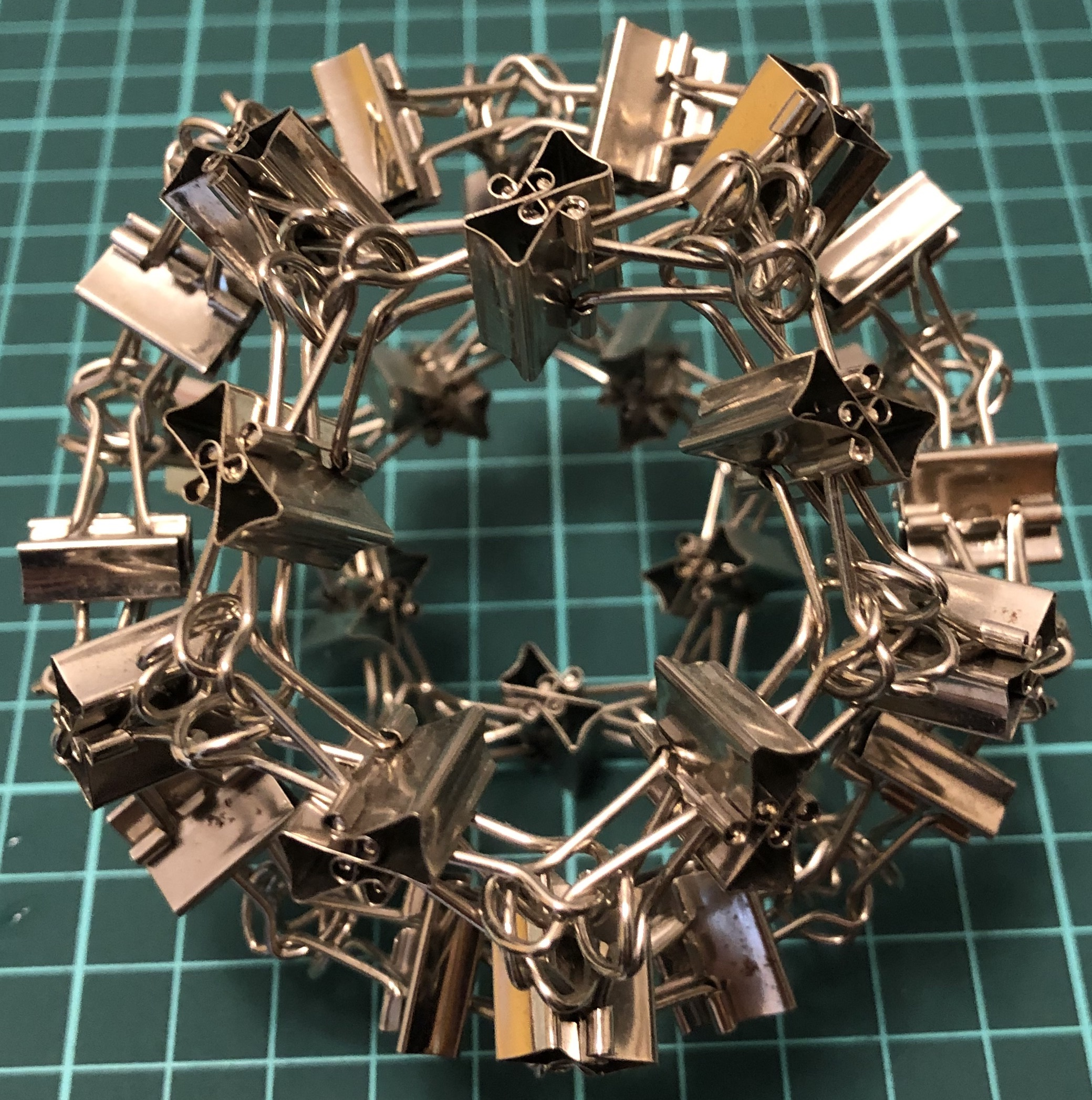↑ # Clips = 30
↑ Vertex config = 5.5.5
↑ Base = dodecahedron
↑ Symmetry = dodecahedron’s rotations
↑ Dual = I60-I

#### I60-I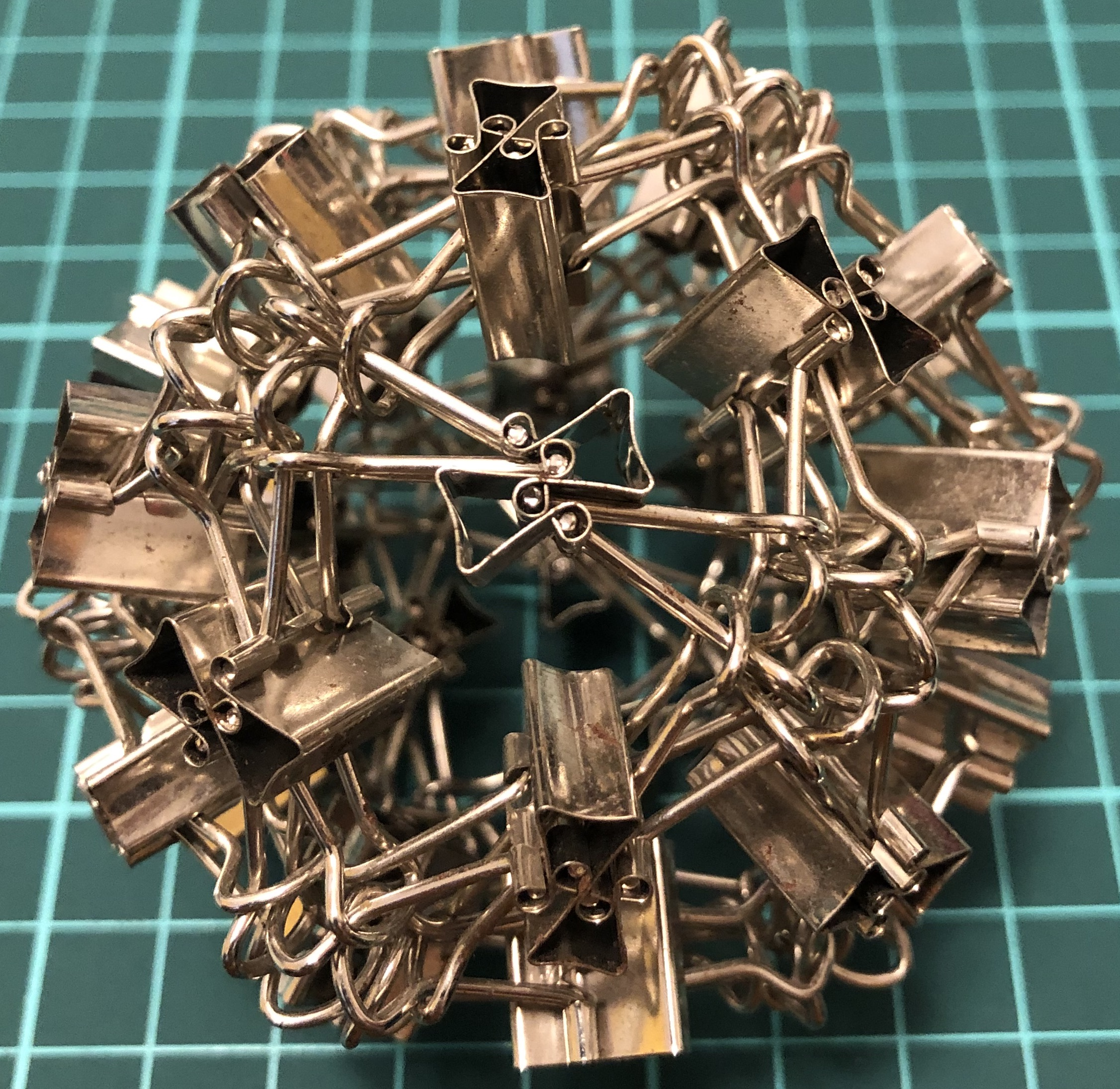↑ # Clips = 30
↑ Base = icosahedron
↑ Vertex config = 3.3.3.3.3
↑ Symmetry = dodecahedron’s rotations
↑ dual = I60-D

#### II24-T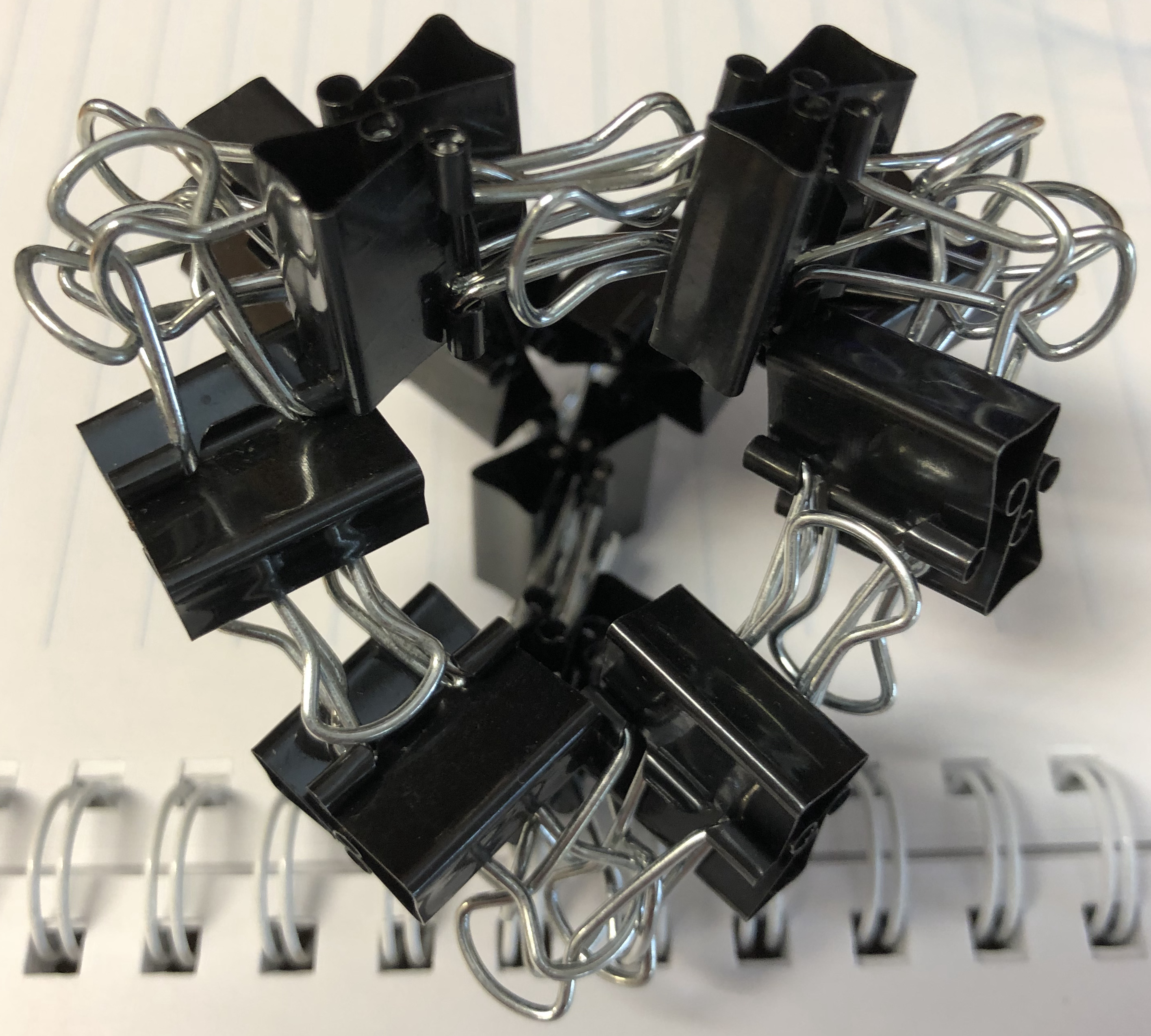↑ # Clips = 24
↑ Base = tetrahedron
↑ Vertex config = 3.3.3
↑ Symmetry = tetrahedron’s rotations
↑ Dual = itself

### I-series, Archimedean

#### I36-tT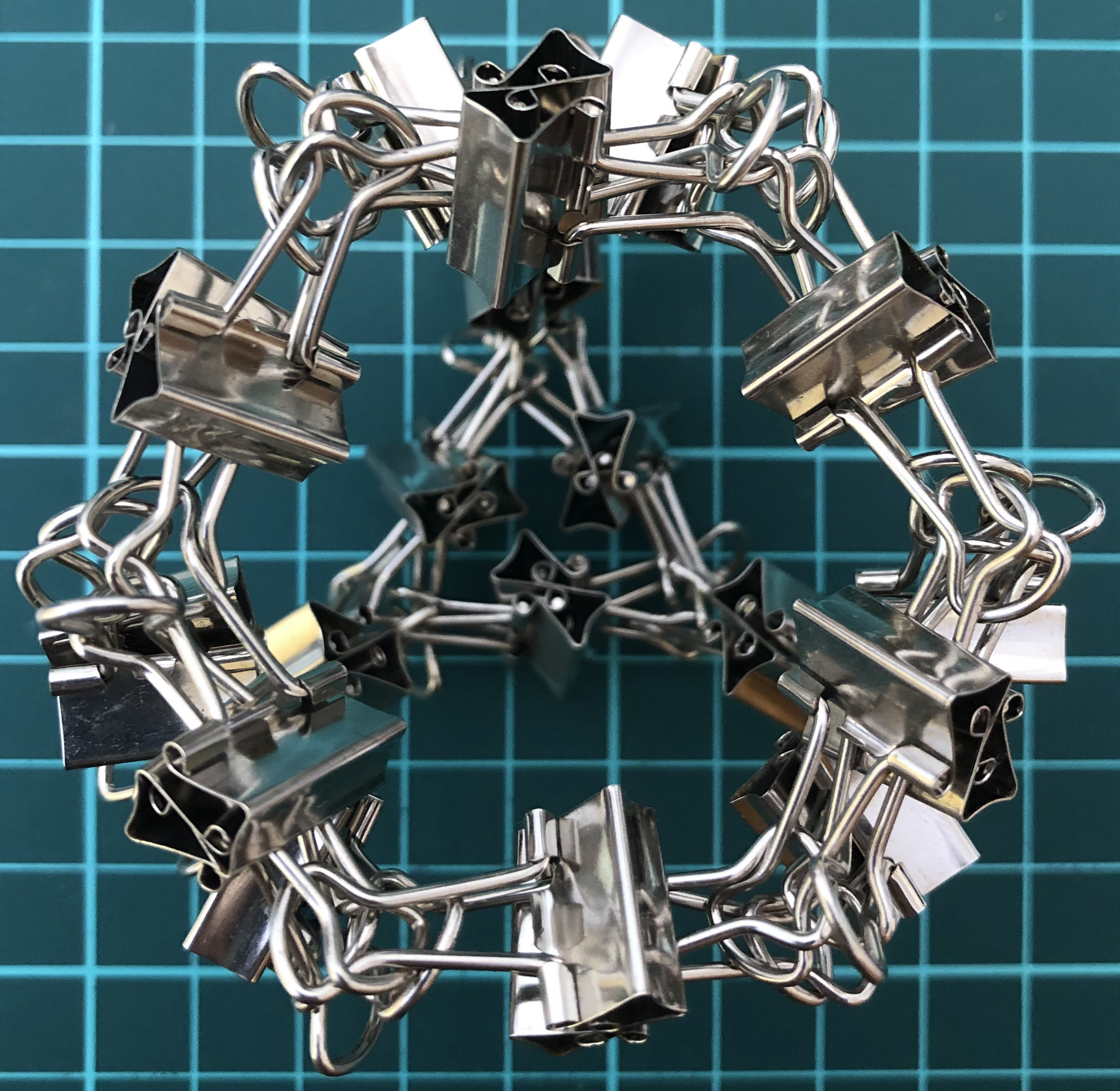↑ # Clips = 36
↑ Base = truncated tetrahedron
↑ Vertex config = 3.6.6
↑ Symmetry = tetrahedron’s rotations
↑ Dual = I36-kT

#### I48-aC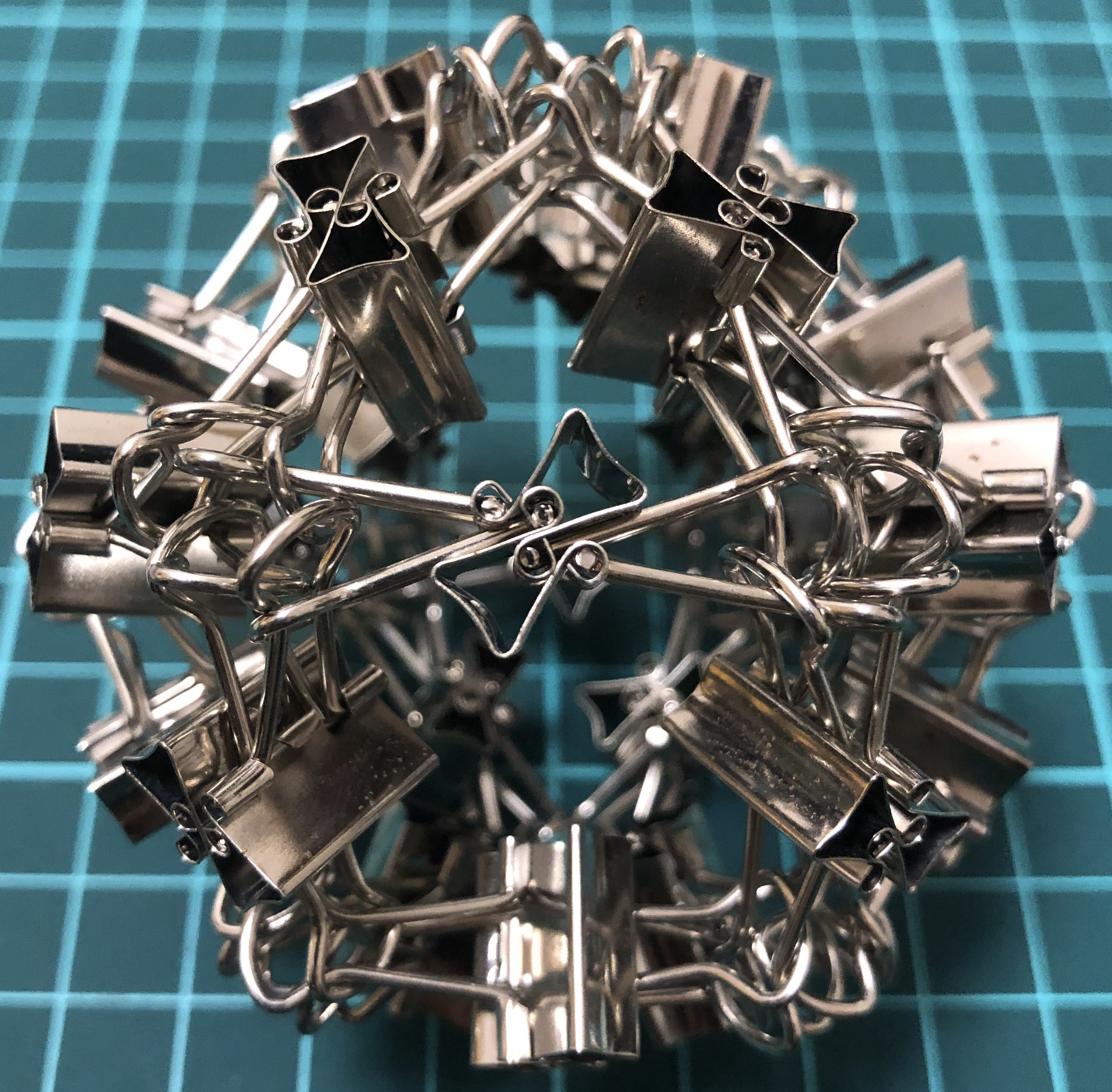↑ # Clips = 48
↑ Base = cuboctahedron
↑ Vertex config = 3.4.3.4
↑ Symmetry = cube’s rotations
↑ Dual = I48-jC

#### I72-tC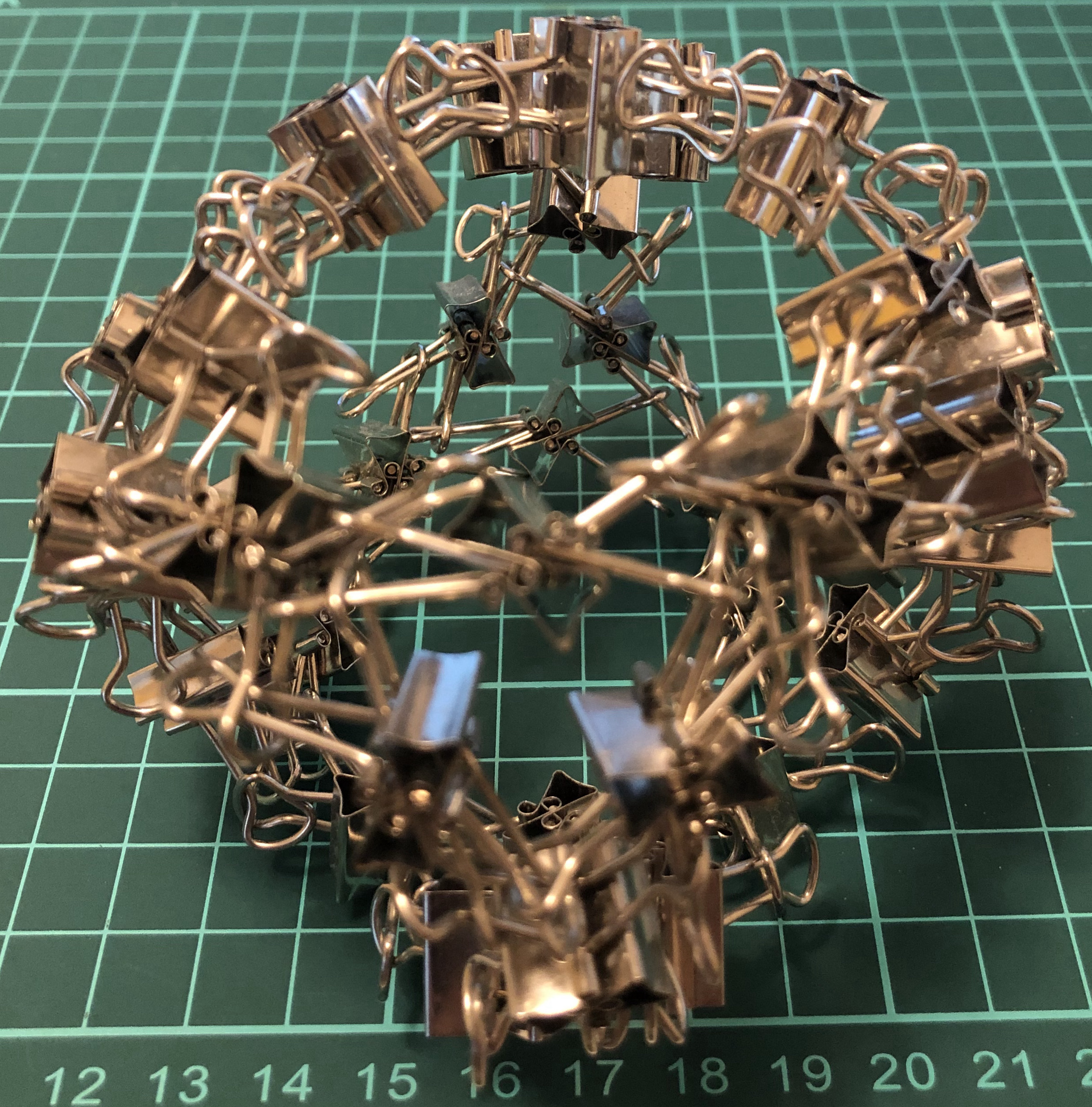↑ # Clips = 72
↑ Base = truncated cube
↑ Vertex config = 3.8.8
↑ Symmetry = cube’s rotations
↑ (Dual = triakis octahedron)

#### I72-tO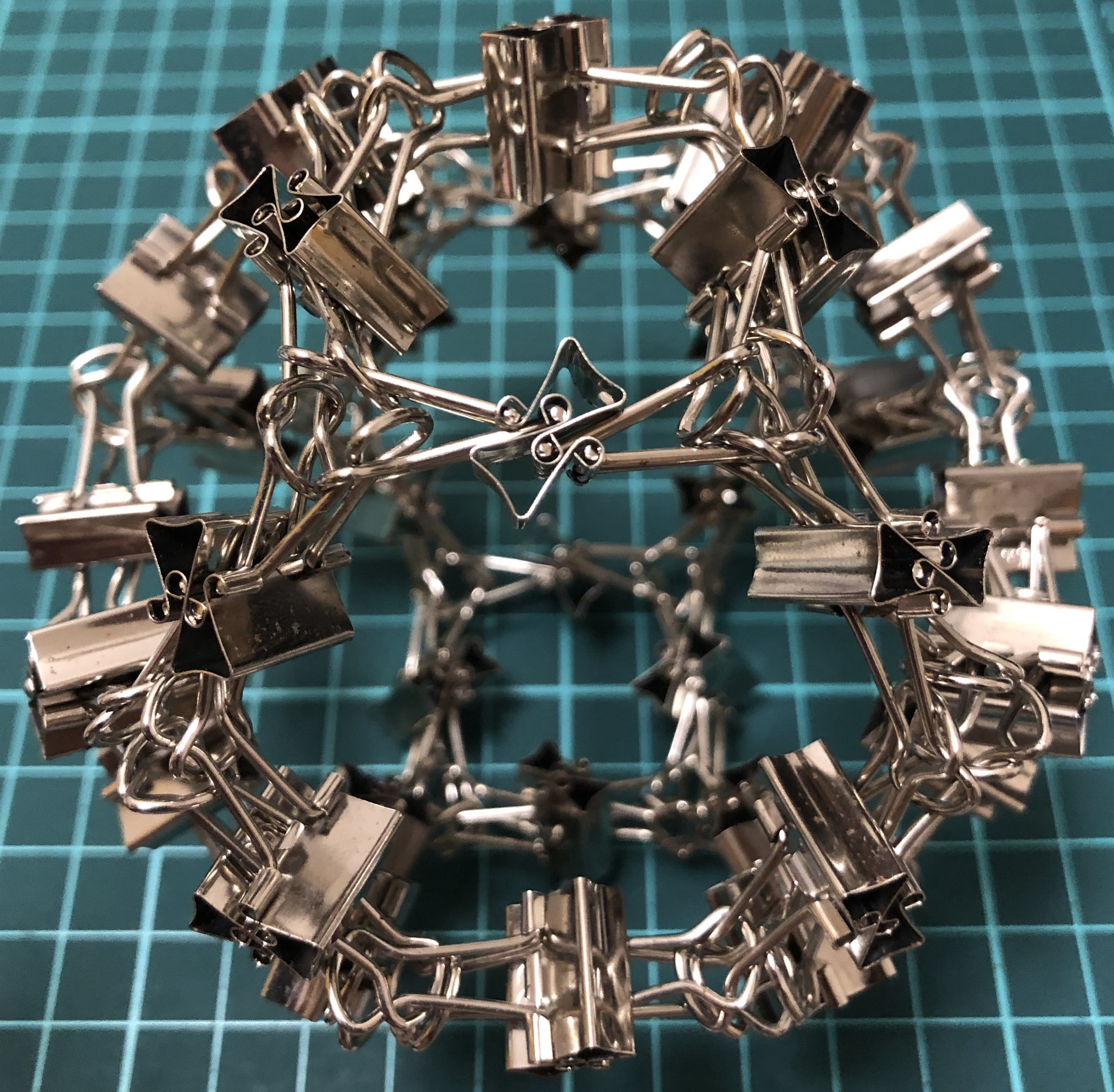↑ # Clips = 72
↑ Base = truncated octahedron
↑ Vertex config = 4.6.6
↑ Symmetry = cube’s rotations
I72-kC

#### I96-aaC↑ # Clips = 96
↑ Base = rhombicuboctahedron
↑ Vertex config = 3.4.4.4
↑ Symmetry = cube’s rotations
I96-jjC

#### I120-sC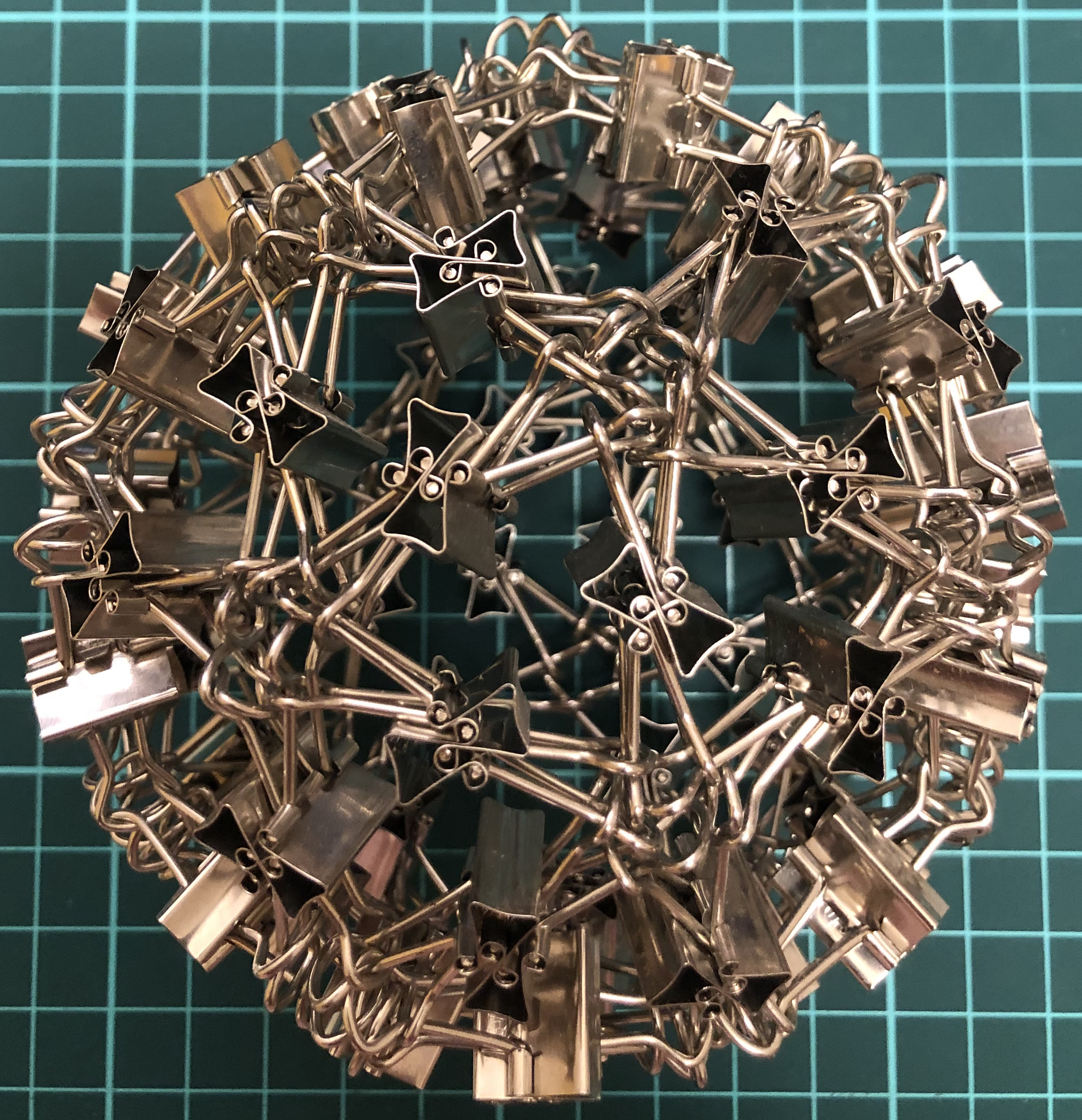↑ # Clips = 120
↑ Base = snub cube
↑ Vertex config = 3.3.3.3.4
↑ Symmetry = cube’s rotations
↑ (Dual = pentagonal icositetrahedron)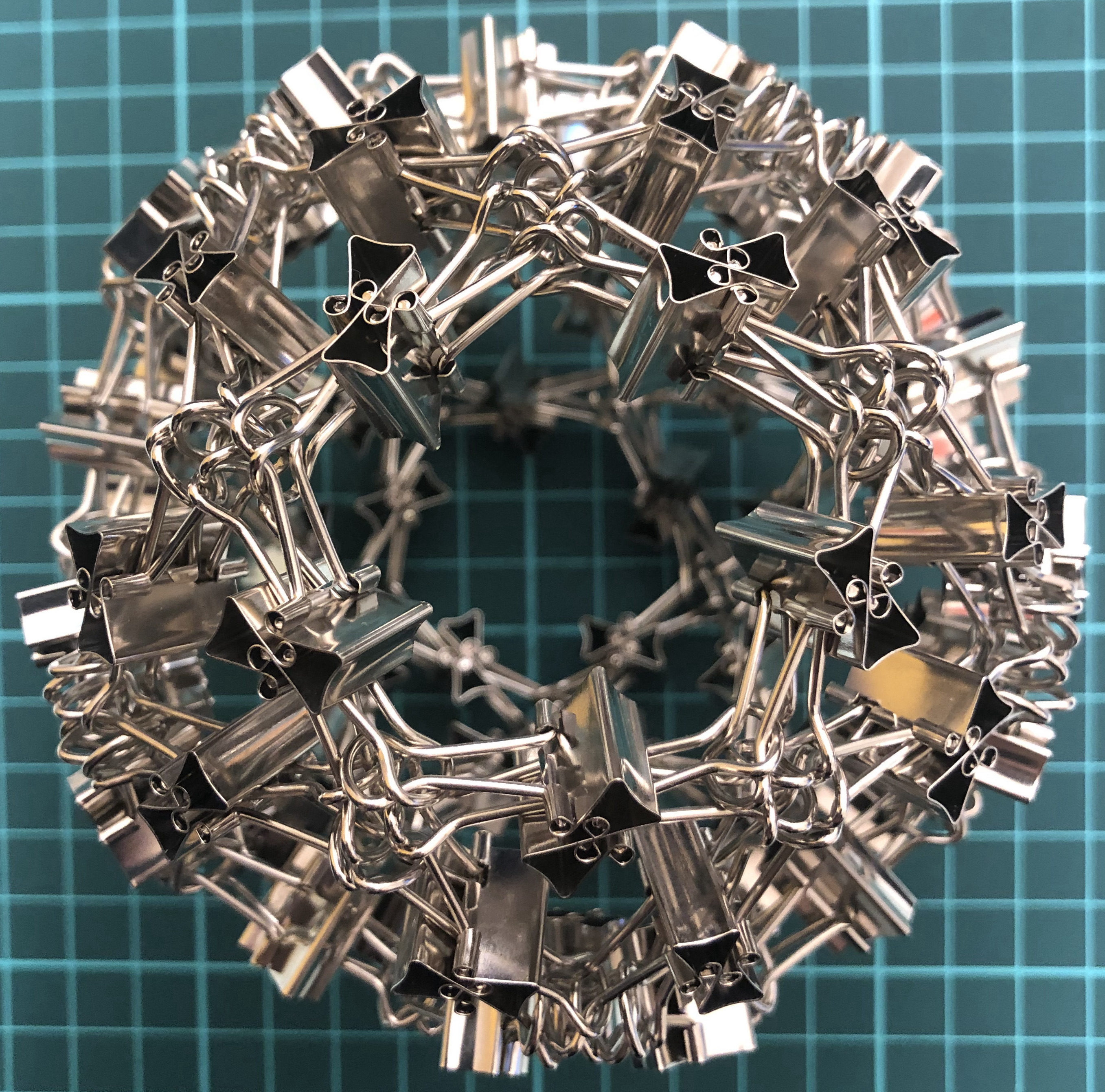↑ # Clips = 120
↑ Base = icosidodecahedron
↑ Vertex config = 3.5.3.5
↑ Symmetry = dodecahedron’s rotations
↑ Dual = I120-jD

#### I180-tI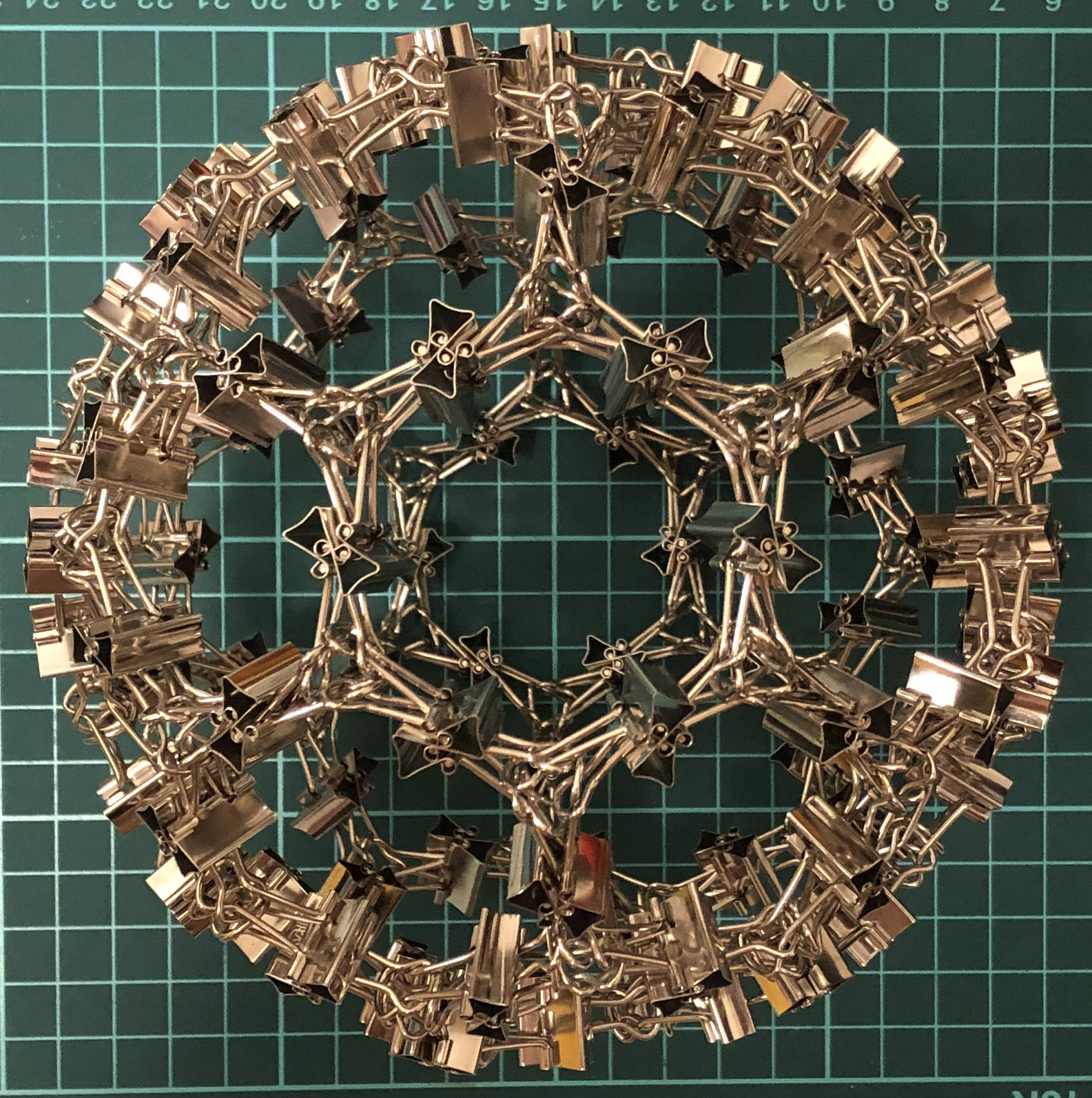↑ # Clips = 180
↑ Base = truncated icosahedron
↑ Vertex config = 5.5.6
↑ Symmetry = dodecahedron’s rotations
↑ Dual = I180-kD↑ # Clips = 240
↑ Base = rhombicosidodecahedron
↑ Vertex config = 3.4.5.4
↑ Symmetry = dodecahedron’s rotations

#### I300-sD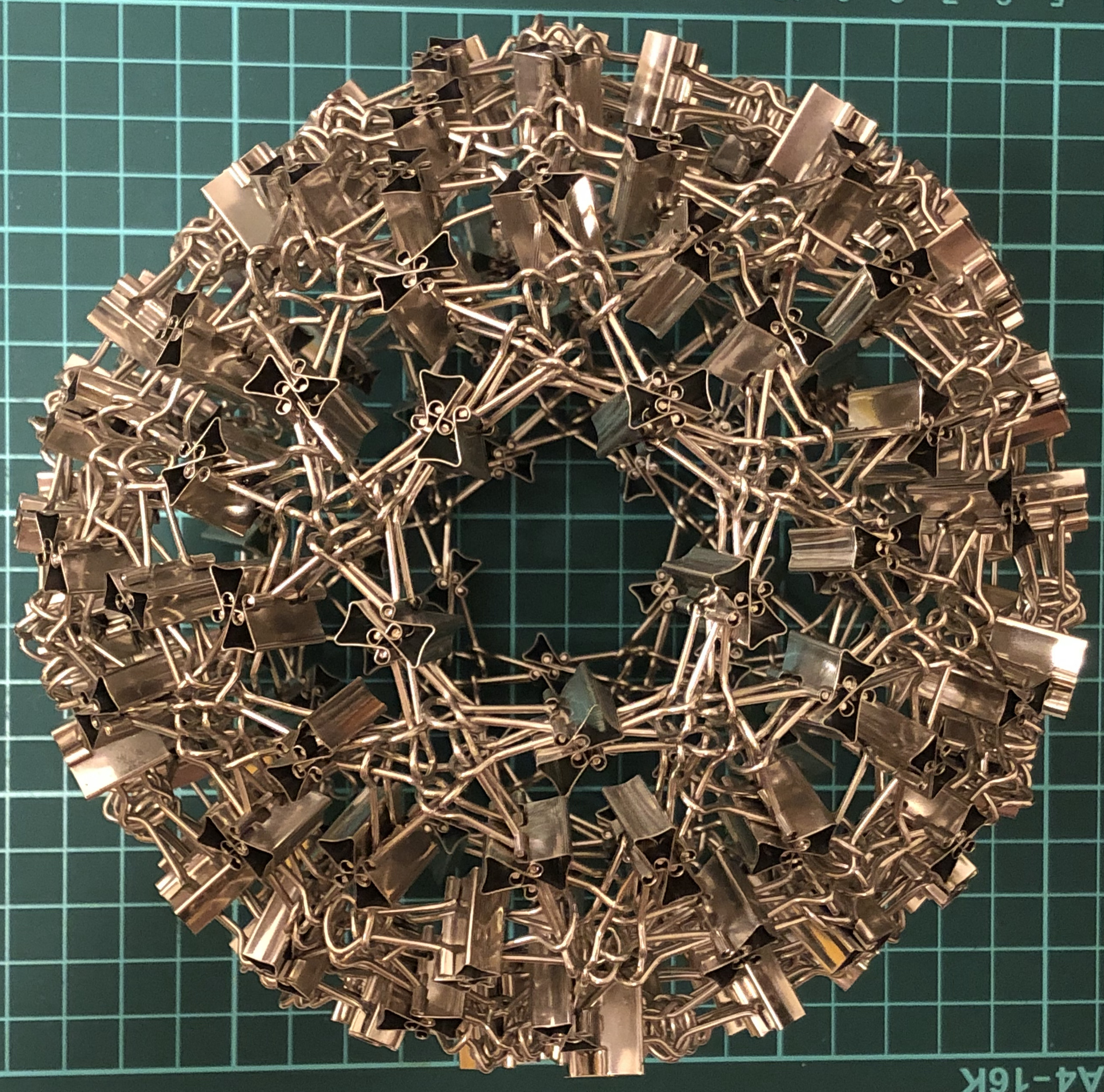↑ # Clips = 300
↑ Base = snub dodecahedron
↑ Face config = 3.3.3.3.5
↑ Symmetry = dodecahedron’s rotations
↑ (Dual = pentagonal hexecontahedron)

### I-series, Catalan

#### I36-kT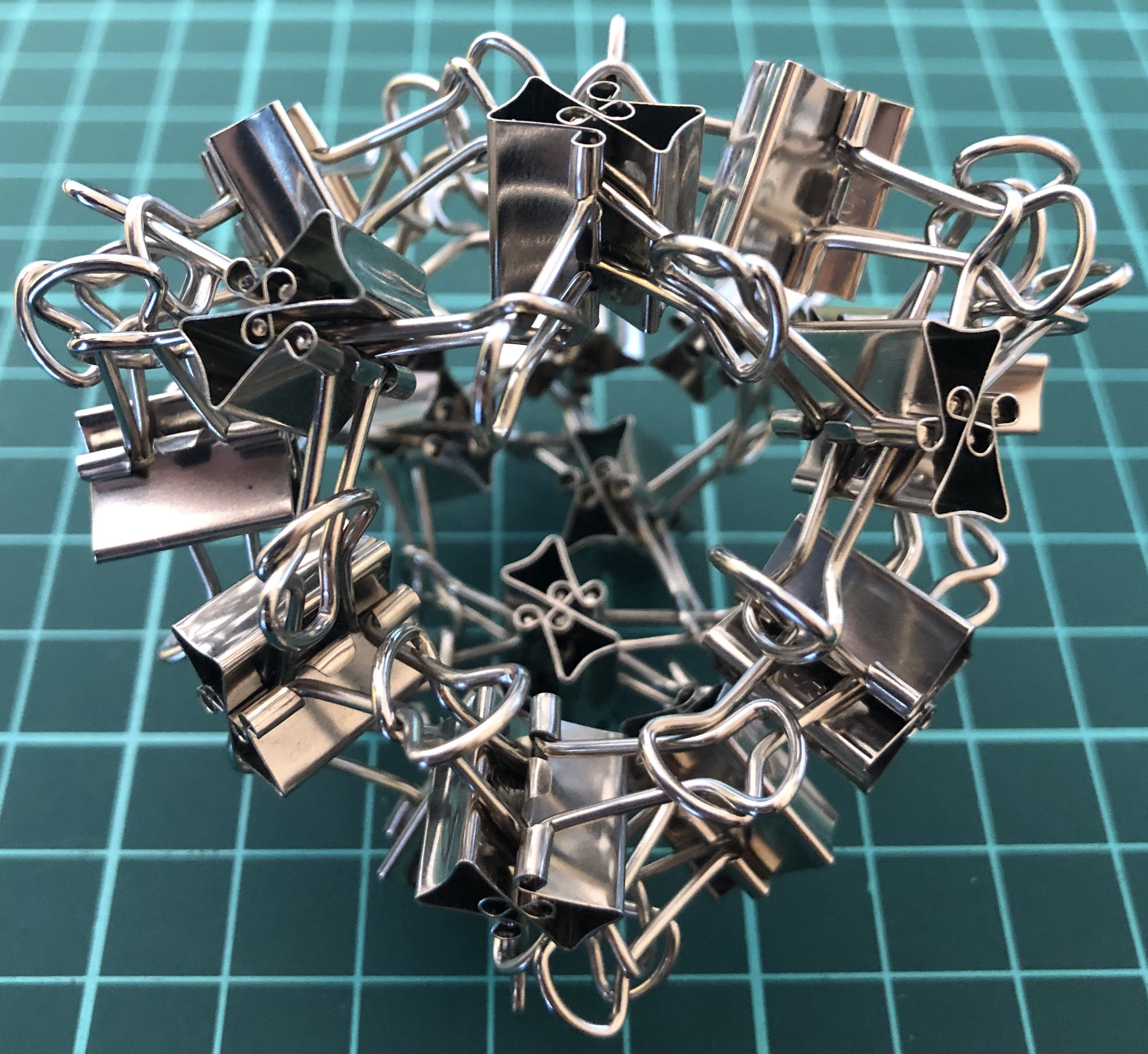↑ # Clips = 36
↑ Face config = 3.6.6
↑ Base = triakis tetrahedron
↑ Symmetry = tetrahedron’s rotations
↑ Dual = I36-tT

#### I48-jC↑ # Clips = 48
↑ Base = rhombic dodecahedron
↑ Face config = 3.4.3.4
↑ Symmetry = cube’s rotations
↑ Dual = I48-aC

#### I72-kC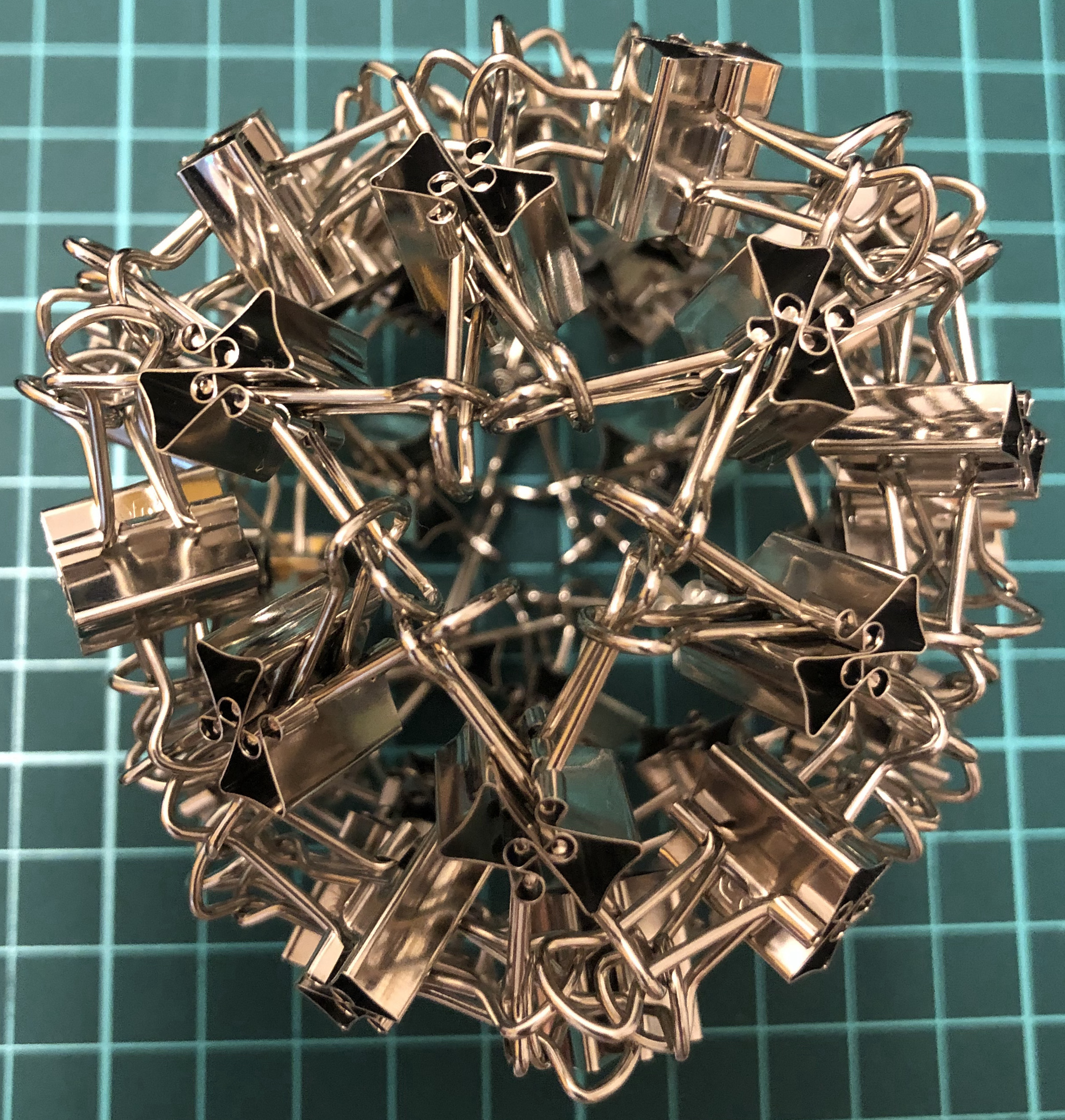↑ # Clips = 72
↑ Base = tetrakis hexahedron
↑ Face config = 4.6.6
↑ Symmetry = cube’s rotations
↑ Dual = I72-tO

#### I96-jjC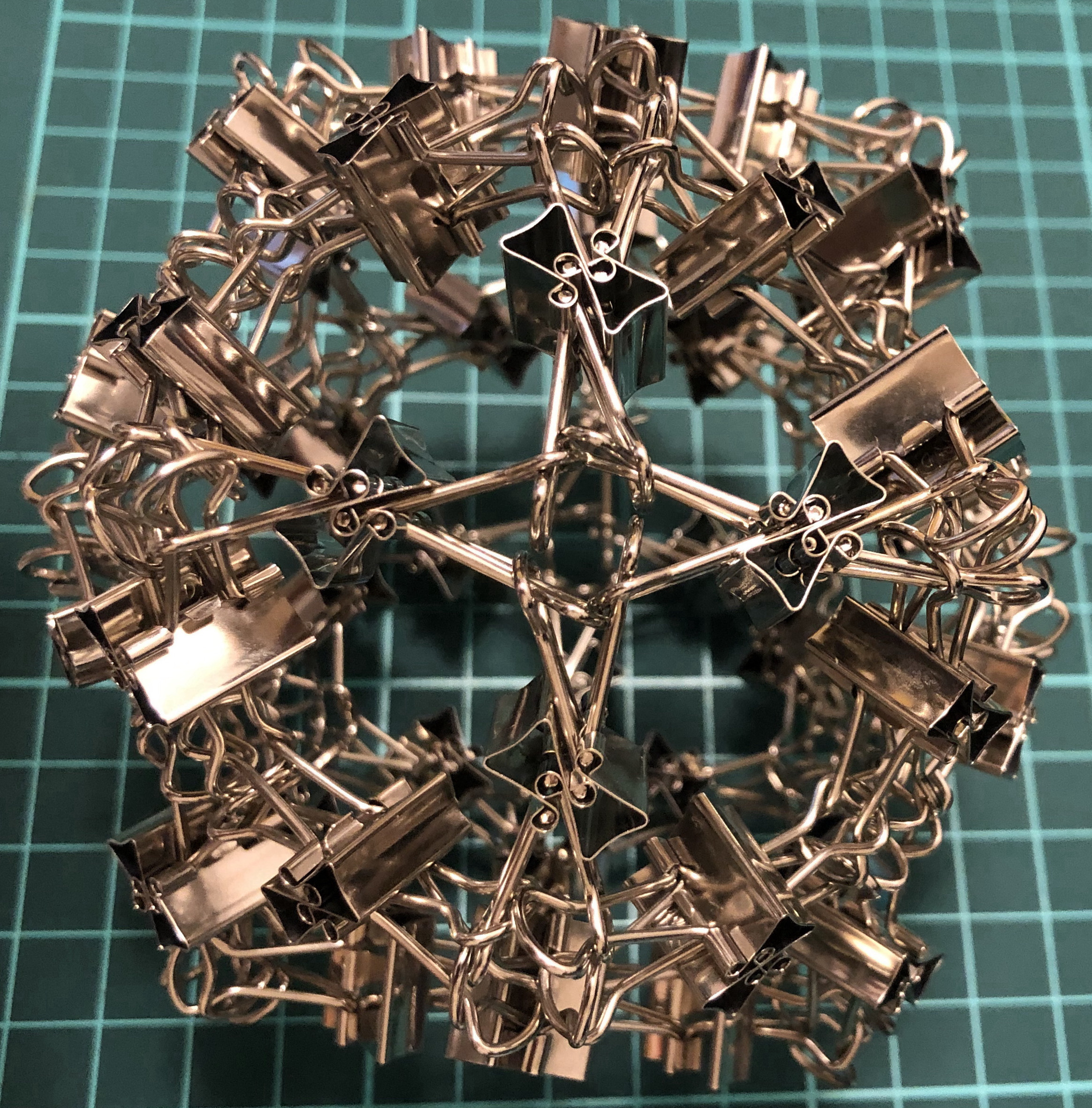↑ # Clips = 96
↑ Base = deltoidal icositetrahedron
↑ Face config = 3.4.4.4
↑ Symmetry = cube’s rotations
I96-aaC

#### I120-jD↑ # Clips = 120
↑ Base = rhombic triacontahedron
↑ Face config = 3.5.3.5
↑ Symmetry = dodecahedron’s rotations

#### I180-kD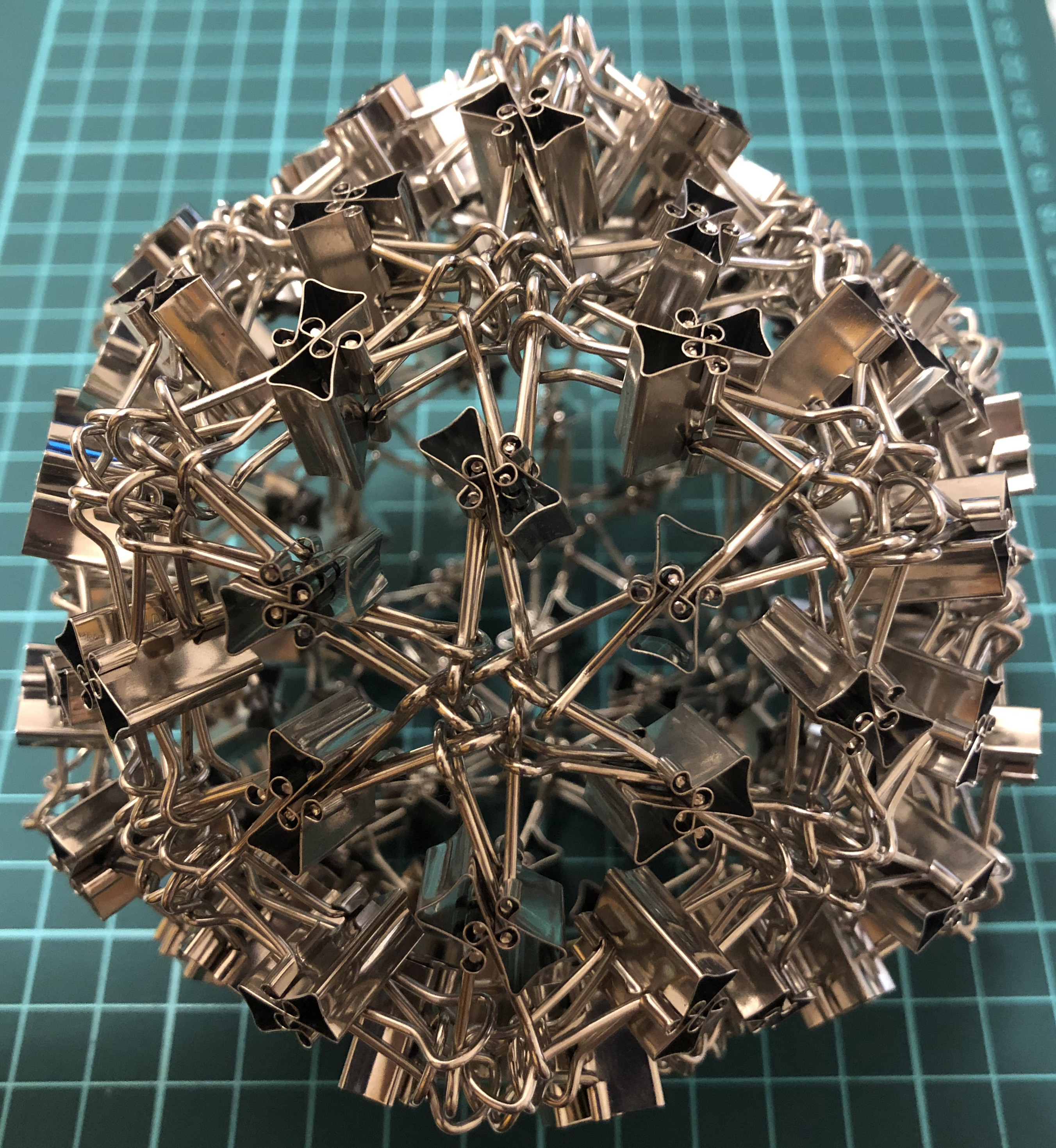↑ # Clips = 120
↑ Base = pentakis dodecahedron
↑ Face config = 5.6.6
↑ Symmetry = dodecahedron’s rotations
↑ Dual = I180-tI

### I-series, Fullerene

#### I240-cD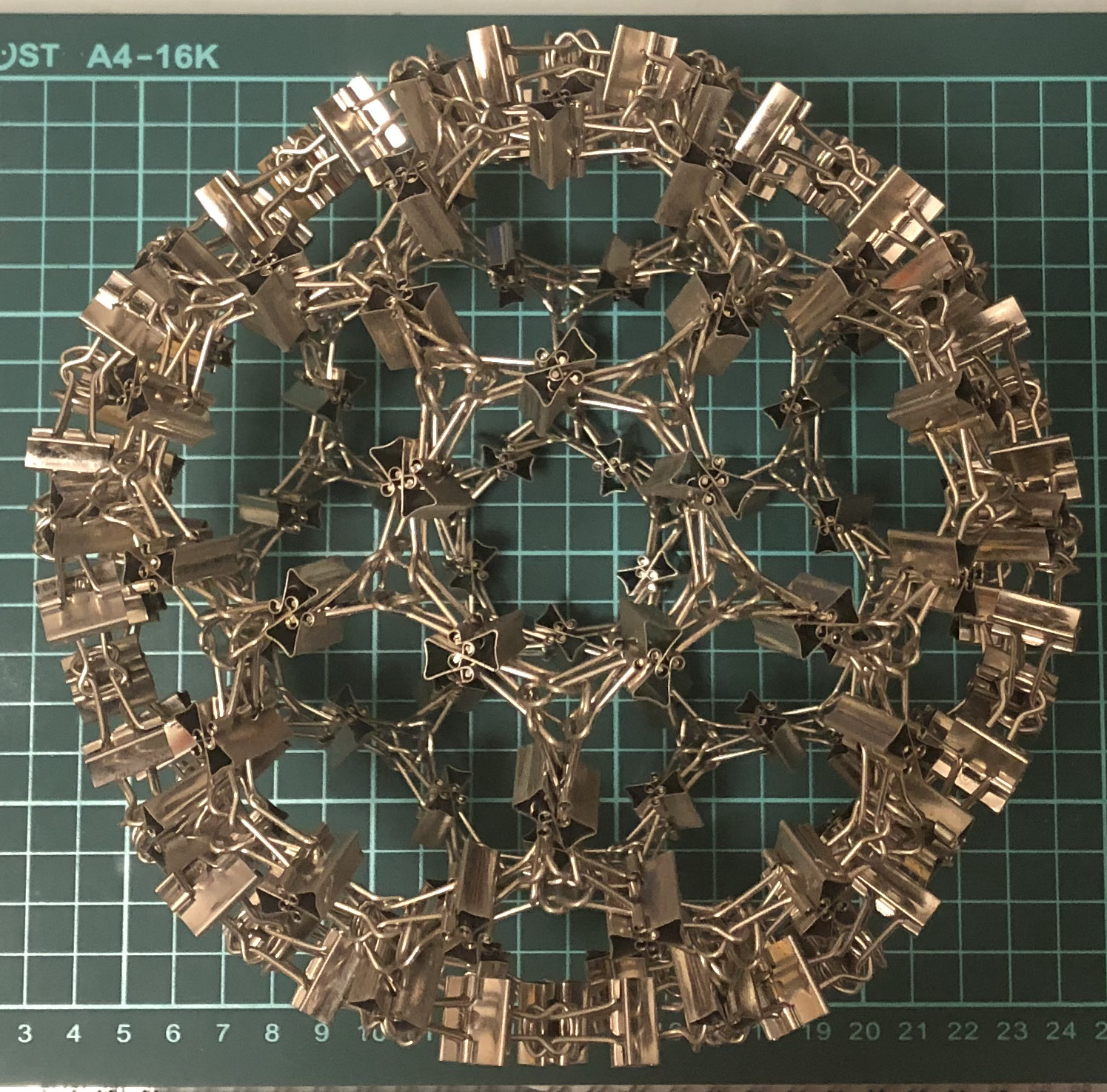↑ # Clips = 240
↑ Base = chamfered dodecahedron
↑ Each dodecahedron vertex = 4 new vertices
↑ Symmetry = dodecahedron’s rotations
↑ Dual = I240-uI

#### I240-uI↑ # Clips = 240
↑ Base = pentakis icosidodecahedron aka C80
↑ Each icosahedron face = 4 small triangles
↑ Symmetry = dodecahedron’s rotations
↑ Dual = I240-cD

### W-series

#### W12-T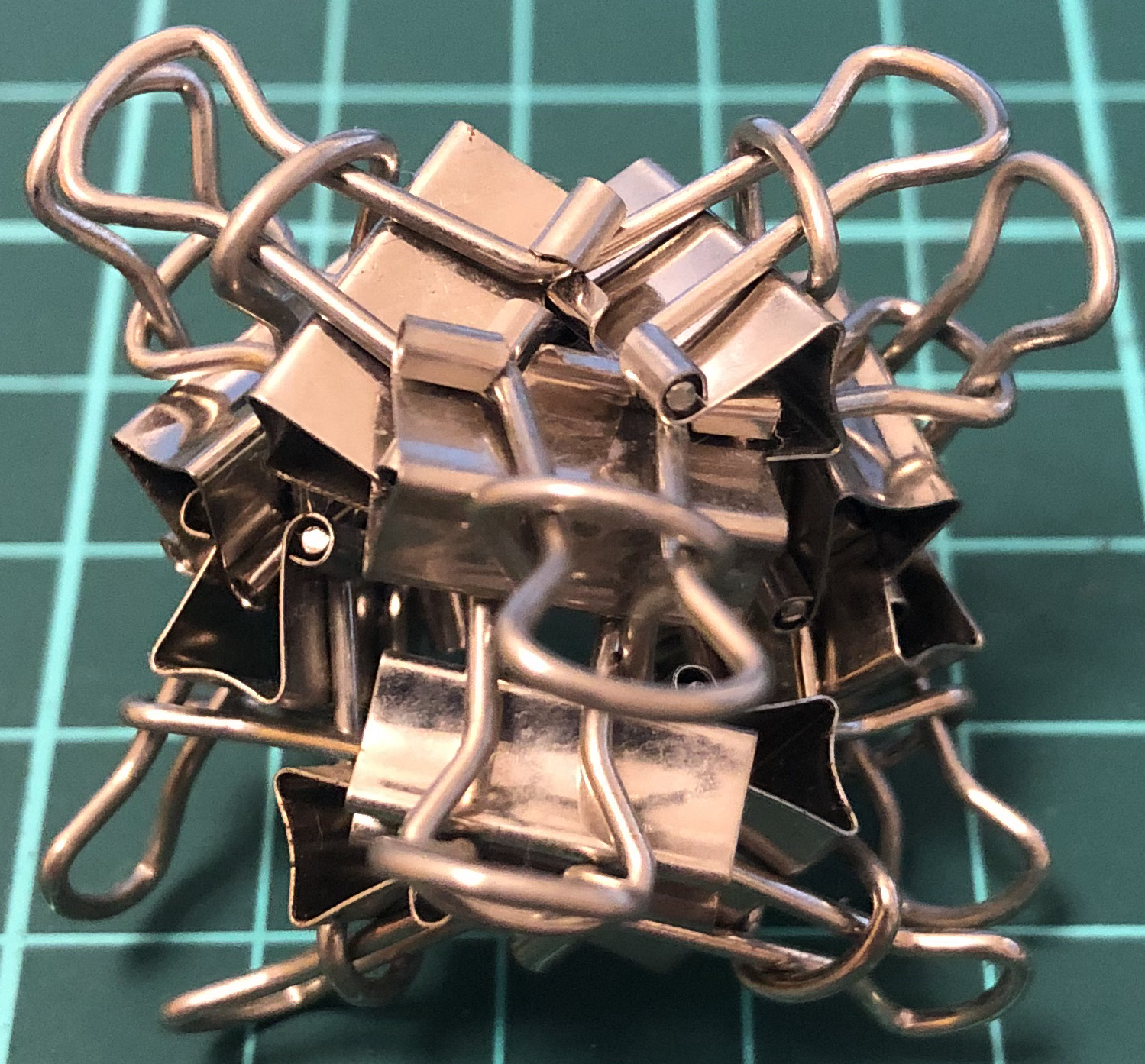↑ # Clips = 12
↑ Base = tetrahedron
↑ Vertex config = 3.3.3
↑ Symmetry = tetrahedron’s rotations
↑ Dual = itself

#### W24-C

Difficulty encountered
↑ # Clips = 24
↑ base = cube
↑ Vertex config = 4.4.4
↑ Symmetry = cube’s rotations
↑ dual = W24-O

#### W24-O↑ # Clips = 24
↑ base = octahedron
↑ Vertex config = 3.3.3.3
↑ Symmetry = cube’s rotations
↑ dual = W24-C

#### W60-D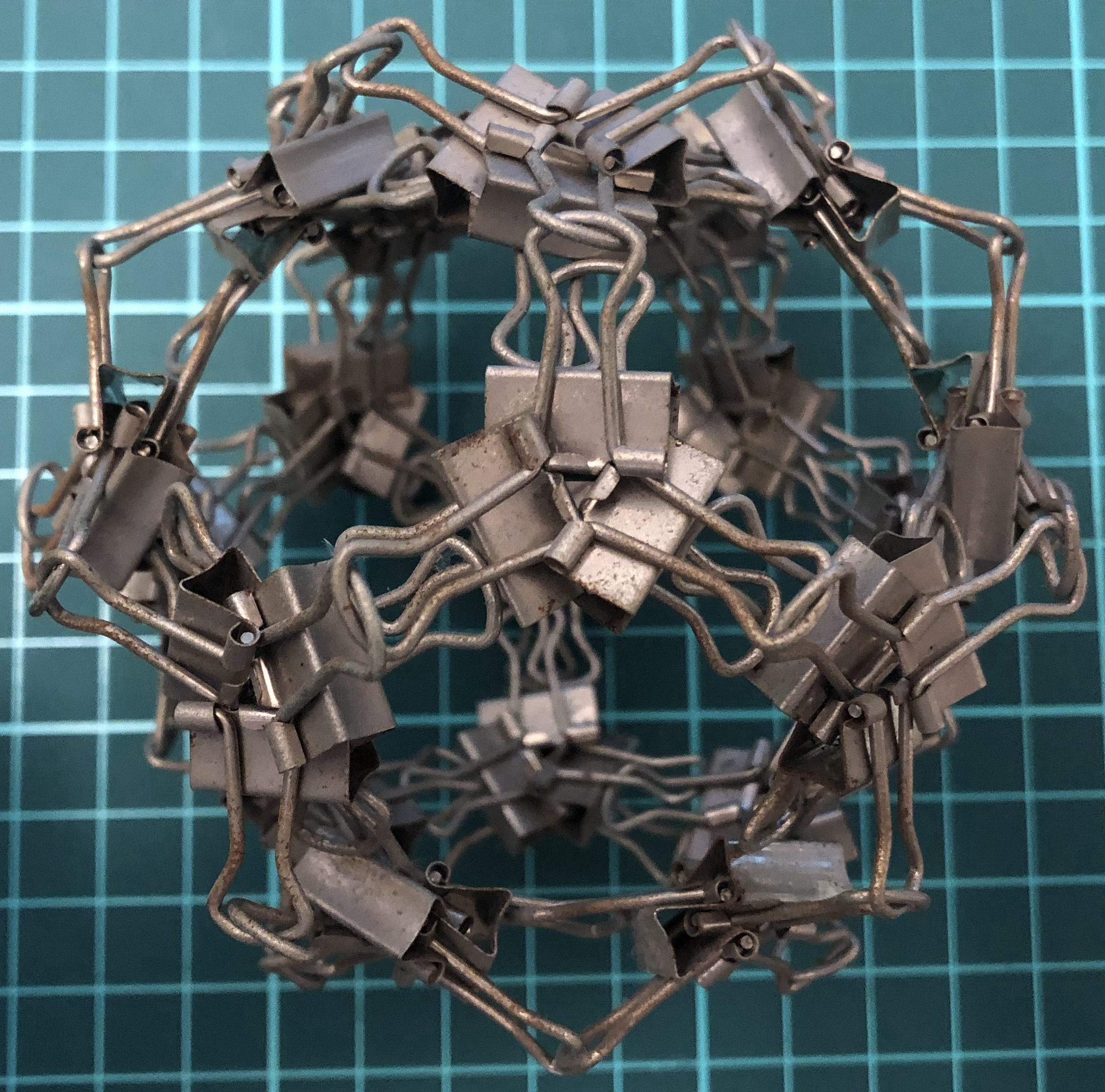↑ # Clips = 60
↑ Base = dodecahedron
↑ Vertex config = 5.5.5
↑ Symmetry = dodecahedron’s rotations
↑ Dual = W60-I

#### W60-I↑ # Clips = 60
↑ Base = icosahedron
↑ Vertex config = 3.3.3.3.3
↑ Symmetry = dodecahedron’s rotations
↑ Dual = W60-D

#### W36-tT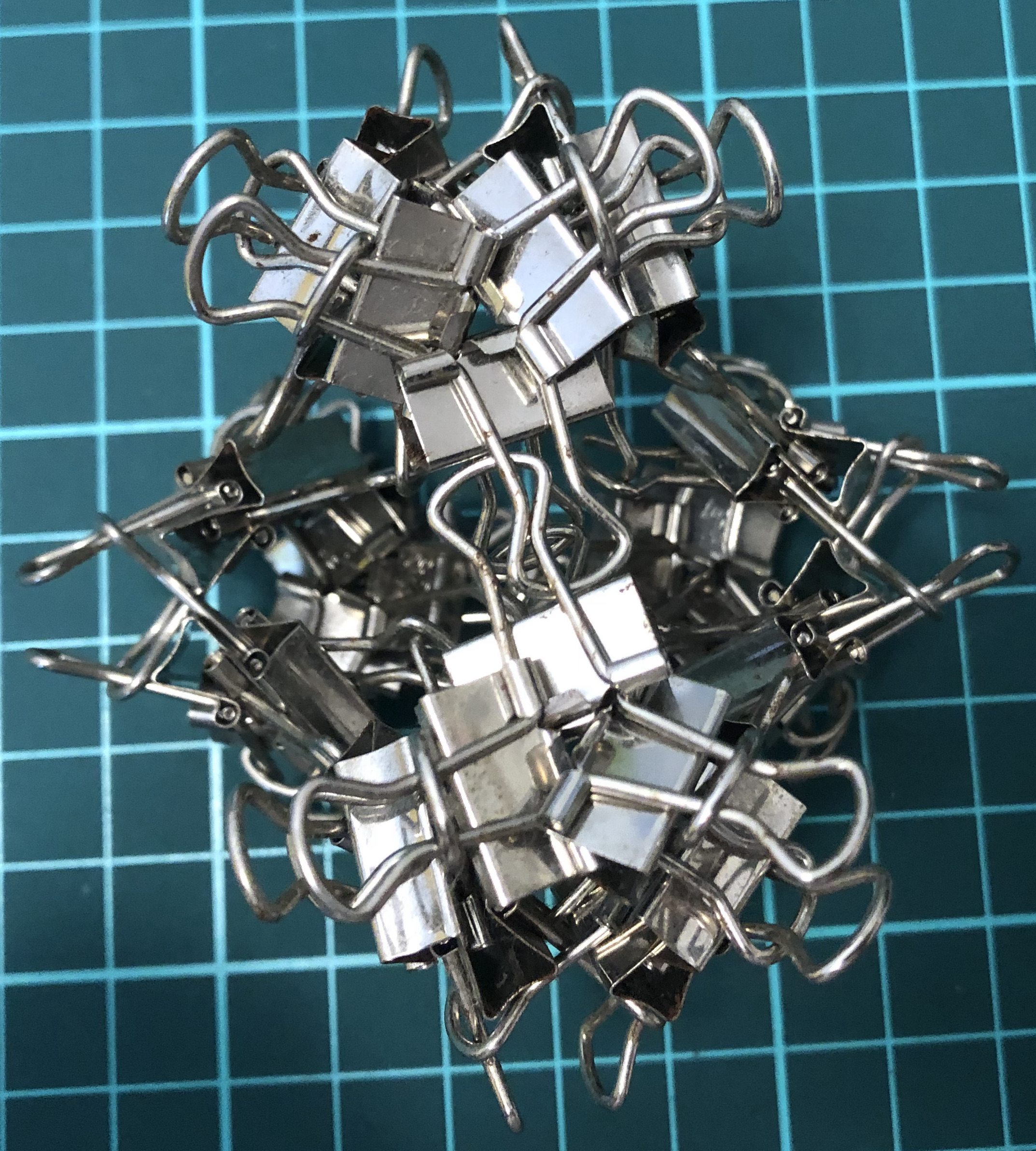↑ # Clips = 36
↑ Base = truncated tetrahedron
↑ Vertex config = 3.6.6
↑ Symmetry = tetrahedron’s rotations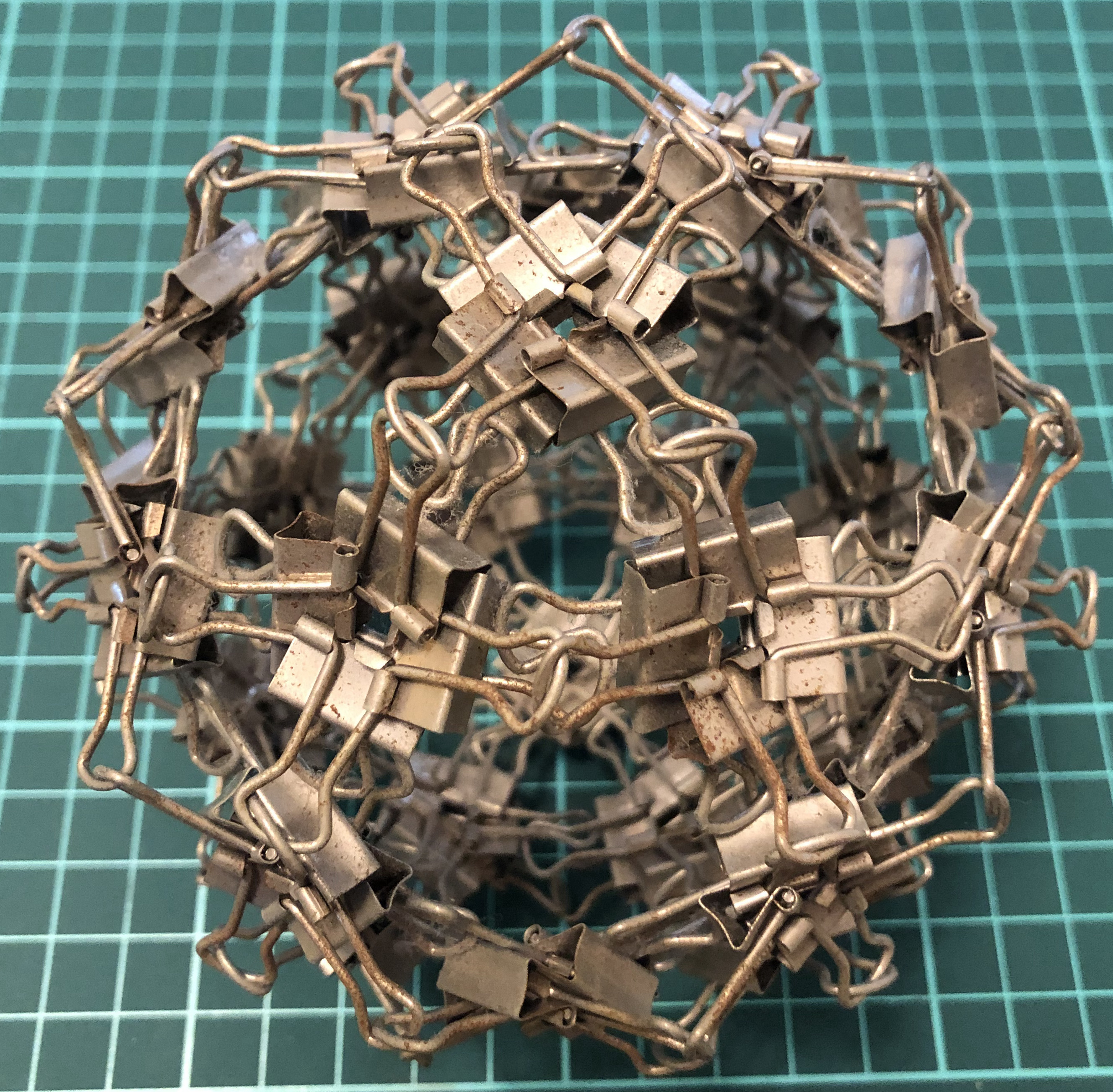↑ # Clips = 120
↑ Base = icosidodecahedron
↑ Vertex config = 3.5.3.5
↑ Symmetry = dodecahedron’s rotations

#### W240-cD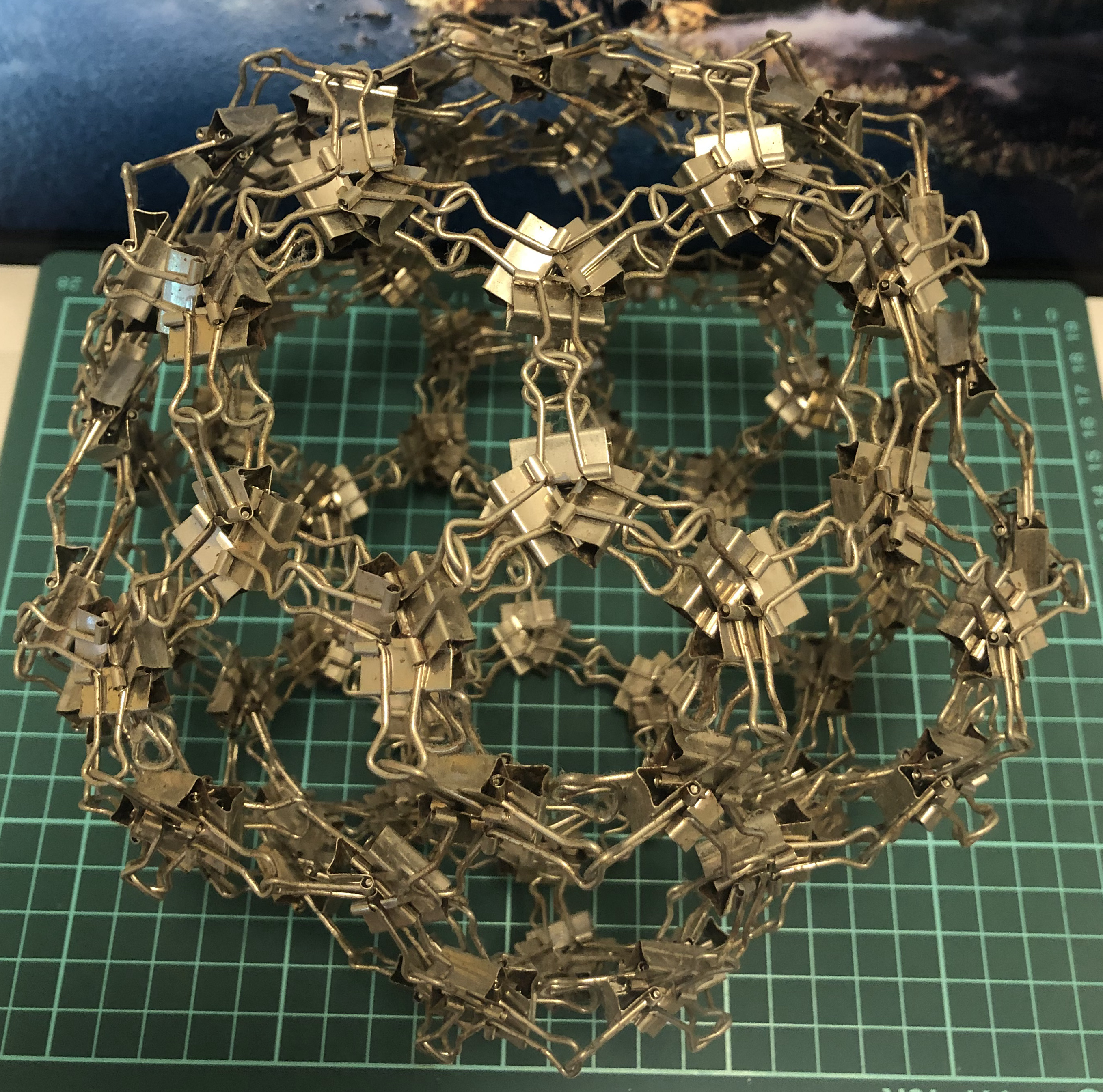↑ # Clips = 240
↑ Base = chamfered dodecahedron
↑ Each dodecahedron vertex = 4 new vertices
↑ Symmetry = dodecahedron’s rotations